• 摘 要： 本文首先介绍了真有效值数字电压表的基本原理，然后阐述LTC1966 TRMS／DC转换器工作原理，最后给出由LTC1966构成的多量程真有效值数字电压表电路。关键词： 真有效值；TRMS／DC转换器；D-S调制器；数字电压...
• TDK-Lambda公司最新推出DC-DC转换器PX系列。全隔离的PXA和PXB系列具有1吋x1吋的小型占位面积，设计用于从通信到工厂自动化的设备。可提供开架式(PXA)和屏蔽金属外壳(PXB)两种型号。PXA系列具有单输出电压，标称输入...
• 该扩展允许用户基于千克价格计算每...我们的平方米价格转换器可用于计算大约每公斤或平方米的价格。 计算得出的价格仅供参考。 蒂森克虏伯材料（英国）对平方米价格转换器的依赖不承担任何责任。 支持语言:English (UK)生产工具
• 新模组的1平方英寸的基板，以高达86%的效率水平提供完全15W的输出功率，而且能节省空间、兼容端子，可完美替换1英寸 ×2英寸的旧DC/DC转换器。 　BEI15系列为直插式的PCB贴装，提供±5V, ±12V 和 ±15V的双输出...
• 　考虑到上述问题，凌力尔特决定采用系统级封装 （SiP） 技术来开发占板面积约为 1/2 平方英寸 （仅刚刚超过 3cm2） 的接收。接收的边界处有 50Ω RF输入、50Ω LO 输入、ADC 时钟输入及数字 ADC 输出。该边界...
• 这款高效的电源管理集成电路 (IC) 是首款尺寸仅为 13 平方毫米的 6 MHz、500 mA 转换器，实现了高度仅为 0.6 毫米的超薄解决方案。更多详情，敬请访问：www.ti.com.cn/tps62601-pr。 　全新 TPS62601 转换器利用 ...
• 模数转换器性能参数是判定器件性能优劣的重要参考，因此了解性能参数对硬件选型来说是很关键的哦，本文对A/D转换器性能参数做一个概述，主要是从静态参数和动态参数两个方面出发。 2、静态参数 2.1 分辨率 分辨率...
1、概述
模数转换器性能参数是判定器件性能优劣的重要参考，因此了解性能参数对硬件选型来说是很关键的哦，本文对A/D转换器性能参数做一个概述，主要是从静态参数和动态参数两个方面出发。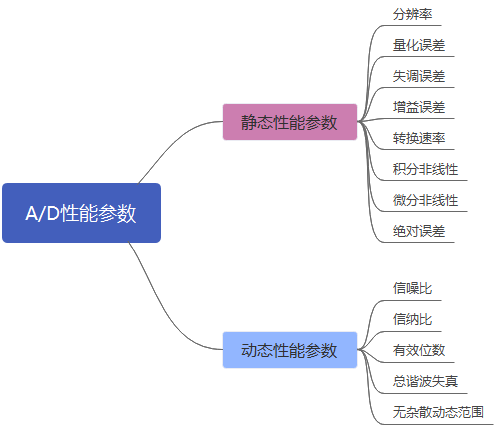2、静态参数
2.1 分辨率
2.2 转换速率
2.3 量化误差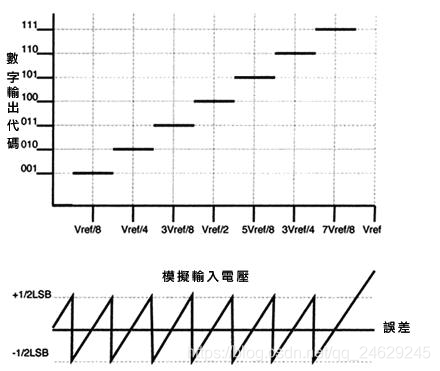2.4 失调误差
失调误差是描述模数转换器零输入情况下的输出偏移，这导致其和理想量化输出曲线右移一段距离，右移的电压偏移即为失调误差，测量时将数字输出”0“转换得到的电压与理论零点电压作比较可以得到失调误差。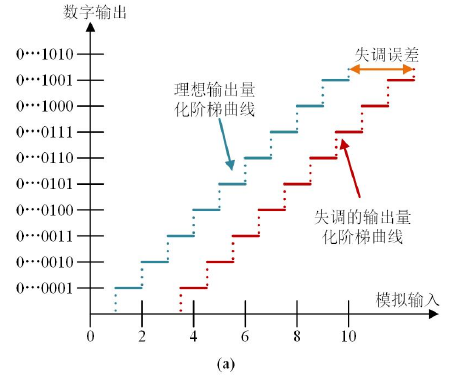2.5 增益误差
增益误差是指输出量化阶梯曲线的直线斜率对比理想输出量化阶梯曲线的直线斜率而存在的斜率误差。表示增益斜率的度量方式是输出满刻度数字码对应的模拟量和 DAC 满量程的模拟范围比值，理想模数转换器的增益斜率为 1，所以增益误差也可以表示为产生满刻度数字码对应的模拟量和参考电压之间的差值。测试时需要采用代码平均法即输入不同电压信号分别得到不同的数字信号，将数字信号求均值，测量得到均值数字信号时的电压输入，得到一组“点”，然后测试零数字信号时的电压点即可求得另一组“点”，两点即可求得斜率变化。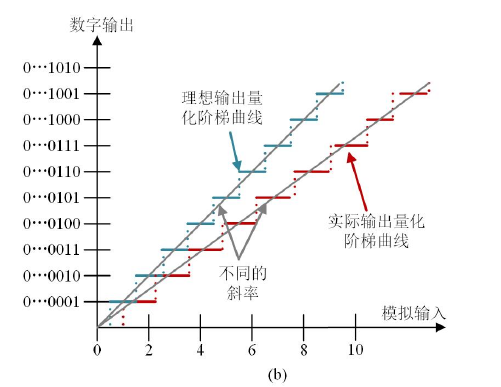2.6 微分非线性
微分非线性误差是模数转换器的实际转换阶梯度量和理想转换阶梯度量的差值，理论上一个代码转换点到下一个代码转换点之间的模拟电压差是恒定的，即1LSB=Vref/2^n，但是实际上1LSB码宽之间的电压差也存在差距哦，理解的话可以以尺子为例，尺子的最小刻度是1mm，那么每个1mm都是精确的1mm么？由于工艺等原因，尺子刻度1mm有可能是0.98mm，1.01mm等。测量时相邻转换点的位置作减法可测得 DNL，假定(k+1)a 和ka 分别是相邻两个数字输出码 k +1 和 k 对应的模拟电压值，此时二进制码 k 跳变成 k +1时的度量为Δ(k)=a(k+1)-ak，得到微分非线性表达式：DNL=(Δ(k)-1LSB)/1LSB，要保证没有丢码和单调的转移函数，ADC的DNL必须小于1LSB。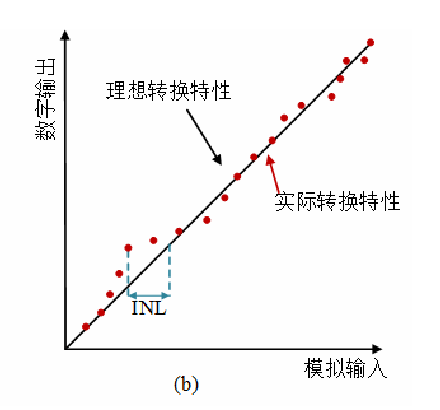2.7 积分非线性
积分非线性误差是模数转换器所有输出数字码对应的模拟电压值与实际采样的模拟电压值的最大差值，也被称为输出数值偏离线性的最大距离，是 DNL 误差累积的结果，一般采用 LSB为标准单位度量，若考虑失调误差Vzero，输出数字码D时对应的模拟电压Vd，得到的积分非线性误差INL=(Vd-Vzero)/1LSB-D。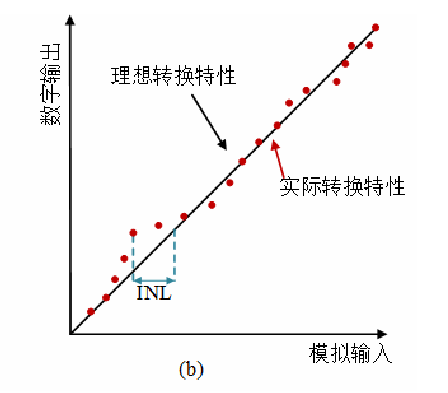2.8 绝对误差
某些 A/D 转换器还定义了绝对误差，它是失调误差、增益误差和积分非线性误差的总和。也可以这样阐述，它是未进行增益或失调误差补偿时，实际 A/D 传递函数与理想线性传递函数之间的偏差。因此，绝对误差也称为未经调整的总误差。
3、动态参数
3.1 信噪比
信噪比是信号功率和总噪声功率（包括量化噪声，热噪声，白噪声等电路噪声）之比。信噪比的功率噪声是针对整个奈奎斯特采样区间内的噪声，其在第二奈奎斯特区间内略有下降，但是整体几乎是恒定不变的。只考虑量化噪声的情况下SNR = 6.02N + 1.76dB，考虑奈奎斯特带宽内噪声，但是在实际使用中信号有效带宽往往小于fs/2，因此如果再后级采用数字滤波器滤去带宽外噪声，这样可以提高信噪比，公式如下图所示，信噪比受到输入信号频率，采样频率，ADC 量化位数和输入信号幅值的影响，与输入信号的幅值成比例关系。提高 ADC 的采样频率，增加 ADC 的量化位数，可以有效提高信噪比，公式推导如ADC/DAC信噪比计算。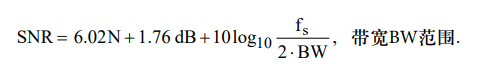3.2信纳比
3.3 有效位数3.4 总谐波失真
总谐波失真加噪声 （THD+N）是转换器产生的各次谐波和噪声有效值的均方根与接近满量程的正弦输入信号的均方根之间的比值。THD+N 不一定包含 FFT 分析中的所有数据。对于一个有效的 THD + N 规范，必须指定噪声带宽。如果噪声带宽占用了转换器的整个可用带宽 （0 - fs/2），那么 THD+N 测量值就与 SINAD 具有相同的结果。
3.5 无杂散动态范围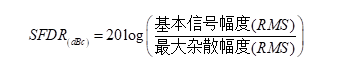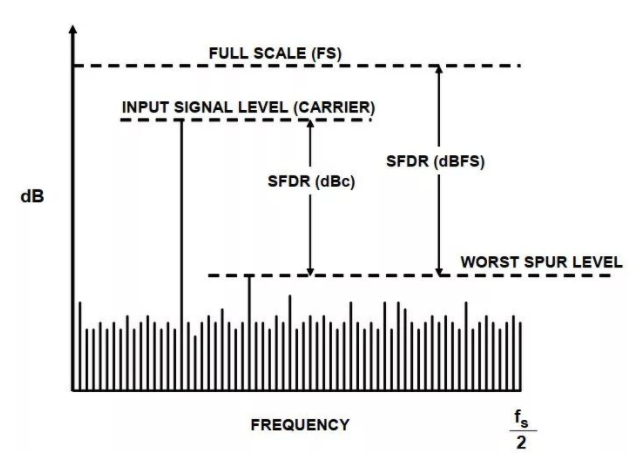ADC动态范围与其有效位数有关，ADC最小能够表示的数是1bit，最大可以表示的数是2^ n，动态范围=20log(最大的数/最小的数)，对于一个16位ADC而言，20log(2^16/1)=96.33db，但是通常有效位数都是小于16bit的哈，如TI的16位ADC ADS54J60，在不同输入频率和功率下有效位数不同，实际使用时需要根据有效位数计算动态范围。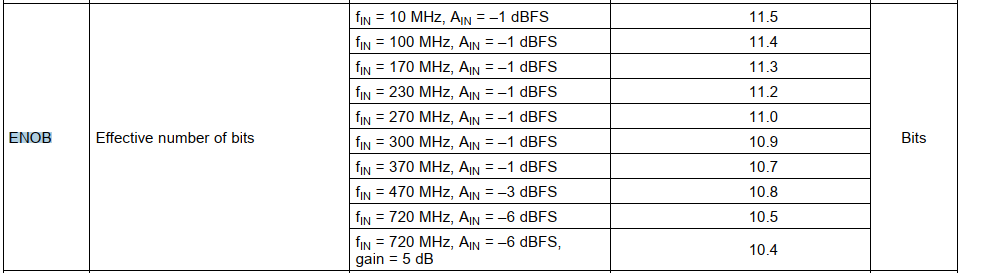结尾分割。。。。。。。。
参考文献
https://ez.analog.com/cn/data-converters/f/forum/96676/adc https://www.analog.com/media/cn/training-seminars/tutorials/MT-003_cn.pdf http://ww1.microchip.com/downloads/cn/AppNotes/00693A_CN.pdf 杨静萱. 逐次逼近型模数转换器（SAR ADC）关键技术研究[D].电子科技大学,2020.
展开全文fpga
• DC/DC转换器TPS63000可在1.8V至5.5V的电压范围内实现高达96%的效率，并能够同时输出达1.2A的输出电流。 　TI负责便携式电源管理业务的副总裁Dave Heacock指出：“便携式应用设计师在如何进一步提高电池利用率方面...
• 单位转换器旗舰版 一个适用于Android的简单，轻巧，以Material Design为主题的单位转换器。 精美的Material Design用户界面允许从一个单元中的数字快速轻松地转换为另一单元。 我们的目标是保持简单-不会因过多的...Java
• 交流电压的真有效值是通过电路对输入交流电压进行“平方→求平均值→开平方”的运算而得到的。真有效值仪表的最大优点是能够精确测量各种电压波形的有效值，而不必考虑被测波形的参数以及失真。随着集成电路的迅速...
• 该扩展允许用户在英制和公制之间转换尺寸...我们的公制和英制转换器可用于计算大约每公斤或平方米的价格。 计算结果仅供参考。 蒂森克虏伯材料（英国）对公制和英制转换器的依赖不承担任何责任。 支持语言:English (UK)生产工具
• 填空题：1、2 例题及上机实践：2~5 案例研究：21点扑克牌游戏 第七章 错误和异常处理 几个例题 案例研究：使用调试调试Python程序 第八章 函数和函数式编程 一些知识点总结和几个例题 选择题：1~5 思考题：4~11 ...
（还在更新中…） 这篇博客花费了我的大量时间和精力，从创作到维护；若认可本篇博客，希望给一个点赞、收藏
并且，遇到了什么问题，请在评论区留言，我会及时回复的

这本书对Python的知识点的描述很详细，而且排版看的很舒服

几个例题： 假装自己从零开始学，将一些有代表性、有意思的例题抽取出来部分复习题： 遇到有意思的复习题，我会拿出来，并且进行分析上机实践： 全部上机实践题的解题思路

文章目录
第一章 Python概述几个例题
第二章 Python语言基础选择题：1、3、7、8思考题：9上机实践：2~6案例研究：使用Pillow库处理图像文件
第三章 程序流程控制几个例题选择题：1、2、3填空题：6思考题：3~6上机实践：2~14案例研究：使用嵌套循环实现图像处理算法
第四章 常用内置数据类型几个例题选择题：11填空题：4、7、8、9、10、13、21思考题：5上机实践：2~14案例研究：科学计算和数据分析
第五章 序列数据类型几个例题选择题：4、5、7、11、12填空题：1、5、6、12、13、14思考题：2、3、5上机实践：2~6案例研究：猜单词游戏
第六章 输入和输出几个例题填空题：1、2例题及上机实践：2~5案例研究：21点扑克牌游戏
第七章 错误和异常处理几个例题案例研究：使用调试器调试Python程序
第八章 函数和函数式编程一些知识点总结和几个例题选择题：1~5思考题：4~11上机实践：2~5案例研究：井字棋游戏
第九章 面向对象的程序设计第十章 模块和客户端第十一章 算法与数据结构基础第十二章 图形用户界面案例研究：简易图形用户界面计算器
第十三章 图形绘制图形绘制模块：tkinter图形绘制模块：turtle
第十四章 数值日期和时间处理第十五章 字符串和文本处理第十六章 文件和数据交换第十七章 数据访问第十八章 网络编程和通信第十九章 并行计算：进程、线程和协程第二十章 系统管理

第一章 Python概述

几个例题
一：Python3.7.4下载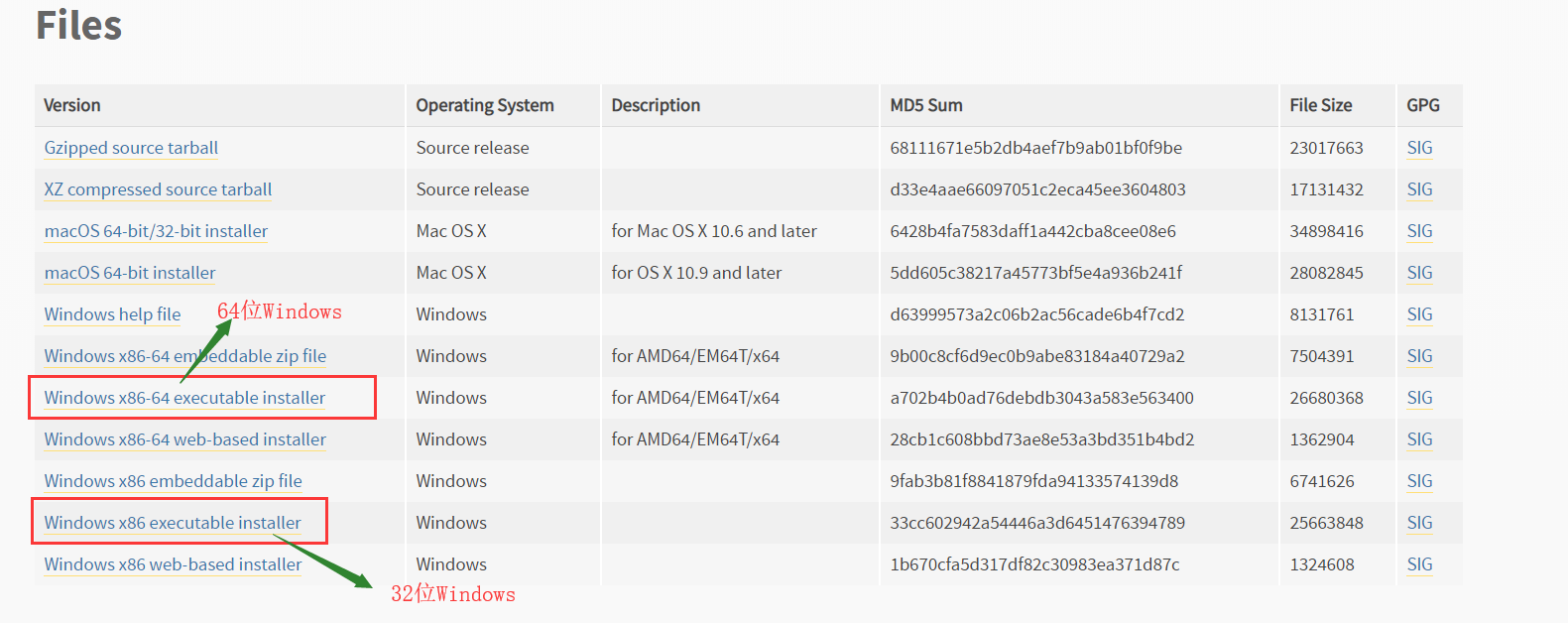下载，安装完python后：出现的四个玩意：Python 3.7 Module Docs，IDLE，Python 3.7 Manuals，Python 3.7（64-bit）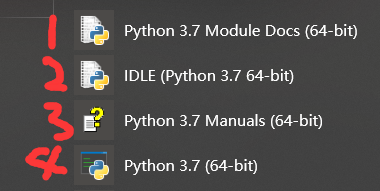Python 3.7 Module Docs（64-bit） 点击之后，会出现一个网页（将我下载的Python3.7.4文件夹中包含的模块都列了出来,页面不止这么点，还可以往下拉）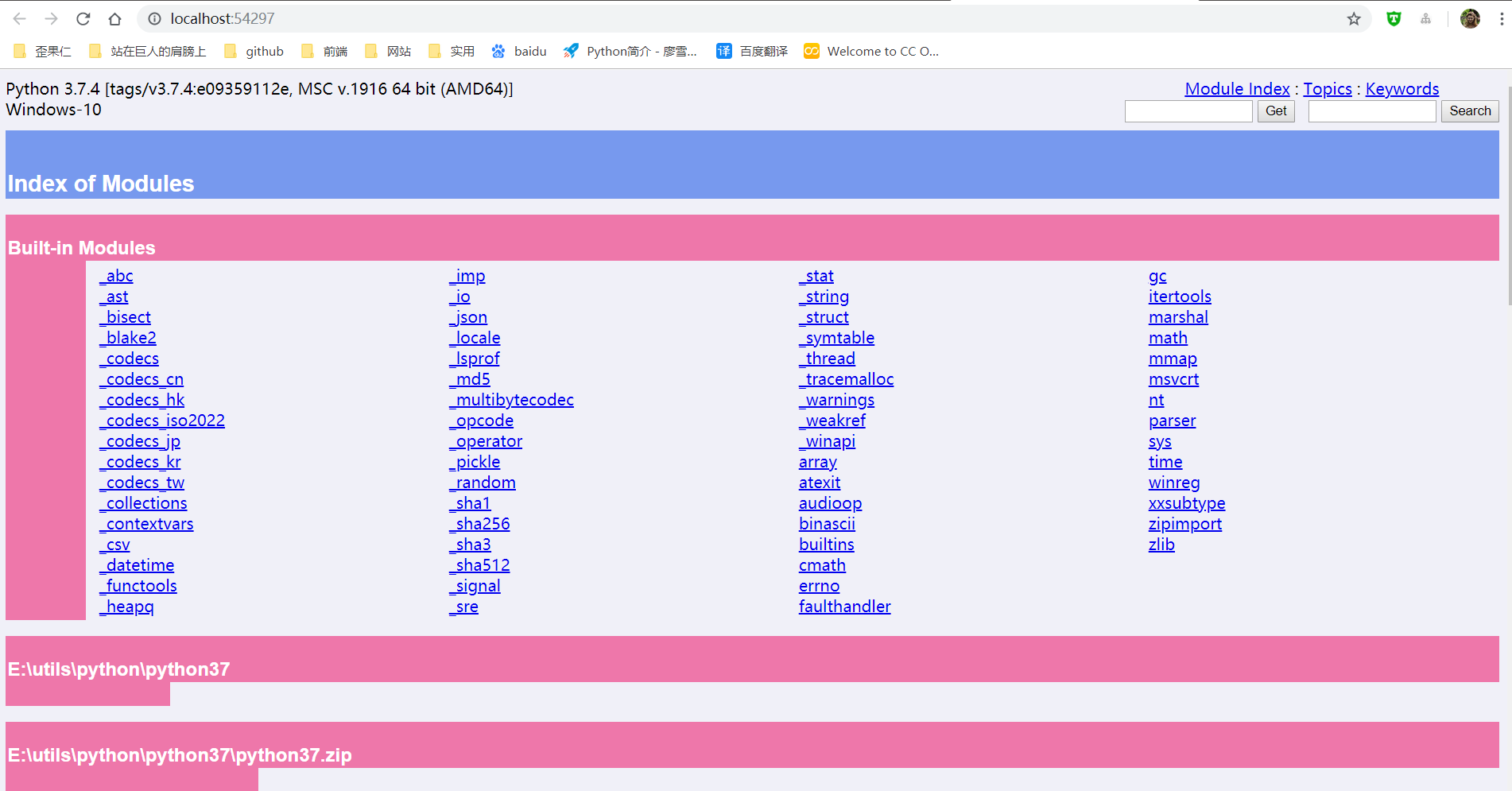IDLE（Python 3.7 64-bit） 一个Python编辑器，Python内置的集成开发工具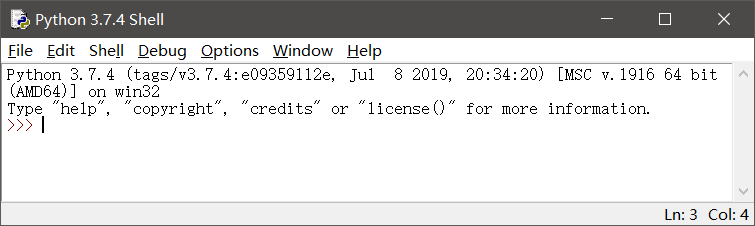Python 3.7 Manuals（64-bit） Python 3.7 开发手册  Python 3.7（64-bit） 控制台中运行Python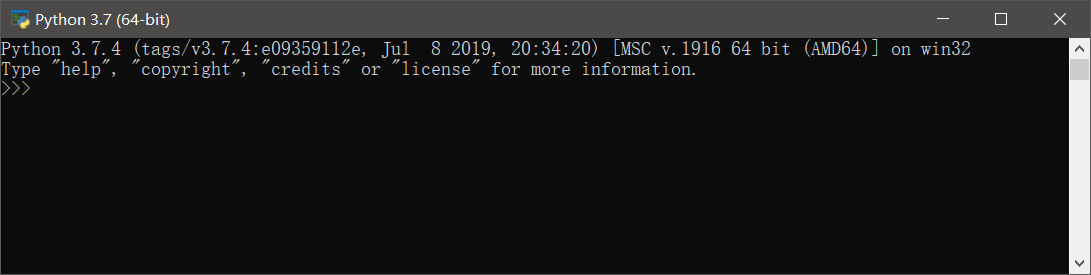二：更新pip和setuptools包，安装NumPy包，安装Matplotlib包
以下三个命令都是在控制台（windows中的cmd）中运行
更新pip和setuptools包
pip用于安装和管理Python扩展包setuptools用于发布Python包
python -m pip install -U pip setuptools

安装NumPy
Python扩展模块NumPy提供了数组和矩阵处理，以及傅立叶变换等高效的数值处理功能
 python -m pip install NumPy

安装Matplotlib包
Matplotlib是Python最著名的绘图库之一，提供了一整套和MATLAB相似的命令API，既适合交互式地进行制图，也可以作为绘图控件方便地嵌入到GUI应用程序中
python -m pip install Matplotlib

三：使用IDLE打开和执行Python源文件程序
首先： 有一个.py文件test.py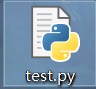使用IDLE打开.py文件的两种方式：
右键test.py---->Edit With IDLE---->Edit With IDLE 3.7（64-bit）打开IDLE，然后File---->Open（或者ctrl+O）选择.py文件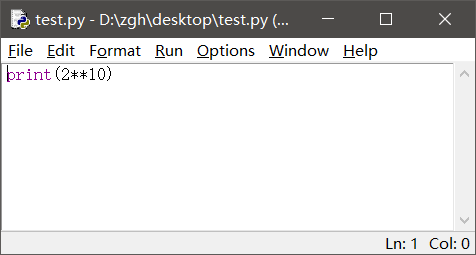运行
Run---->Run Module（或者F5） 就会出现这个界面，执行结果显示在这个界面中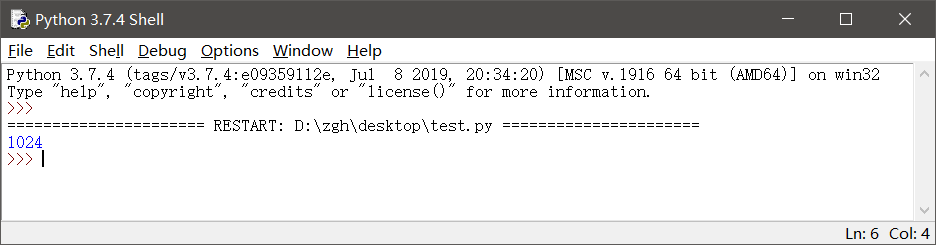补充一点： 如果在IDLE中编辑.py文件，记得修改后要保存（ctrl+s），再运行（F5）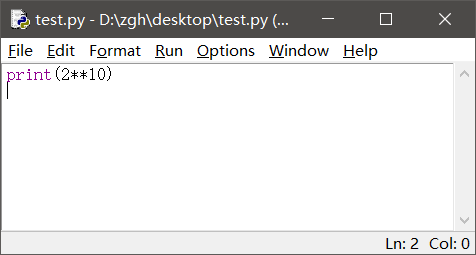四：使用资源管理器运行hello.py
hello.py文件在桌面
import random

print("hello,Python")
print("你今天的随机数字是：",random.choice(range(10)))#输出在0-9之间随机选择的整数
input()

在桌面打开PowerShell（还有两种输入方式：python hello.py或者.\hello.py）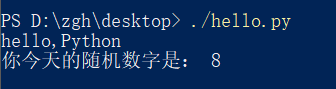或者在桌面打开cmd, 就输入hello.py或者python hello.py
补充：上述两种命令中的hello.py都是相对路径，因为文件在桌面，而且我是在桌面打开cmd，所以文件路劲可以这么简简单单的写。如果文件存储位置和cmd打开位置不一样，请使用绝对路径
五：命令行参数示例hello_argv.py
hello_argv.py文件在桌面
import sys

print("Hello,",sys.argv)
#这样写也行：
#print("Hello,"+sys.argv)

在桌面打开PowerShell（还有两种输入方式：python hello_argv.py 任意输入或者./hello_argv.py 任意输入）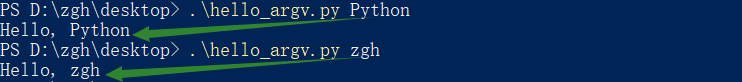或者在桌面打开cmd，就输入hello_argv.py 任意输入或者python hello_argv.py 任意输入
补充：以图中第一个命令举例，hello_argv.py即sys.argv；Python即sys.argv
第二章 Python语言基础

选择题：1、3、7、8
1. 在Python中，以下标识符合法的是
A. _B. 3CC. it’sB. str
答案：A

标识符的第一个字符必须是字母，下划线（_）；其后的字符可以是字母、下划线或数字。一些特殊的名称，作为python语言的保留关键字，不能作为标识符以双下划线开始和结束的名称通常具有特殊的含义。例如__init__为类的构造函数，一般应避免使用

B：以数字开头，错误 C：使用了'，不是字母、下划线或数字 D：str是保留关键字
3. 在下列Python语句中非法的是
A. x = y =1B. x = (y =1)C. x,y = y,xB. x=1;y=1
答案：B，C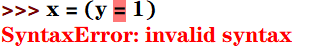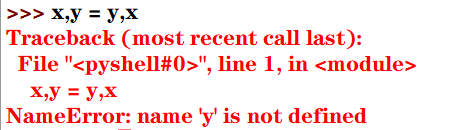7. 为了给整型变量x，y，z赋初值10，下面Python赋值语句正确的是
A. xyz=10B. x=10 y=10 z=10C. x=y=z=10B. x=10，y=10，z=10
答案：C

分号;用于在一行书写多个语句python支持链式赋值

A：赋值对象是xyz B：分号;用于在一行书写多个语句，而不是' '（即空格） D：分号;用于在一行书写多个语句，而不是,
8. 为了给整型变量x，y，z赋初值5，下面Python赋值语句正确的是
A. x=5;y=5;z=5B. xyz=5C. x,y,z=10B. x=10，y=10，z=10
答案：A

Pytho能支持序列解包赋值，但是变量的个数必须与序列的元素个数一致，否则会报错

B：赋值对象是xyz C：序列解包赋值，变量的个数必须与序列的元素个数一致，否则会报错 D：分号;用于在一行书写多个语句，而不是,
思考题：9
9.下列Python语句的输出结果是
def f():pass
print(type(f()))

结果：<class 'NoneType'>

NoneType数据类型包含唯一值None，主要用于表示空值，如没有返回值的函数的结果

上机实践：2~6
2. 编写程序，输入本金、年利率和年数，计算复利（结果保留两位小数）
money = int(input("请输入本金："))
rate = float(input("请输入年利率："))
years = int(input("请输入年数："))
amount = money*((1+rate/100)**years)
print(str.format("本金利率和为：{0:2.2f}",amount))

运行：
请输入本金：1000
请输入年利率：6.6
请输入年数：10
本金利率和为：1894.84

3. 编写程序，输入球的半径，计算球的表面积和体积（结果保留两位小数）
import math
r = float(input("请输入球的半径："))
area = 4 * math.pi * r**2
volume = 4/3*math.pi*r**3
print(str.format("球的表面积为：{0:2.2f}，体积为：{1:2.2f}",area,volume))

运行：
请输入球的半径：666
球的表面积为：5573889.08，体积为：1237403376.70

4. 编写程序，声明函数getValue(b,r,n)，根据本金b，年利率r和年数n计算最终收益v
money = int(input("请输入本金："))
rate = float(input("请输入年利率(<1)："))
years = int(input("请输入年数："))

def getValue(b,r,n):
return b*(1+r)**n

print(str.format("本金利率和为：{0:2.2f}",getValue(money,rate,years)))

运行：
请输入本金：10000
请输入年利率(<1)：0.6
请输入年数：6
本金利率和为：167772.16

5. 编写程序，求解一元二次方程x2-10x+16=0
from math import sqrt
x = (10+sqrt(10*10-4*16))/2
y = (10-sqrt(10*10-4*16))/2
print(str.format("x*x-10*x+16=0的解为：{0:2.2f}，{1:2.2f}",x,y))

运行：
x*x-10*x+16=0的解为：8.00，2.00

6. 编写程序，提示输入姓名和出生年份，输出姓名和年龄
import datetime
sName = str(input("请输入您的姓名："))
birthday = int(input("请输入您的出生年份："))
age = datetime.date.today().year - birthday
print("您好！{0}。您{1}岁。".format(sName,age))

运行：
请输入您的姓名：zgh
请输入您的出生年份：1999
您好！zgh。您20岁。

案例研究：使用Pillow库处理图像文件
https://blog.csdn.net/Zhangguohao666/article/details/102060722
通过此案例，进一步了解Python的基本概念：模块、对象、方法和函数的使用
第三章 程序流程控制

几个例题
一：编程判断某一年是否为闰年

闰年：年份能被4整除但不能被100整除，或者可以被400整除。 口诀：四年一闰，百年不闰，四百必闰

代码一：
y = int(input("请输入要判断的年份："))
if((y % 4 == 0 and y % 100 != 0) or y % 400 == 0):
print("是闰年")
else:
print("不是闰年")

代码二（使用calendar模块的isleap()函数来判断）：
from calendar import isleap

y = int(input("请输入要判断的年份："))
if(isleap(y)):print("闰年")
else:print("不是闰年")

二：利用嵌套循环打印九九乘法表
九九乘法表：
for i in range(1,10):
s = ""
for j in range(1,10):
s += str.format("%d * %d = %02d  " %(i, j, i*j))
print(s)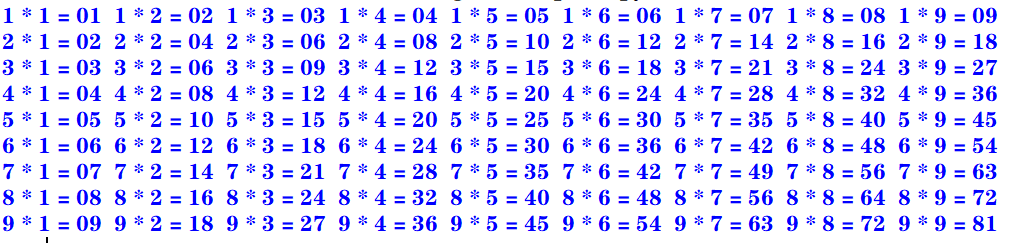下三角：
for i in range(1,10):
s = ""
for j in range(1,i+1):
s += str.format("%d * %d = %02d  " %(i, j, i*j))
print(s)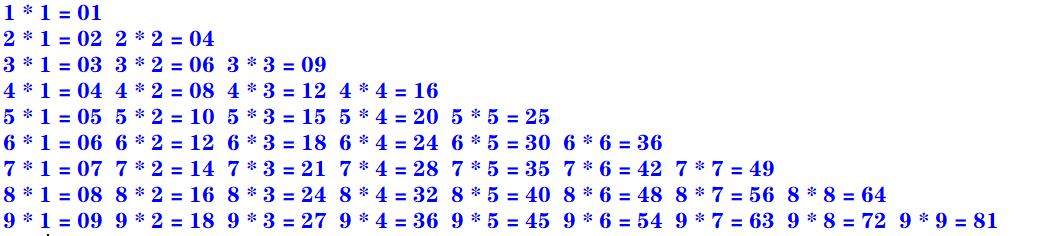上三角：
for i in range(1,10):
s = ""
for k in range(1,i):
s += "                   "
for j in range(i,10):
s += str.format("%d * %d = %02d  " %(i, j, i*j))
print(s)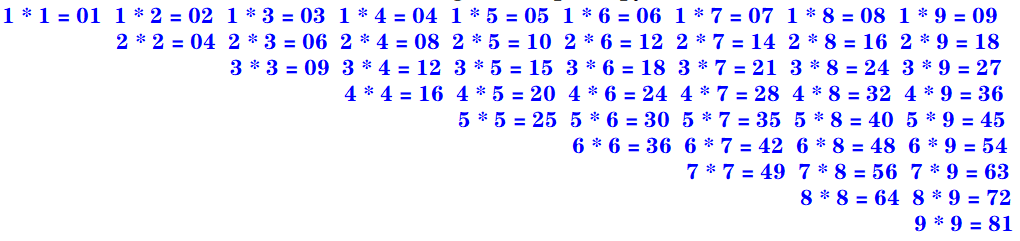三：enumerate()函数和下标元素循环示例

Python语言中的for循环直接迭代对象集合中的元素，如果需要在循环中使用索引下标访问集合元素，则可以使用内置的enumerate()函数

enumerate()函数用于将一个可遍历的数据对象（例如列表、元组或字符串）组合为一个索引序列，并返回一个可迭代对象，故在for循环当中可直接迭代下标和元素
seasons = ["Spring","Summer","Autumn","Winter"]
for i,s in enumerate(seasons,start=1):    #start默认从0开始
print("第{0}个季节：{1}".format(i,s))

运行：
第1个季节：Spring
第2个季节：Summer
第3个季节：Autumn
第4个季节：Winter

四：zip()函数和并行循环示例

如果需要并行遍历多个可迭代对象，则可以使用Python的内置函数zip()

zip()函数将多个可迭代对象中对应的元素打包成一个个元组，然后返回一个可迭代对象。如果元素的个数不一致，则返回列表的长度与最短的对象相同。
利用运算符*还可以实现将元组解压为列表
evens = [0,2,4,6,8]
odds = [1,3,5,7,9]
for e,o in zip(evens,odds):
print("{0} * {1} = {2}".format(e,o,e*o))

运行：
0 * 1 = 0
2 * 3 = 6
4 * 5 = 20
6 * 7 = 42
8 * 9 = 72

五：map()函数和循环示例

如果需要遍历可迭代对象，并使用指定函数处理对应的元素，则可以使用Python的内置函数map()

map(func,seq1[,seq2,...])

func作用于seq中的每一个元素，并将所有的调用结果作为可迭代对象返回。如果func为None，该函数的作用等同于zip()函数
计算绝对值：
>>> list(map(abs, [-1, 0, 7, -8]))
[1, 0, 7, 8]

计算乘幂：
>>> list(map(pow, range(5), range(5)))
[1, 1, 4, 27, 256]

计算ASCII码：
>>> list(map(ord, 'zgh'))
[122, 103, 104]

字符串拼接（使用了匿名函数lambda）：
>>> list(map(lambda x, y: x+y, 'zgh', '666'))
['z6', 'g6', 'h6']

选择题：1、2、3
1. 下面的Python循环体的执行次数与其他不同的是
A.
i = 0
while(i <= 10):
print(i)
i = i + 1

B.
i = 10
while(i > 0):
print(i)
i = i - 1

C.
for i in range(10):
print(i)

D.
for i in range(10,0,-1):
print(i)

答案：A
A：[0,10] 执行11次 B：[10,1] 执行10次 C：[0,9) 执行10次 D：[10,0) 执行10次
2. 执行下列Python语句将产生的结果是
x = 2; y = 2.0
if(x == y): print("Equal")
else: print("Not Equal")

A. EqualB. Not EqualC. 编译错误D. 运行时错误
答案：A

Python中的自动类型转换：
自动类型转换注意针对Number数据类型来说的当2个不同类型的数据进行运算的时候,默认向更高精度转换数据类型精度从低到高:bool int float complex关于bool类型的两个值：True 转化成整型是1；False 转化成整型是0

int类型的2转化为float类型的2.0
3. 执行下列Python语句将产生的结果是
i= 1
if(i): print(True)
else: print(False)

A. 输出1B. 输出TrueC. 输出FalseD. 编译错误
答案：B
在Python中，条件表达式最后被评价为bool值True或False。
如果表达式的结果为数值类型（0），空字符串（""），空元组（()），空列表（[]），空字典（{}），其bool值为False，否则其bool值为True
填空题：6
6. 要使语句for i in range(_,-4,-2)循环执行15次，则循环变量i的初值应当为
答案：26或者25
一开始我给的答案是26，经过评论区 亻的提醒：>>> a = 0
>>> for i in range(26, -4, -2): a+=1

>>> print(a)
15

>>> a = 0
>>> for i in range(25, -4, -2): a+=1

>>> print(a)
15

这种题目有一个规律：for i in range(x,y,z): 若循环中没有break或者continue语句， 执行次数的绝对值：result = (x-y)÷z
但实际上没有这么简单：
如果步长为 -1或者1，那么答案只有一个如果步长为 -2或者2，那么答案有两个如果步长为 -3或者3，那么答案有三个…
通过公式算出 x 之后，
如果步长为2，还要计算 (x ± 1) - z × (result-1) 的值，然后再经过琐碎的判断即可如果步长为3，还要计算 (x ± 2) - z × (result-1) 的值，……
虽然看着麻烦，但实际上是很好理解的
思考题：3~6
3. 阅读下面的Python程序，请问程序的功能是什么？
from math import sqrt

n = 0
for m in range(101,201,2):
k = int(sqrt(m))
for i in range(2, k+2):
if m % i == 0:break
if i == k + 1:
if n % 10 == 0:print()
print('%d' % m,end = " ")
n += 1

输出101到200之间的素数 每行输出10个，多余换行
运行：
101 103 107 109 113 127 131 137 139 149
151 157 163 167 173 179 181 191 193 197
199


素数（质数）是指在大于1的自然数中，除了1和它本身以外不再有其他因数的自然数。

4. 阅读下面的Python程序，请问输出的结果使什么？
n = int(input("请输入图形的行数："))
for i in range(0, n):
for j in range(0, 10 - i):print(" ", end=" ")
for k in range(0, 2 * i + 1):print(" * ", end=" ")
print("\n")

输出的是一个金字塔
运行：
请输入图形的行数：4
*

*   *   *

*   *   *   *   *

*   *   *   *   *   *   *

5. 阅读下面的Python程序，请问输出的结果使什么？程序的功能是什么？
for i in range(100,1000):
n1 = i // 100
n2 = i // 10 % 10
n3 = i % 10
if(pow(n1, 3) + pow(n2, 3) + pow(n3, 3) == i):print(i, end=" ")

输出三位数中所有的水仙花数
运行：
153 370 371 407


水仙花数 是指一个 3 位数，它的每个位上的数字的 3次幂之和等于它本身

6. 阅读下面的Python程序，请问输出的结果使什么？程序的功能是什么？
for n in range(1,1001):
total = 0; factors = []
for i in range(1, n):
if(n % i == 0):
factors.append(i)
total += i
if(total == n):print("{0} : {1}".format(n, factors))

输出1到1000的所有完数，并输出每个完数的所有因子
运行：
6 : [1, 2, 3]
28 : [1, 2, 4, 7, 14]
496 : [1, 2, 4, 8, 16, 31, 62, 124, 248]


完数 所有的真因子（即除了自身以外的约数）的和（即因子函数），恰好等于它本身

上机实践：2~14
2. 编写程序，计算1=2+3+…+100之和
使用for循环（递增）：
total = 0
for i in range(101):
total += i
print(total)

使用求和公式：
>>> (1 + 100) * 100 /2
5050.0

使用累计迭代器itertools.accumulate
>>> import itertools
>>> list(itertools.accumulate(range(1, 101)))
5050

3. 编写程序，计算10+9+8+…+1之和
使用for循环（递增）：
total = 0
for i in range(11):
total += i
print(total)

使用for循环（递减）：
total = 0
for i in range(10,0,-1):
total += i
print(total)

使用求和公式：
>>> (1 + 10) * 10 / 2
55.0

使用累计迭代器itertools.accumulate
>>> import itertools
>>> list(itertools.accumulate(range(1,11)))
55

4. 编写程序，计算1+3+5+7+…+99之和
使用for循环（递增）：
total = 0
for i in range(1,100,2):
total += i
print(total)

使用求和公式：
>>> (1 + 99) * 50 /2
2500.0

使用累计迭代器itertools.accumulate
>>> import itertools
>>> list(itertools.accumulate(range(1,100,2)))
2500

5. 编写程序，计算2+4+6+8+…+100之和
使用for循环（递增）：
total = 0
for i in range(2,101,2):
total += i
print(total)

使用求和公式：
>>> (2 + 100) * 50 / 2
2550.0

使用累计迭代器itertools.accumulate
>>> import itertools
>>> x = list(itertools.accumulate(range(2,101,2)))
>>> x[len(x)-1]
2550

6. 编写程序，使用不同的实现方法输出2000~3000的所有闰年
代码一：
for y in range(2000,3001):
if((y % 4 == 0 and y % 100 != 0) or y % 400 == 0):
print(y,end = ' ')

代码二（使用calendar模块的isleap()函数来判断）：
from calendar import isleap

for y in range(2000,3001):
if(isleap(y)):print(y,end = " ")

运行：
2000 2004 2008 2012 2016 2020 2024 2028 2032 2036 2040 2044 2048 2052 2056 2060 2064 2068 2072 2076 2080 2084 2088 2092 2096 2104 2108 2112 2116 2120 2124 2128 2132 2136 2140 2144 2148 2152 2156 2160 2164 2168 2172 2176 2180 2184 2188 2192 2196 2204 2208 2212 2216 2220 2224 2228 2232 2236 2240 2244 2248 2252 2256 2260 2264 2268 2272 2276 2280 2284 2288 2292 2296 2304 2308 2312 2316 2320 2324 2328 2332 2336 2340 2344 2348 2352 2356 2360 2364 2368 2372 2376 2380 2384 2388 2392 2396 2400 2404 2408 2412 2416 2420 2424 2428 2432 2436 2440 2444 2448 2452 2456 2460 2464 2468 2472 2476 2480 2484 2488 2492 2496 2504 2508 2512 2516 2520 2524 2528 2532 2536 2540 2544 2548 2552 2556 2560 2564 2568 2572 2576 2580 2584 2588 2592 2596 2604 2608 2612 2616 2620 2624 2628 2632 2636 2640 2644 2648 2652 2656 2660 2664 2668 2672 2676 2680 2684 2688 2692 2696 2704 2708 2712 2716 2720 2724 2728 2732 2736 2740 2744 2748 2752 2756 2760 2764 2768 2772 2776 2780 2784 2788 2792 2796 2800 2804 2808 2812 2816 2820 2824 2828 2832 2836 2840 2844 2848 2852 2856 2860 2864 2868 2872 2876 2880 2884 2888 2892 2896 2904 2908 2912 2916 2920 2924 2928 2932 2936 2940 2944 2948 2952 2956 2960 2964 2968 2972 2976 2980 2984 2988 2992 2996

7. 编写程序，计算Sn=1-3+5-7+9-11…
代码一：
n = int(input("项数："))
total = 0
flag = True
for i in range(1,2*n,2):
if(flag):
total += i
flag = False
else:
total -= i
flag = True
print(total)

代码二：
n = int(input("项数："))
total = 0
x = 2
for i in range(1,2*n,2):
total += pow(-1,x)*i
x += 1
print(total)

运行：
项数：10
-10

8. 编写程序，计算Sn=1+1/2+1/3+…
n = int(input("项数："))
total = 0.0
for i in range(1,n+1):
total += 1/i
print(total)

运行：
项数：10
2.9289682539682538

9. 编写程序，打印九九乘法表。要求输入九九乘法表的各种显示效果（上三角，下三角，矩形块等方式）
矩形块：
for i in range(1,10):
s = ""
for j in range(1,10):
s += str.format("%d * %d = %02d  " %(i, j, i*j))
print(s)下三角：
for i in range(1,10):
s = ""
for j in range(1,i+1):
s += str.format("%d * %d = %02d  " %(i, j, i*j))
print(s)上三角：
for i in range(1,10):
s = ""
for k in range(1,i):
s += "                   "
for j in range(i,10):
s += str.format("%d * %d = %02d  " %(i, j, i*j))
print(s)10. 编写程序，输入三角形的三条边，先判断是否可以构成三角形，如果可以，则进一步求三角形的周长和面积，否则报错“无法构成三角形！”
from math import sqrt

a = float(input("请输入三角形的边长a："))
b = float(input("请输入三角形的边长b："))
c = float(input("请输入三角形的边长c："))

if(a < b): a,b = b,a
if(a < c): a,c = c,a
if(b < c): b,c = c,b

if(a < 0 or b < 0 or c < 0 or b+c <= a): print("无法构成三角形！")
else:
h = (a+b+c)/2
area = sqrt(h*(h-a)*(h-b)*(h-c))
print("周长：{0}，面积：{1}".format(a+b+c,area))

运行：
请输入三角形的边长a：4
请输入三角形的边长b：3
请输入三角形的边长c：5
周长：12.0，面积：6.0

11. 编写程序，输入x，根据如下公式计算分段函数y的值。请分别用单分支语句，双分支语句结构以及条件运算语句等方法实现
y = (x2-3x)/(x+1) + 2π + sinx （x≥0 ） y = ln(-5x) + 6√(|x|+e4) - (x+1)3 (x<0)
单分支语句：
import math

x = float(input("请输入x："))
if(x >= 0):
y = (x*x - 3*x)/(x+1) + 2*math.pi + math.sin(x)
if(x < 0):
y = math.log(-5*x) + 6 * math.sqrt(abs(x) + math.exp(4)) - pow(x+1,3)

print(y)


双分支语句：
import math

x = float(input("请输入x："))
if(x >= 0):
y = (x*x - 3*x)/(x+1) + 2*math.pi + math.sin(x)
else:
y = math.log(-5*x) + 6 * math.sqrt(abs(x) + math.exp(4)) - pow(x+1,3)

print(y)

条件运算语句：
import math

x = float(input("请输入x："))
y = ((x*x - 3*x)/(x+1) + 2*math.pi + math.sin(x)) if(x >= 0) \
else (math.log(-5*x) + 6 * math.sqrt(abs(x) + math.exp(4)) - pow(x+1,3))

print(y)

运行一：
请输入x：666
668.2715406628656

运行二：
请输入x：-666
294079794.1744833

12. 编写程序，输入一元二次方程的3个系数a、b、c，求ax2+bx+c=0方程的解
import math

a = float(input("请输入系数a："))
b = float(input("请输入系数b："))
c = float(input("请输入系数c："))

delta = b*b -4*a*c

if(a == 0):
if(b == 0): print("无解")
else: print("有一个实根：",-1*c/b)
elif(delta == 0): print("有两个相等实根：x1 = x2 = ", (-1*b)/(2*a))
elif(delta > 0): print("有两个不等实根：x1 = {0}，x2 = {1}".format\
((-1*b +math.sqrt(delta))/2*a,(-1*b -math.sqrt(delta))/2*a))
elif(delta < 0): print("有两个共轭复根：x1 = {0}，x2 = {1}".format\
(complex( (-1*b)/(2*a),math.sqrt(delta*-1)/(2*a)),complex( (-1*b)/(2*a),-1*math.sqrt(delta*-1)/(2*a))))

运行一：
请输入系数a：0
请输入系数b：0
请输入系数c：10
无解

运行二：
请输入系数a：0
请输入系数b：10
请输入系数c：5
有一个实根： -0.5

运行三：
请输入系数a：1
请输入系数b：8
请输入系数c：16
有两个相等实根：x1 = x2 =  -4.0

运行四：
请输入系数a：1
请输入系数b：-5
请输入系数c：6
有两个不等实根：x1 = 3.0，x2 = 2.0

运行五：
请输入系数a：5
请输入系数b：2
请输入系数c：1
有两个共轭复根：x1 = (-0.2+0.4j)，x2 = (-0.2-0.4j)

13. 编写程序，输入整数n（n≥0）,分别利用for循环和while循环求n!
for循环
n = int(input("请输入n："))

if(n == 0): total = 1
if(n > 0):
total = 1
for i in range(n,0,-1):
total *= i

print(total)


while循环
n = int(input("请输入n："))

if(n == 0): total = 1
if(n > 0):
total = 1
while(n >= 1):
total *= n
n -= 1

print(total)

补充一个：使用累计迭代器itertools.accumulate
>>> import itertools, operator
>>> n = int(input('请输入n：'))
请输入n：7
>>> x = list(accumulate(range(1, n+1), operator.mul))
>>> x[len(x)-1]
5040

14. 编写程序，产生两个0~100（包含0和100）的随机整数a和b，求这两个整数的最大公约数和最小公倍数
现有知识点解决方法

import random

a = random.randint(0,100)
b = random.randint(0,100)
sum = a*b

print(a) #输出原来的a，b
print(b)

if(a < b): a,b = b,a

while(a%b != 0):
a,b = b,a%b

print("最大公约数：{0}，最小公倍数：{1}".format(b,sum/b))


补充：使用生成器（generate）函数：yield
>>> def func(a, b):
if(a < b): a,b = b,a
while(a%b != 0):
a,b = b,a%b
yield b

>>> import random
>>> if __name__ == '__main__':
a = random.randint(0,100)
b = random.randint(0,100)
sum = a*b
print(a,b)
t = list(iter(func(a, b)))
gcd = t[len(t)-1]
print("gcd = {0}, mcm = {1}".format(gcd, sum/gcd))

29 65
gcd = 1, mcm = 1885.0

补充：使用math模块中的gcd(x,y)函数
>>> import random
>>> import math
>>> if __name__ == '__main__':
a = random.randint(0,100)
b = random.randint(0,100)
sum = a*b
print(a,b)
gcd = math.gcd(a,b)
print("gcd = {0}, mcm = {1}".format(gcd, sum/gcd))

29 48
gcd = 1, mcm = 1392.0

案例研究：使用嵌套循环实现图像处理算法
https://blog.csdn.net/Zhangguohao666/article/details/103935185
通过图像处理算法案例，深入了解Python数据结构和基本算法流程
第四章 常用内置数据类型

几个例题
一：Python内置数据类型概述
Python中一切皆为对象，而每个对象属于某个数据类型

Python的数据类型包括：
内置的数据类型模块中定义的数据类型用户自定义的类型

四种内置的数值类型：int，float，bool，complex

int 与其他计算机语言有精度限制不同，Python中的整数位数可以为任意长度（只受限于计算机内存）。 整型对象是不可变对象。float 与其他计算机语言中的double和float对应 Python的浮点类型的精度和系统相关boolcomplex 当数值字符串中包含虚部j（或J）时即复数字面量

序列数据类型：str，tuple，bytes，list，bytearray

序列数据类型表示若干有序数据.

不可变序列数据类型：
str（字符串） 表示Unicode字符序列，例如：“zgh666” 在Python中没有独立的字符数据类型，字符即长度为1的字符串tuple（元组） 表示任意数据类型的序列，例如：(“z”,“g”,“h”,6,6,6)bytes（字节序列） 表示字节（8位）序列数据

可变序列数据类型：
list（列表） 表示可以修改的任意类型数据的序列，比如：[‘z’,‘g’,‘h’,6,6,6]bytearray（字节数组） 表示可以修改的字节（8位）数组

集合数据类型：set，frozenset

集合数据类型表示若干数据的集合，数据项目没有顺序，且不重复
set（集） 例如：{1，2，3}frozenset（不可变集）

字典数据类型：dict

字典数据类型用于表示键值对的字典 例如：{1:"zgh", 2:666}

NoneType，NotImplementedType，EllipsisType

NoneType数据类型包含唯一值None，主要用于表示空值，如没有返回值的函数的结果NotImplementedType数据类型包含唯一值NotImplemented，在进行数值运算和比较运算时，如果对象不支持，则可能返回该值EllipsisType数据类型包含唯一值Ellipsis，表示省略字符串符号...

其他数据类型

Python中一切对象都有一个数据类型，模块、类、对象、函数都属于某种数据类型 Python解释器包含内置类型， 例如： 代码对象Code objects 框架对象Frame objects 跟踪对象Traceback objects 切片对象Slice objects 静态方法对象Static method objects 类方法对象Class method objects

二：整型字面量示例

Python3.7支持使用下划线作为整数或者浮点数的千分位标记，以增强大数值的可阅读性。 二进制、八进制、十六进制则使用下划线区分4位标记

1_000_000_000  #输出1000000000

0xff_ff_ff_ff  #输出4294967295
0x_FF_FF_FF_FF  #输出4294967295

三：字符串字面量示例

两个紧邻的字符串，如果中间只有空格分隔，则自动拼接位一个字符串

'zgh' '666'  #输出'zgh666'
'zgh' + "666"   #输出'zgh666'

四：转义字符示例

转义字符后跟Unicode编码也可以表示字符
\ooo八进制Unicode码对应的字符\xhh十六进制Unicode码对应的字符

'\101'  #输出'A'
'\x41'  #输出'A'


使用r’‘或者R’'的字符串称为原始字符串，其中包含的任何字符都不进行转义

s = r'换\t行\t符\n'
s  		  #输出：'换\\t行\\t符\\n'
print(s)  #输出：换\\t行\\t符\\n

五：字符串的格式化
一：
"student number：{0}，score_average：{1}".format(2,100)
#输出：'student number：2，score_average：100'

二：
str.format("student number：{0}，score_average：{1}",2,100)
#输出：'student number：2，score_average：100'

三（兼容Python2的格式，不推荐使用）：
 "student number：%4d，score_average：%2.1f" %(2,100)
#输出：'student number：   2，score_average：100.0'

六：字符串示例，格式化输出字符串堆积的三角形

str.center()方法用于字符串两边填充str.rjust()方法用于字符串右填充

print("1".center(20))		#一行20个字符，居中对齐
print(format("121","^20"))	#一行20个字符，居中对齐
print("1".rjust(20,"*"))	#一行20个字符，右对齐，加*
print(format("121","*>20"))	#一行20个字符，右对齐，加*

运行：
         1
121
*******************1
*****************121

选择题：11
11. 关于Python字符串，下列说法错误的是
A. 字符即长度为1的字符串 B. 字符串以/0标识字符串的结束 C. 用户既可以用单引号，也可以用双引号创建字符串 D. 用三引号字符串中可以包含换行回车等特殊字符
答案：B

Python中字符串不是用\0来判断字符串结尾， 每个字符串都存有字符串的长度，通过计数来判断是否到达结尾。

虽然在c语言中\0就是来判断字符串的结尾;
填空题：4、7、8、9、10、13、21
4. Python表达式3 ** 2 ** 3的值为
答案：6561

表达式中，相同优先级的运算，从右往左

7. Python语句print(pow(-3,2),round(18.67,1),round(18.67,-1))的输出结果是
答案：9 18.7 20.0

pow()幂运算 round()四舍六入，五留双

8. Python语句print(round(123.84,0),round(123.84,-2),floor(15.5))的输出结果是
答案：124.0 100.0 15

补充：floor()是math模块中的方法，向下取整

9. Python语句print(int(‘20’,16),int(‘101’,2))的输出结果是
答案：32 5

注意：int(x,y)是指将y进制的数值x转化为10进制数

10. Python语句print(hex(16),bin(10))的输出结果是
答案：0x10 0b1010

hex(x)将十进制数x转化为十六进制，以字符串形式输出 bin(x)将十进制数x转化为二进制，以字符串形式输出

13. Python语句print(gcd(12,16),divmod(7,3))的输出结果是
答案：4 (2,1)

gcd()是math模块中的函数，求最大公约数 divmod()是内置函数，返回商和余数

21. Python语句序列 x=True;y=False;z=False;print(x or y and z) 的运行结果是
答案：True

and优先级比or高

思考题：5
5. 阅读下面的Python程序，请问输出结果是什么？
from decimal import *

ctx = getcontext()
ctx.prec = 2
print(Decimal('1.78'))#1.78
print(Decimal('1.78') + 0)#1.8
ctx.rounding = ROUND_UP
print(Decimal('1.65') + 0)#1.7
print(Decimal('1.62') + 0)#1.7
print(Decimal('-1.45') + 0)#-1.5
print(Decimal('-1.42') + 0)#-1.5
ctx.rounding = ROUND_HALF_UP
print(Decimal('1.65') + 0)#1.7
print(Decimal('1.62') + 0)#1.6
print(Decimal('-1.45') + 0)#-1.5
ctx.rounding = ROUND_HALF_DOWN
print(Decimal('1.65') + 0)#1.6
print(Decimal('-1.45') + 0)#-1.4

上机实践：2~14
2. 编写程序，格式化输出杨辉三角

杨辉三角即二项式定理的系数表，各元素满足如下条件：第一列及对角线上的元素均为1；其余每个元素等于它上一行同一列元素与前一列元素之和

我使用了一个更加精妙的规律 比如第一行为1 第二行：01 + 10 = 11 第三行：011 + 110 = 121 第四行：0121 + 1210 = 1331 。。。
def generate(numRows):
l1 = 
n = 0
while n < numRows:
print(str(l1).center(66))
l1 = [sum(t) for t in zip( + l1, l1 + )]  #利用zip函数算出每一行 如第二行 zip([0,1],[1,0])=[1,1],以此类推
n += 1
a=int(input("请输入行数"))
generate(a)

运行：
请输入行数4

[1, 1]
[1, 2, 1]
[1, 3, 3, 1]

3. 输入直角三角形的两个直角边，求三角形的周长和面积，以及两个锐角的度数。结果保留一位小数
import math

a = float(input("请输入直角三角形的直角边a："))
b = float(input("请输入直角三角形的直角边b："))
c = math.sqrt(a*a+b*b)

p = a + b + c
area = 0.5*a*b
print("三角形的周长：{0:1.1f}，面积：{1:1.1f}".format(p,area))

sina = a/c
sinb = b/c

a_degree = round(math.asin(sina) * 180 / math.pi,0)
b_degree = round(math.asin(sinb) * 180 / math.pi,0)

print("三角形直角边a的度数：{0}，b的度数：{1}".format(a_degree,b_degree))

运行：
请输入直角三角形的直角边a：3
请输入直角三角形的直角边b：4
三角形的周长：12.0，面积：6.0
三角形直角边a的度数：37.0，b的度数：53.0

4. 编程产生0~100（包含0和100）的三个随机数a、b、c，要求至少使用两种不同的方法，将三个数按从小到大的顺序排序
方法一：
import random

a = random.randint(0, 100)
b = random.randint(0, 100)
c = random.randint(0, 100)

print(str.format("原始值：{0}，{1}，{2}", a, b, c))

if(a > b): a,b = b,a
if(a > c): a,c = c,a
if(b > c): b,c = c,b

print(str.format("增序：{0}，{1}，{2}", a, b, c))

方法二（使用内置函数max、min、sum）：
import random

a = random.randint(0, 100)
b = random.randint(0, 100)
c = random.randint(0, 100)

print(str.format("原始值：{0}，{1}，{2}", a, b, c))

maxx = max(a, b, c)
minx = min(a, b, c)
median = sum([a, b, c]) - minx - maxx

print(str.format("增序：{0}，{1}，{2}", minx, median, maxx))

方法三（使用内置函数sorted）：
>>> import random
>>> a = random.randint(0,100)
>>> b = random.randint(0,100)
>>> c = random.randint(0,100)
>>> print("init value: {0} , {1} , {2}".format(a,b,c))
init value: 17 , 6 , 59
>>> sorted([a,b,c])
[6, 17, 59]

5. 编程计算有固定工资收入的党员每月所缴纳的党费。
工资基数3000元及以下者，交纳工资基数的0.5% 工资基数3000~5000元者，交纳工资基数的1% 工资基数在5000~10000元者，交纳工资基数的1.5% 工资基数超过10000元者，交纳工资基数的2%
salary = float(input("请输入有固定工资收入的党员的月工资："))
if salary <= 3000: dues = salary*0.005
elif salary <= 5000: dues = salary*0.01
elif salary <= 10000: dues = salary*0.15
else: dues = salary*0.02

print("交纳党费：",dues)

运行：
请输入有固定工资收入的党员的月工资：10001
交纳党费： 200.02

6. 编程实现袖珍计算器，要求输入两个操作数和一个操作符（+、-、*、/、%），根据操作符输出运算结果。注意/和%运算符的零异常问题
a = float(input("请输入操作数（左）："))
b = float(input("请输入操作数（右）："))
operator = input("请输入操作符（+、-、*、/、%）：")

if(b == 0 and (operator == '/' or operator == '%')):
print("分母为零，异常！")
else:
if operator == '+': result = a+b
elif operator == '-': result = a-b
elif operator == '*': result = a*b
elif operator == '/': result = a/b
elif operator == '%': result = a%b
print("{0} {1} {2}= {3}：".format(a,operator,b,result))

运行：
请输入操作数（左）：10
请输入操作数（右）：5
请输入操作符（+、-、*、/、%）：+
10.0 + 5.0= 15.0：

7. 输入三角形的3条边a、b、c，判断此3边是否可以构成三角形。若能，进一步判断三角形的性质，即为等边、等腰、直角或其他三角形
a = float(input("请输入三角形的边a："))
b = float(input("请输入三角形的边b："))
c = float(input("请输入三角形的边c："))

if(a > b): a,b = b,a
if(a > c): a,c = c,a
if(b > c): b,c = c,b

result = "三角形"
if(not(a>0 and b>0 and c>0 and a+b>c)):
result = '此三边无法构成三角形'
else:
if a == b == c: result = '等边三角形'
elif(a==b or a==c or b==c): result = '等腰三角形'
elif(a*a+b*b == c*c): result = '直角三角形'

print(result)

运行：
请输入三角形的边a：3
请输入三角形的边b：4
请输入三角形的边c：5
直角三角形

8. 编程实现鸡兔同笼问题
已知在同一个笼子里共有h只鸡和兔，鸡和兔的总脚数为f，其中h和f由用户输入，求鸡和兔各有多少只？要求使用两种方法：一是求解方程；二是利用循环进行枚举测试
h = int(input("请输入总头数："))
f = int(input("请输入总脚数："))

def fun1(h,f):
rabbits = f/2-h
chicken = h-rabbits
if(chicken < 0 or rabbits < 0): return '无解'
return chicken,rabbits

def fun2(h,f):
for i in range(0,h+1):
if(2*i + 4*(h-i) == f):return i,h-i
return '无解'

if(h>0 and f>0 and f % 2 == 0):
if fun1(h,f)=='无解':
print("无解")
else:
print("方法一：鸡：{0}，兔：{1}".format(fun1(h,f),fun1(h,f)))
print("方法二：鸡：{0}，兔：{1}".format(fun2(h,f),fun2(h,f)))
else:
print('输入的数据无意义')

运行：
请输入总头数：100
请输入总脚数：100
无解

9. 输入任意实数x，计算ex的近似值，直到最后一项的绝对值小于10-6为止
ex = 1 + x + x2/2 + x3/3! + x4/4! + … + xn/n!
x = int(input("请输入任意实数："))

e = 1
i = 1
t = 1
a = 1
while(a >= 10e-6):
t *= i
a = pow(x,i)/t
e += a
i += 1

print(e)

运行：
请输入任意实数：1
2.7182815255731922

我发现了在Python中10e-6和pow(10,-6)是有差别的，将上述代码中的10e-6改为pow(10,-6)，输出结果会有细微的差别
运行：
请输入任意实数：1
2.7182818011463845

10. 输入任意实数a（a>=0），用迭代法求x=√a，要求计算的相对偏差小于10-6
求平方根的公式：
Xn+1 = 0.5(Xn + a/Xn)
import math

a = int(input("请输入任意实数a(>=0)："))

x = a / 2
y = (x + a/x) / 2

while(abs(y-x) >= pow(10,-6)):
x = y
y = (x + a/x) / 2

print(y)

运行：
请输入任意实数a(>=0)：2
1.414213562373095

11. 即有一个数，用3除余2，用5除余3，用7除余2，请问0~1000中这样的数有哪些？
我国古代有位大将，名叫韩信。他每次集合部队，只要求部下先后按1-3，1-5，1-7报数，然后再报告一下各队每次报数的余数，他就知道到了多少人。他的这种巧妙算法被人们称作“鬼谷算”，也叫“隔墙算”，或称为“韩信点兵”，外国人还称它为“中国余数定理”。
for i in range(0,1001):
if((i % 3 == 2 )and (i % 5 == 3) and (i % 7 == 2)): print(i, end="  ")

运行：
23  128  233  338  443  548  653  758  863  968

12. 一球从100米的高度自由下落，每次落地后反弹回原高度的一半，再落下。求小球在第10次落地时共经过多少米?第10次反弹多高
规律： 第一次下落时的高度：100 第二次下落时的高度（第一次反弹的高度）：50 第三次下落时的高度（第二次反弹的高度）：25 …
n = 10

h_down = 100
h_up = 0
sum = 0
for i in range(1,n+1):
sum += h_down+h_up
h_down = h_up = h_down/2

print("小球在第十次落地时共经过：{0}米，第十次反弹高度：{1}米".format(sum,h_up))

运行：
小球在第十次落地时共经过：299.609375米，第十次反弹高度：0.09765625米

13. 猴子吃桃问题
猴子第一天摘下若干个桃子，当天吃掉一半多一个；第二天接着吃了剩下的桃子的一半多一个；以后每天都吃了前一天剩下的桃子的一半多一个。到第八天发现只剩一个桃子了。请问猴子第一天共摘了多少个桃子？
这是一个递推问题
某天所剩桃子数x 后一天所剩桃子数y = x - (x/2+1) = x/2-1
则x = 2(y+1)
result = 1
for i in range(8,0,-1):
print("第{0}天桃子数：{1}".format(i,result))
result = 2*(result+1)

运行：
第8天桃子数：1
第7天桃子数：4
第6天桃子数：10
第5天桃子数：22
第4天桃子数：46
第3天桃子数：94
第2天桃子数：190
第1天桃子数：382

14. 计算Sn = 1+11+111+…+111…111（最后一项是n个1）。n是一个随机产生的1~10（包括1和10）中的正整数
import random

n = random.randint(1,10)

x = 1
s = 0
for i in range(1,n+1):
s += x
x = 10*x+1

print("n = {0}，sn = {1}".format(n,s))

运行：
n = 6，sn = 123456

random.randint(a, b)
生成指定范围内的整数范围：[a, b]
案例研究：科学计算和数据分析
https://blog.csdn.net/Zhangguohao666/article/details/103941448
通过Python科学计算和数据分析库的安装和基本使用，了解使用Python进行科学计算的基本方法
第五章 序列数据类型

几个例题
一：Python中内置的序列数据类型

元组也称为定值表，用于存储固定不变的表列表也称为表，用于存储其值可变的表字符串是包括若干字符的序列数据，支持序列数据的基本操作字节序列数据是包括若干字节的序列。Python抓取网页时返回的页面通常为utf-8编码的字节序列。

字节序列和字符串可以直接相互转换（字节编码和解码）：
>>> s1 = b'abc'
>>> s1
b'abc'
>>> s1.decode("utf-8")
abc

>>> s2 = "中国"
>>> s2.encode("utf-8")

二：序列的切片操作示例
>>> s = 'zgh666'
>>> s
'z'
>>> s
'h'
>>> s[:3]
'zgh'
>>> s[1:3]
'gh'
>>> s[3:6]
'666'
>>> s[3:55]
'666'
>>> s[::-1]
'666hgz'
>>> s[3:2]
''
>>> s[:]
'zgh666'
>>> s[::2]
'zh6'

三：序列的连接和重复操作

通过连接操作符+可以连接两个序列，形成一个新的序列对象通过重复操作符*可以重复一个序列n次连接操作符和重复操作符也支持复合赋值运算，即：+=，*=

>>> x = 'zgh'
>>> y = '666'
>>> x + y
'zgh666'
>>> x *2
'zghzgh'
>>> x += y
>>> x
'zgh666'
>>> y *= 3
>>> y
'666666666'

四：序列的成员关系操作
innot ins.count(x) x在s中出现的次数s.index(x) x在s中第一次出现的下标
>>> s = "zgh666"
>>> 'z' in s
True
>>> 'g' not in s
False
>>> s.count('6')
3
>>> s.index('6')
3

五：序列的排序操作
sorted(iterable,key=None,reverse=False)
>>> sorted(s)
[1, 3, 5, 9]
>>> sorted(s,reverse=True)
[9, 5, 3, 1]

>>> s = 'zGhZgH'
>>> sorted(s)
['G', 'H', 'Z', 'g', 'h', 'z']
>>> sorted(s,key=str.lower)
['G', 'g', 'h', 'H', 'z', 'Z']
>>> sorted(s,key=str.lower,reverse=True)
['z', 'Z', 'h', 'H', 'G', 'g']

六：序列的拆分
变量个数与序列长度相等 若变量个数与序列的元素个数不一致，将导致ValueError
>>> data = (118,'zgh',(100,100,100))
>>> sid,name,(chinese,english,math) = data
>>> sid
118
>>> name
'zgh'
>>> chinese
100
>>> english
100
>>> math
100

变量个数与序列长度不等 如果序列长度未知，可以使用*元组变量，将多个值作为元组赋值给元组变量。在一个赋值语句中，*元组变量只允许出现一次，否则将导致SyntaxError
>>> first,second,third,*middles,last = range(10)
>>> first
0
>>> second
1
>>> third
2
>>> middles
[3, 4, 5, 6, 7, 8]
>>> last
9

>>> first,*middles,last = sorted([58,60,60,100,70,70])
>>> sum(middles)/len(middles)
65.0

使用临时变量_ 如果只需要部分数据，序列的其它位置可以使用临时变量_
>>> record = ['zgh','858990471@qq.com','17354364147','15272502101']
>>> name,_,*phone = record
>>> name
'zgh'
>>> phone
['17354364147', '15272502101']

七：使用元组字面量，tuple创建元组实例对象的实例
>>> t1 = 1,2,3
>>> t1
(1, 2, 3)

>>> t2 = (4,5,6)
>>> t2
(4, 5, 6)

>>> t3 = (9,)
>>> t3
(9,)

如果元组中只有一个项目，后面的逗号不能省略。

Python解释器把(1)解释为整数1，将(1,)解释为元组

>>> t1 = tuple()
>>> t1
()

>>> t2 = tuple("zgh666")
>>> t2
('z', 'g', 'h', '6', '6', '6')

>>> t3 = tuple(['z','g','h'])
>>> t3
('z', 'g', 'h')

八：使用列表字面量，list创建列表实例对象的实例
>>> l1 = []
>>> l1
[]

>>> l2 = ['zgh666']
>>> l2
['zgh666']

>>> l3 = [(1,2,3)]
>>> l3
[(1, 2, 3)]

>>> l1 = list()
>>> l1
[]

>>> l2 = list(b'zgh666')
>>> l2
[122, 103, 104, 54, 54, 54]

>>> l3 = list(b'aAbBcC')
>>> l3
[97, 65, 98, 66, 99, 67]

补充：列表是可变对象，故用户可以改变列表对象中元素的值，也可以通过del删除某元素
九：列表解析表达式示例
使用列表解析表达式可以简单，高效地处理一个可迭代对象，并生成结果列表
>>> [(i,i**2) for i in range(10)]
[(0, 0), (1, 1), (2, 4), (3, 9), (4, 16), (5, 25), (6, 36), (7, 49), (8, 64), (9, 81)]

>>> [i for i in range(10) if i%2==0]
[0, 2, 4, 6, 8]

>>> [(x,y,x*y) for x in range(1,4) for y in range(1,4) if x>=y]
[(1, 1, 1), (2, 1, 2), (2, 2, 4), (3, 1, 3), (3, 2, 6), (3, 3, 9)]

选择题：4、5、7、11、12
4. Python语句序列“a = (1,2,3,None,(),[]);print(len(a))”的运行结果是
>>> a = (1,2,3,None,(),[])
>>> len(a)
6

5. Python语句序列“nums = set([1,2,2,3,3,3,4]);print(len(nums))”的运行结果是
>>> nums = set([1,2,2,3,3,3,4])
>>> nums
{1, 2, 3, 4}
>>> len(nums)
4

7. Python语句序列“s1=[4,5,6];s2=s1;s1=0;print(s2)”的运行结果是
Python中变量（如s1，s2）存储在栈中，存放的是地址 [4,5,6]存储在堆中
s1 = [4,5,6]即s1存储指向堆中[4,5,6]的地址 s2 = s1地址赋值，即s2和s1都指向同一个地址 所以对列表进行修改，两者的显示都会发生变化
>>> s1 = [4,5,6]
>>> s2 = s1
>>> s1 = 0
>>> s1
[4, 0, 6]
>>> s2
[4, 0, 6]

11. Python语句序列“s={‘a’,1,‘b’,2};print(s[‘b’])”的运行结果是
A. 语法错B. ‘b’C. 1D. 2
答案：A
通过值访问集合是没有意义的，语法也不支持
>>> s ={'a',1,'b',2}
>>> print(s['b'])
Traceback (most recent call last):
File "<pyshell#29>", line 1, in <module>
print(s['b'])
TypeError: 'set' object is not subscriptable

补充：集合set是无序不重复的，是无法通过下标访问的
12. Python语句print(r"\nGood")的运行结果是
A. 新行和字符串GoodB. r"\nGood"C. \nGoodD. 字符r、新行和字符串Good
答案：C
>>> print(r"\nGood")
\nGood


r""声明原始字符串

填空题：1、5、6、12、13、14
1. Python语句序列“fruits = [‘apple’,‘banana’,‘bear’];print(fruits[-1][-1])”的运行结果是
注意：fruit[-1]是字符串’bear’ 所以：fruit[-1][-1]即'bear[-1]'
>>> fruits = ['apple','banana','pear']
>>> fruits[-1]
'pear'
>>> fruits[-1][-1]
'r'

5. Python语句 print(’%d%%%d’%(3/2,3%2)) 的运行结果是
>>> print('%d%%%d'%(3/2,3%2))
1%1

6. Python语句序列“s = [1,2,3,4];s.append([5,6]);print(len(s))”的运行结果是
答案：5
注意append()和extend()函数的区别 s.append(x)将对象x追加到s尾部 s.extend(x)将序列x追加到s尾部
append
>>> s = [1,2,3,4]
>>> s.append([5,6])
>>> s
[1, 2, 3, 4, [5, 6]]
>>> len(s)
5

extend
>>> s = [1,2,3,4]
>>> s.extend([5,6])
>>> s
[1, 2, 3, 4, 5, 6]
>>> len(s)
6

12
>>> s =('a','b','c','d','e')
>>> s
'c'
>>> s[2:3]
('c',)
>>> s[2:4]
('c', 'd')
>>> s[1::2]
('b', 'd')
>>> s[-2]
'd'
>>> s[::-1]
('e', 'd', 'c', 'b', 'a')
>>> s[-2:-1]
('d',)
>>> s[-99:-5]
()
>>> s[-99:-3]
('a', 'b')
>>> s[::]
('a', 'b', 'c', 'd', 'e')
>>> s[1:-1]
('b', 'c', 'd')

13
>>> s = [1,2,3,4,5,6]
>>> s[:1] = []
>>> s
[2, 3, 4, 5, 6]

>>> s[:2] = 'a'
>>> s
['a', 4, 5, 6]

>>> s[2:] = 'b'
>>> s
['a', 4, 'b']

>>> s[2:3] = ['x','y']
>>> s
['a', 4, 'x', 'y']

>>> del s[:1]
>>> s
[4, 'x', 'y']

14
>>> s = ['a','b']
>>> s.append([1,2])
>>> s
['a', 'b', [1, 2]]
>>> s.extend('34')
>>> s
['a', 'b', [1, 2], '3', '4']
>>> s.extend([5,6])
>>> s
['a', 'b', [1, 2], '3', '4', 5, 6]
>>> s.insert(1,7)
>>> s
['a', 7, 'b', [1, 2], '3', '4', 5, 6]
>>> s.insert(10,8)
>>> s
['a', 7, 'b', [1, 2], '3', '4', 5, 6, 8]
>>> s
['a', 7, 'b', [1, 2], '3', '4', 5, 6]
>>> s.remove('b')
>>> s
['a', 7, [1, 2], '3', '4', 5, 6]
>>> s[3:] =[]
>>> s
['a', 7, [1, 2]]
>>> s.reverse()
>>> s
[[1, 2], 7, 'a']
>>>

思考题：2、3、5
2. 阅读下面的Python语句，请问输出结果是什么？
n = int(input('请输入图形的行数：'))

for i in range(n,0,-1):
print(" ".rjust(20-i),end=' ')
for j in range(2*i-1):print(" * ",end=' ')
print('\n')

for i in range(1,n):
print(" ".rjust(19-i),end=' ')
for j in range(2*i+1):print(" * ",end=' ')
print('\n')

运行一：
请输入图形的行数：1
*

运行二：
请输入图形的行数：2
*   *   *

*

*   *   *

运行三：
请输入图形的行数：3
*   *   *   *   *

*   *   *

*

*   *   *

*   *   *   *   *

3. 阅读下面的Python语句，请问输出结果是什么？
n = int(input('请输入上（或下）三角行数：'))

for i in range(0,n):
print(" ".rjust(19-i),end=' ')
for j in range(2*i+1):print(" * ",end=' ')
print('\n')

for i in range(n-1,0,-1):
print(" ".rjust(20-i),end=' ')
for j in range(2*i-1):print(" * ",end=' ')
print('\n')

运行：
请输入上（或下）三角行数：4
*

*   *   *

*   *   *   *   *

*   *   *   *   *   *   *

*   *   *   *   *

*   *   *

*

5. 阅读下面的Python语句，请问输出结果是什么？
先看这三句：
>>> names1 = ['Amy','Bob','Charlie','Daling']
>>> names2 = names1
>>> names3 = names1[:]

毫无疑问，此时names1,names2,names3的值都是[‘Amy’,‘Bob’,‘Charlie’,‘Daling’] 但是
>>> id(names1)
2338529391368
>>> id(names2)
2338529391368
>>> id(names3)
2338529391560

names1和names2指向同一个地址 而names3指向另一个地址
然后：
>>> names2 = 'Alice'
>>> names3 = 'Ben'
>>> names1
['Alice', 'Bob', 'Charlie', 'Daling']
>>> names2
['Alice', 'Bob', 'Charlie', 'Daling']
>>> names3
['Amy', 'Ben', 'Charlie', 'Daling']

最后：
>>> sum = 0
>>> for ls in(names1,names2,names3):
if ls == 'Alice': sum+=1
if ls == 'Ben':sum+=2

>>> print(sum)
4

上机实践：2~6
2. 统计所输入字符串中单词的个数，单词之间用空格分隔
s = input("请输入字符串：")

num = 0
for i in s:
if((i >= 'a' and i <= 'z') or (i >= 'A' and i <= 'Z')):
num += 1

print("其中的单词总数：",num)

运行：
请输入字符串：zgh666 ZGH6
其中的单词总数： 6

3. 编写程序，删除一个list里面重复元素
方法一：利用set集合不重复的性质（但结果不能保证原来的顺序）
l = [1,2,2,3,3,3,4,5,6,6,6]
s = set(l)
l = list(s)
print(l)

运行：
[1, 2, 3, 4, 5, 6]

方法二：既可以去除重复项，又可以保证原来的顺序
def unique(items):
items_existed = set()
for item in items:
if item not in items_existed:
yield item

if __name__ == '__main__':
a = [1, 8, 5, 1, 9, 2, 1, 10]
a1 = unique(a)
print(list(a1))


运行结果：
[1, 8, 5, 9, 2, 10]

对代码的分析：
可以看出，unique()函数返回的并不是items_existed，而是利用了yield

在函数定义中，如果使用yield语句代替return返回一个值，则定义了一个生成器函数（generator） 生成器函数是一个迭代器，是可迭代对象，支持迭代

a1 = unique(a) 这个函数返回的实际上是一个可迭代对象 若print(a1)得到的会是：<generator object unique at 0x0000016E23AF4F48>所以，要得到去掉重复后的列表的样子，需要将可迭代对象a1放在list()中 运行：
4. 编写程序，求列表[9,7,8,3,2,1,55,6]中的元素个数、最大值、最小值，以及元素之和、平均值。请思考有几种实现方法？
内置函数：
s = [9,7,8,3,2,1,55,6]

print("元素个数：{0}，最大值：{1}，最小值：{2}，和：{3}，平均值：{4}".\
format(len(s),max(s),min(s),sum(s),sum(s)/len(s)))

直接访问元素列表（for i in s…）：
s = [9,7,8,3,2,1,55,6]

sum = 0
max = s
min = s
length = 0
for i in s:
sum += i
length += 1
if(i > max): max = i
if(i < min): min = i

print("元素个数：{0}，最大值：{1}，最小值：{2}，和：{3}，平均值：{4}".\
format(length,max,min,sum,sum/length))


间接访问列表元素（for i in range(0,len(s))…）：
s = [9,7,8,3,2,1,55,6]

sum = 0
max = s
min = s
length = len(s)
for i in range(0,length):
sum += s[i]
if(s[i] > max): max = s[i]
if(s[i] < min): min = s[i]

print("元素个数：{0}，最大值：{1}，最小值：{2}，和：{3}，平均值：{4}".\
format(length,max,min,sum,sum/length))


正序访问（i=0;while i<len(s)…）：
s = [9,7,8,3,2,1,55,6]

sum = 0
max = s
min = s
length = len(s)

i = 0
while(i < length):
sum += s[i]
if(s[i] > max): max = s[i]
if(s[i] < min): min = s[i]
i += 1

print("元素个数：{0}，最大值：{1}，最小值：{2}，和：{3}，平均值：{4}".\
format(length,max,min,sum,sum/length))


反序访问（i=len(s)-1;while i>=0…）：
s = [9,7,8,3,2,1,55,6]

sum = 0
max = s
min = s
length = len(s)

i = length-1
while(i >= 0):
sum += s[i]
if(s[i] > max): max = s[i]
if(s[i] < min): min = s[i]
i -= 1

print("元素个数：{0}，最大值：{1}，最小值：{2}，和：{3}，平均值：{4}".\
format(length,max,min,sum,sum/length))


while True：…break
s = [9,7,8,3,2,1,55,6]

sum = 0
max = s
min = s
length = len(s)

i = 0
while(True):
if(i > length-1): break
sum += s[i]
if(s[i] > max): max = s[i]
if(s[i] < min): min = s[i]
i += 1

print("元素个数：{0}，最大值：{1}，最小值：{2}，和：{3}，平均值：{4}".\
format(length,max,min,sum,sum/length))

运行：
元素个数：8，最大值：55，最小值：1，和：91，平均值：11.375

5. 编写程序，将列表[9,7,8,3,2,1,5,6]中的偶数变成它的平方，奇数保持不变
l = [9,7,8,3,2,1,5,6]

for i,value in enumerate(l):
if(value % 2 == 0):l[i] = value**2

print(l)

运行：
[9, 7, 64, 3, 4, 1, 5, 36]

6. 编写程序，输入字符串，将其每个字符的ASCII码形成列表并输出
s = input("请输入一个字符串：")
l = list()
for i in s:
l.append(ord(i))

print(l)

运行：
请输入一个字符串：zgh666
[122, 103, 104, 54, 54, 54]

案例研究：猜单词游戏
https://blog.csdn.net/Zhangguohao666/article/details/103948234
通过猜单词游戏的设计和实现，帮助读者了解使用Python系列数据类型和控制流程
第六章 输入和输出

几个例题
一：运行时提示输入密码

输入密码时，一般需要不明显，则可以使用模块getpass，以保证用户输入的密码在控制台中不回显

import getpass

else:
print('failed!')

input()#为了看到输出结果。因为执行完毕后，控制台会立马关闭


注意：上面这个代码，如果使用IDLE执行，会因为安全问题而执行失败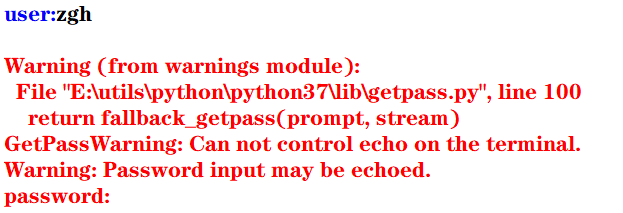但是，在控制台中执行就没问题，看输出结果（可以看到，输入的密码不会显示出来）：
user:zgh

二：重定向标准输出到一个文件的示例
这种重定向由控制台完成，而与Python本身无关。

格式： 程序 > 输出文件

其目的是将显示屏从标准输出中分离，并将输出文件与标准输出关联，即程序的执行结果将写入输出文件，而不是发送到显示屏中显示

首先准备一个test.py文件（代码如下）
import sys,random

n = int(sys.argv)
for i in range(n):
print(random.randrange(0,100))

然后在PowerShell中：python test.py 100 > scores.txt 记住，切记，一定要注意：千万能省略python，这样写./test.py 100 > scores.txt会出现问题，生成的scores文件中会没有任何内容！！！（原因未知）
然后在当前目录下，100个[0,100)范围内的的整数生成在scores.txt文件中了
三：重定向文件到标准输入

格式： 程序 < 输入文件

其目的是将控制台键盘从标准输入中分离，并将输入文件与标准输入关联，即程序从输入文件中读取输入数据，而不是从键盘中读取输入数据

准备一个average.py文件（代码如下）
import sys

total =0.0
count = 0
for line in sys.stdin:
count += 1
total += float(line)

avg = total/count
print("average:",avg)

然后问题总是不期而至， 在PowerShell中：python average.py < scores.txt，会报错，PowerShell会提示你：“<”运算符是为将来使用而保留的。 很无奈，我只能使用cmd了，然后得出结果
四：管道

格式： 程序1 | 程序2 | 程序3 | … | 程序4

其目的是将程序1的标准输出连接到程序2的标准输入， 将程序2的标准输出连接到程序3的标准输入，以此类推

例如： 打开cmd，输入python test.py 100 | average.py，其执行结果等同于上面两个例子中的命令
使用管道更加简洁，且不用创建中间文件，从而消除了输入流和输出流可以处理的数据大小的限制，执行效率更高
五：过滤器
使用操作系统实用程序more逐屏显示数据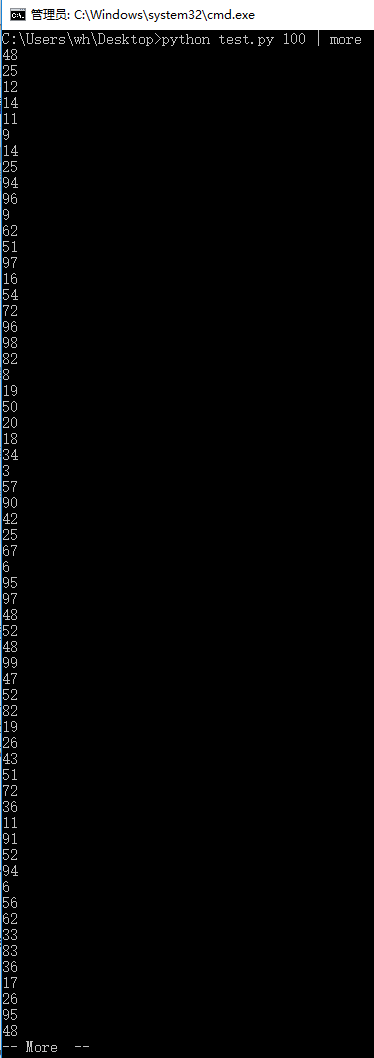使用操作系统实用程序sort排序输出数据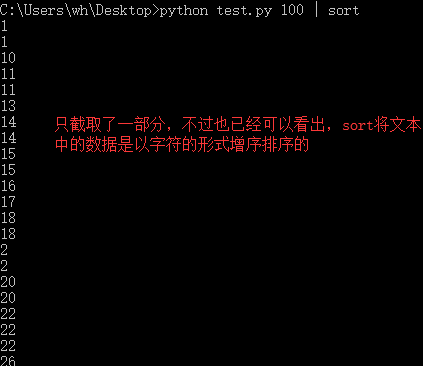more和sort都可以在一个语句中使用
填空题：1、2

print(value, ..., sep = ' ', end = '\n', file = sys.stdout, flush = False)
sep（分隔符，默认为空格）end（换行符，即输入的末尾是个啥）file（写入到指定文件流，默认为控制台sys.stdout）flush（指定是否强制写入到流）

1
>>> print(1,2,3,4,5,sep='-',end='!')
1-2-3-4-5!

2
>>> for i in range(10):
print(i,end=' ')

0 1 2 3 4 5 6 7 8 9

例题及上机实践：2~5
2. 尝试修改例6.2编写的命令行参数解析的程序，解析命令行参数所输入边长的值，计算并输出正方形的周长和面积

argparse模块用于解析命名的命令行参数，生成帮助信息的Python标准模块

例6.2：解析命令行参数所输入的长和宽的值，计算并输出长方形的面积
import argparse

parser = argparse.ArgumentParser()
parser.add_argument('--length', default = 10, type = int, help = '长度')
parser.add_argument('--width', default = 5, type = int, help = '宽度')

args = parser.parse_args()
area = args.length * args.width
print('面积 = ', area)

input()#加这一句是为了可以看到输出结果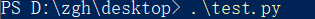输出：面积 = 50
如果在执行这个模块时，加入两个命令行参数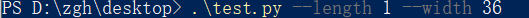输出：面积 = 36

基本上看了上面这个例子后，就可以理解argparse的用法了

本题代码：
import argparse

parser = argparse.ArgumentParser()
parser.add_argument('--length', default = 10, type = int, help = '长度')

args = parser.parse_args()
area = args.length ** 2
perimeter = 4 * args.length
print('面积 = {0}，周长 = {1}'.format(area,perimeter))

input()#加这一句是为了可以看到输出结果


在PowerShell中输入.\test.py 不给命令行参数，输出是以默认值来计算的 输出：面积 = 100，周长 = 40
给命令行参数：.\test.py --length 1 输出：面积 = 1，周长 = 4
3. 尝试修改例6.8编写读取并输出文本文件的程序，由命令行第一个参数确认所需输出的文本文件名

f = open(file, mode = 'r' , buffering = -1, encoding = None)
file是要打开或创建的文件名，如果文件不在当前路径，需指出具体路径mode是打开文件的模式，模式有： ‘r’（只读） ‘w’（写入，写入前删除就内容） ‘x’（创建新文件，如果文件存在，则导致FileExistsError） ‘a’（追加） ‘b’（二进制文件） ‘t’（文本文件，默认值） ‘+’（更新，读写）buffering表示是否使用缓存（缓存为-1，表示使用系统默认的缓冲区大小）encoding是文件的编码

例6.8：读取并输出文本文件
import sys

filename = sys.argv#就读取本文件，骚的呀皮
f = open(filename, 'r', encoding = 'utf-8')

line_no = 0
while True:
line_no += 1
if line:
print(line_no, ":", line)
else:
break
f.close()

输出（代码输出的就是本python文件）：
1 : import sys

2 :

3 : filename = sys.argv#就读取本文件，骚的呀皮

4 : f = open(filename, 'r', encoding = 'utf-8')

5 :

6 : line_no = 0

7 : while True:

8 :     line_no += 1

10 :     if line:

11 :         print(line_no, ":", line)

12 :     else:

13 :         break

14 : f.close()

15 :


本题代码：
对例题代码进行些许修改就可以了，首先将上例中的第二个语句改为：filename = sys.argv，再考虑下面怎么进行
准备一个用来测试的文件test.txt：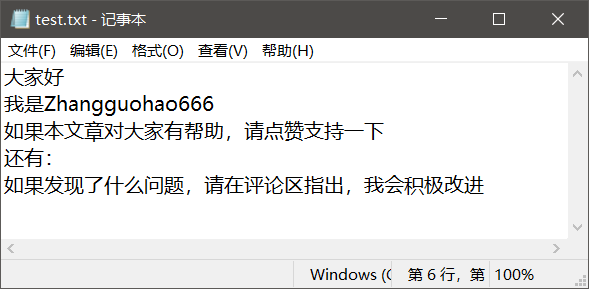对于这个文件要注意一点（你们很可能回出现这个问题！！！），win10默认创建的文本文件的字符编码是ANSI
代码怎么写，有两种：
将test.txt文本文件的编码修改为utf-8，代码如上所说 记事本方式打开test.txt文件，点击文件，点击另存为，看到下方的编码（修改为utf-8）test.txt就用默认的ANSI编码方式，再将上例代码的第三个语句修改为f = open(filename, 'r', encoding = 'ANSI')
在PowerShell中输入：./test.py test.txt 输出：
1 : ﻿大家好

2 : 我是Zhangguohao666

3 : 如果本文章对大家有帮助，请点赞支持一下

4 : 还有：

5 : 如果发现了什么问题，请在评论区指出，我会积极改进

4. 尝试修改例6.9编写利用with语句读取并输出文本文件的程序，由命令行第一个参数确认所需输出的文本文件名

为了简化操作，Python语言中与资源相关的对象可以实现上下文管理协议，可以使用with语句，确保释放资源。 with open(file,mode) as f:

例6.9：利用with语句读取并输出文本文件
import sys

filename = sys.argv

line_no = 0
with open(filename, 'r', encoding = 'utf-8') as f:
for line in f:
line_no += 1
print(line_no, ":", line)
f.close()


基本上，看这个例子，就可以上手with语句了

本题代码：
还是上一题准备的文本文件， 代码一（文本文件的编码为默认的ANSI）：
import sys

filename = sys.argv

line_no = 0
with open(filename, 'r', encoding = 'ANSI') as f:
for line in f:
line_no += 1
print(line_no, ":", line)
f.close()


代码二（将文本文件的编码修改为utf-8）：
import sys

filename = sys.argv

line_no = 0
with open(filename, 'r', encoding = 'utf-8') as f:
for line in f:
line_no += 1
print(line_no, ":", line)
f.close()


本题的输出，我再不要脸的放一次吧：
1 : ﻿大家好

2 : 我是Zhangguohao666

3 : 如果本文章对大家有帮助，请点赞支持一下

4 : 还有：

5 : 如果发现了什么问题，请在评论区指出，我会积极改进

5. 尝试修改例6.12编写标准输出流重定向的程序，从命令行第一个参数中获取n的值，然后将0-n，0-n的2倍值，2的0-n次幂的列表打印输出到out.log文件中
例6.12：从命令行第一个参数中获取n的值，然后将0-n，2的0-n次幂的列表打印输出到out.log文件中

标准输入流文件对象：sys.stdin， 默认值为sys.__stdin__标准输出流文件对象：sys.stdout， 默认值为sys.__stdout__错误输出流文件对象（标准错误流文件对象）：sys.stderr 默认值为sys.__stderr__

书中给的代码是这样的：
import sys

n = int(sys.argv)
power = 1
i = 0

f = open('out.log', 'w')
sys.stdout = f

while i <= n:
print(str(i), ' ', str(power))
power = 2*power
i += 1
sys.stdout = sys.__stdout__

如果使用的编辑器是PyCharm（现在大多数编辑器会帮你对代码进行优化和处理一些隐患），运行书中的这个代码没有问题。
但是： 若使用的编辑器是python自带的IDLE，运行这个代码有问题！
第一：out.log文件会生成，但是没有东西 （发现文件关闭不了（就是×不掉）， 确定是文件没关闭（f.close()）的原因）
第二：控制台没有输出’done’语句（估计是IDLE编辑器处理不了__stdout__这个值）
经过研究后，发现（基于IDLE编辑器）： 如果在上面的代码中加入f.close()后，该输入的东西都成功输入进out.log文件了， 但是， 还有一个问题 控制台依旧没有输出’done’语句 经过一步步的断点调试（就是手动写print） 发现sys.stdout = sys.__stdout__不会执行
然后进行改动后，就可以了，代码如下： （既然__stdout__不好使，就使用中间变量）
import sys

n = int(sys.argv)
power = 1
i = 0

output = sys.stdout
f = open('out.log', 'w')
sys.stdout = f

while i <= n:
print(str(i), ' ', str(power))
power = 2*power
i += 1

f.close()
sys.stdout = output
print('done!')#这一句是用来检测上面的代码是否成功执行



问题虽然解决，但是原因没有彻底弄清楚，求助。。。。。。

本题代码：
import sys

n = int(sys.argv)
power = 1
i = 0

output = sys.stdout
f = open('out.log', 'w')
sys.stdout = f

while i <= n:
print(str(i), ' ',  str(2*i),  ' ', str(power))
power = 2*power
i += 1

f.close()
sys.stdout = output
print('done!')#这一句是用来检测上面的代码是否成功执行


比如时输入的命令行参数是6 输出：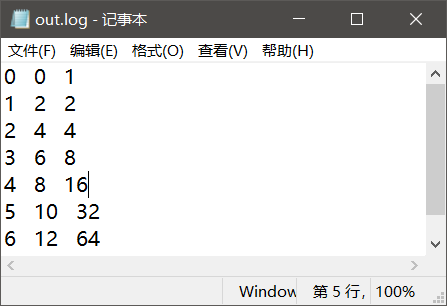案例研究：21点扑克牌游戏
https://blog.csdn.net/Zhangguohao666/article/details/103948545
通过21点扑克牌游戏的设计和实现，了解使用Python数据类型、控制流程和输入输出
第七章 错误和异常处理

Python语言采用结构化的异常处理机制捕获和处理异常

而我感觉，Python在这方面的知识点其实和Java的差不多
几个例题
一：程序的错误和异常处理
语法错误

指源代码中的拼写错误，这些错误导致Python编译器无法把Python源代码转换为字节码，故也称之为编译错误

运行时错误

在解释执行过程中产生的错误

例如：
程序中没有导入相关的模块，NameError程序中包括零除运算，ZeroDivisionError程序中试图打开不存在的文件，FileNotFoundError
逻辑错误

程序可以执行（程序运行本身不报错），但执行结果不正确。 对于逻辑错误，Python解释器无能为力，需要用户根据结果来调试判断

大部分由程序错误而产生的错误和异常一般由Python虚拟机自动抛出。另外，在程序中如果判断某种错误情况，可以创建相应的异常类的对象，并通过raise语句抛出
>>> a = -1
>>> if(a < 0): raise ValueError("数值不能为负数")

Traceback (most recent call last):
File "<pyshell#9>", line 1, in <module>
if(a < 0): raise ValueError("数值不能为负数")
ValueError: 数值不能为负数
>>>


在程序中的某个方法抛出异常后，Python虚拟机通过调用堆栈查找相应的异常捕获程序。如果找到匹配的异常捕获程序（即调用堆栈中的某函数使用try…except语句捕获处理），则执行相应的处理程序（try…except语句中匹配的except语句块）

如果堆栈中没有匹配的异常捕获程序，则Python虚拟机捕获处理异常，在控制台打印出异常的错误信息和调用堆栈，并中止程序的执行

二：try …except…else…finally
try:
可能产生异常的语句
except Exception1:
发生Exception1时执行的语句
except (Exception2,Exception3):
发生Exception2或Exception3时执行的语句
except Exception4 as e:
发生Exception4时执行的语句，Exception4的实例是e
except:
捕获其他所有异常
else:
无异常时执行的语句
finally:
不管异常发生与否都保证执行的语句

except语句可以写多个，但是要注意一点：系统是自上而下匹配发生的异常，所以用户需要将带有最具体的（即派生类程度最高的）异常类的except写在前面
三：创建自定义异常，处理应用程序中出现的负数参数的异常

自定义异常类一般继承于Exception或其子类。自定义异常类的名称一般以Error或Exception为后缀

>>> class NumberError(Exception):
def __init__(self,data):
Exception.__init__
(self,data)
self.data = data
def __str__(self):
return self.data + '：非法数值（<0）'

>>>
>>> def total(data):
total = 0
for i in data:
if i < 0: raise NumberError(str(i))
total += 1

>>>
>>> data1 = (44, 78, 90, 80, 55)
>>> print("sum: ",total(data1))
sum:  5
>>>
>>> data2 = (44, 78, 90, 80, -1)
>>> print("sum: ",total(data2))
Traceback (most recent call last):
File "<pyshell#24>", line 1, in <module>
print("sum: ",total(data2))
File "<pyshell#18>", line 4, in total
if i < 0: raise NumberError(str(i))
NumberError: -1：非法数值（<0）
>>>

四：断言处理
用户在编写程序时，在调试阶段往往需要判断代码执行过程中变量的值等信息：
用户可以使用print()函数打印输出结果也可以通过断点跟踪调试查看变量但使用断言更加灵活
assert语句和AssertionError
断言的声明：
assert <布尔表达式> 即：if __debug__: if not testexpression: raise AssertionErrorassert <布尔表达式>,<字符串表达式> 即：if __debug__: if not testexpression: raise AssertionError(data) 字符串表达式（即data）是断言失败时输出的失败消息
__debug__也是布尔值，Python解释器有两种：调试模式和优化模式
调试模式：__debug__ == True优化模式：__debug__ == False
在学习中，对于执行一个py模块（比如test.py）我们通常在cmd中这么输入python test.py，而这默认是调试模式。 如果我们要使用优化模式来禁用断言来提高程序效率，我们可以加一个运行选项-O，在控制台中这么输入python -O test.py
看一下断言的示例吧，理解一下用法：
a =int(input("a: "))
b =int(input("b: "))
assert b != 0, '除数不能为零'
c = a/b
print("a/b = ", c)

cmd出场： 输入正确数值时：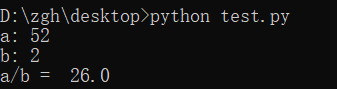输入错误数值时：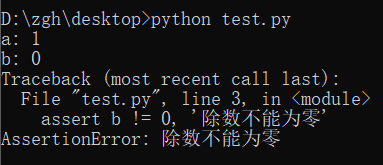禁用断言，并且输入错误数值时：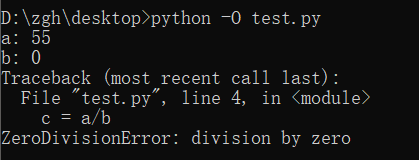案例研究：使用调试器调试Python程序
https://blog.csdn.net/Zhangguohao666/article/details/103948568
了解使用Python调试器调试程序的方法
第八章 函数和函数式编程

一些知识点总结和几个例题
Python中函数的分类：
内置函数 在程序中可以直接使用标准库函数 Python语言安装程序同时会安装若干标准库，例如math、random等第三方库函数 Python社区提供了许多其它高质量的库，在下载、安装这些库后，通过import语句可以导入库用户自定义函数
函数名为有效的标识符（命名规则为全小写字母，可以使用下划线增加可阅读性，例如my_func()）函数可以使用return返回值 如果函数体中包含return语句，则返回值， 否则不返回，即返回值为空（None），无返回值的函数相当于其它编程语言中的过程
调用函数之前程序必须先执行def语句，创建函数对象
内置函数对象会自动创建import导入模块时会执行模块中的def语句，创建模块中定义的函数Python程序结构顺序通常为import语句>函数定义>全局代码
一：产生副作用的函数，纯函数
打印等腰三角形
n = int(input("行数："))

def print_star(n):
print((" * " * n).center(50))

for i in range(1, 2*n, 2):
print_star(i)

输出：
行数：5
*
*  *  *
*  *  *  *  *
*  *  *  *  *  *  *
*  *  *  *  *  *  *  *  *

上面代码中的print_star()是一个产生副作用的函数，其副作用是向标准输出写入若干星号

副作用：例如读取键盘输入，产生输出，改变系统的状态等在一般情况下，产生副作用的函数相当于其它程序设计语言中的过程，可以省略return语句

定义计算并返回第n阶调和数（1+1/2+1/3+…+1/n）的函数，输出前n个调和数
def harmonic(n):
total = 0.0
for i in range(1, n+1):
total += 1.0/i

n = int(input("n："))

print("输出前n个调和数的值：")
for i in range(1, n+1):
print(harmonic(i))

输出：
 n：8
输出前n个调和数的值：
1.0
1.5
1.8333333333333333
2.083333333333333
2.283333333333333
2.4499999999999997
2.5928571428571425
2.7178571428571425

上面代码中的harmonic()是纯函数

纯函数：给定同样的实际参数，其返回值唯一，且不会产生其它的可观察到的副作用

注意：编写同时产生副作用和返回值的函数通常被认为是不良编程风格，但有一个例外，即读取函数。例如，input()函数既可以返回一个值，又可以产生副作用（从标准输入中读取并消耗一个字符串）
二：传递不可变对象、可变对象的引用
实际参数值默认按位置顺序依次传递给形式参数。如果参数个数不对，将会产生错误
在调用函数时：
若传递的是不可变对象（例如：int、float、bool、str对象）的引用，则如果函数体中修改对象的值，其结果实际上是创建了一个新的对象
i = 1

def func(i,n):
i += n
return i

print(i)#1
func(i,10)
print(i)#1

执行函数func()后，i依旧为1，而不是11
若传递的是可变对象（例如：list对象）的引用，则在函数体中可以直接修改对象的值
import random

def shuffle(a):
n = len(a)
for i in range(n):
r = random.randrange(i,n)
a[i],a[r] = a[r],a[i]

a = [1,2,3,4,5]
print("初始：",a)
shuffle(a)
print("调用函数后：",a)

输出：
初始： [1, 2, 3, 4, 5]
调用函数后： [1, 5, 4, 3, 2]

三：可选参数，命名参数，可变参数，强制命名参数
可选参数
在声明函数时，如果希望函数的一些参数是可选的，可以在声明函数时为这些参数指定默认值
>>> def babbles(words, times=1):
print(words * times)

>>> babbles('Hello')
Hello
>>>
>>> babbles("Hello", 2)
HelloHello
>>>

注意到一点：必须先声明没有默认值的形参，然后再声明有默认值的形参，否则报错。 这是因为在函数调用时默认是按位置传递实际参数的。
怎么理解上面那句话呢？
默认是按位置传递实际参数（如果有默认值的形参在左边，无默认值的形参在右，那么在调用函数时，你的实参该怎么传递呢？）
命名参数
位置参数：当函数调用时，实参默认按位置顺序传递形参命名参数（关键字参数）：按名称指定传入的参数 参数按名称意义明确 传递的参数与顺序无关 如果有多个可选参数，则可以选择指定某个参数值
基于期中成绩和期末成绩，按照指定的权重计算总评成绩
>>> def my_sum(mid_score, end_score, mid_rate = 0.4):
score = mid_score*mid_rate + end_score*(1-mid_rate)
print(format(score,'.2f'))

>>> my_sum(80,90)
86.00
>>> my_sum(mid_score = 80,end_score = 90)
86.00
>>> my_sum(end_score = 90,mid_score = 80)
86.00
>>>

可变参数
在声明函数时，可以通过带星号的参数（例如：def func(* param)）向函数传递可变数量的实参，调用函数时，从那一点后所有的参数被收集为一个元组在声明函数时，可以通过带双星号的参数（例如：def func(** param)）向函数传递可变数量的实参，调用函数时，从那一点后所有的参数被收集为一个字典
利用带星的参数计算各数字的累加和
>>> def my_sum(a,b,*c):
total = a+b
for i in c:
total += i

>>> print(my_sum(1,2))
3
>>> print(my_sum(1,2,3,4,5,6))
21

利用带星和带双星的参数计算各数字的累加和
>>> def my_sum(a,b,*c,**d):
total = a+b
for i in c:
total += i
for key in d:
total += d[key]

>>> print(my_sum(1,2))
3
>>> print(my_sum(1,2,3,4))
10
>>> print(my_sum(1,2,3,4,male=1,female=2))
13

强制命名参数
在带星号的参数后面声明参数会导致强制命名参数（Keyword-only），然后在调用时必须显式使用命名参数传递值因为按位置传递的参数默认收集为一个元组，传递给前面带星号的可变参数
>>> def my_sum(*, mid_score, end_score, mid_rate = 0.4):
score = mid_score*mid_rate + end_score*(1-mid_rate)
print(format(score,'.2f'))

>>> my_sum(mid_score=80,end_score=90)
86.00
>>> my_sum(end_score=90,mid_score=80)
86.00
>>> my_sum(80,90)
Traceback (most recent call last):
File "<pyshell#47>", line 1, in <module>
my_sum(80,90)
TypeError: my_sum() takes 0 positional arguments but 2 were given
>>>

四：全局语句global示例，非局部语句nonlocal示例，输出局部变量和全局变量
在函数体中可以引用全局变量，但是要为定义在函数外的全局变量赋值，需要使用global语句
pi = 2.1415926
e = 2.7182818

def my_func():
global pi
pi = 3.14
print("global pi = ", pi)
e = 2.718
print("local e = ", e)

print('module pi = ', pi)
print('module e = ', e)
my_func()
print('module pi = ', pi)
print('module e = ', e)

输出：
module pi =  2.1415926
module e =  2.7182818
global pi =  3.14
local e =  2.718
module pi =  3.14
module e =  2.7182818

在函数体中可以定义嵌套函数，在嵌套函数中如果要为定义在上级函数体的局部变量赋值，可以使用nonlocal
def outer_func():
tax_rate = 0.17
print('outer function tax rate is ',tax_rate)
def inner_func():
nonlocal tax_rate
tax_rate = 0.01
print('inner function tax rate is ',tax_rate)
inner_func()
print('outer function tax rate is ',tax_rate)

outer_func()

输出：
outer function tax rate is  0.17
inner function tax rate is  0.01
outer function tax rate is  0.01

输出局部变量和全局变量
内置函数locals()，局部变量列表内置函数globals()，全局变量列表
五：获取和设置最大递归数
在sys模块中，函数getrecursionlimit()和setrecursionlimit()用于获取和设置最大递归次数
>>> import sys
>>> sys.getrecursionlimit()
1000
>>> sys.setrecursionlimit(666)
>>> sys.getrecursionlimit()
666
>>>

六：三个有趣的内置函数：eval()、exec()、compile()
eval
对动态表达式进行求值，返回值eval(expression, globals=None, locals=None) expression是动态表达式的字符串 globals和locals是求值时使用的上下文环境的全局变量和局部变量，如果不指定，则使用当前运行上下文
>>> x = 2
>>> str_func = input("请输入表达式：")
请输入表达式：x**2+2*x+1
>>> eval(str_func)
9
>>>

exec
可以执行动态表达式，不返回值exec(str, globals=None, locals=None)
>>> exec("for i in range(10): print(i, end=' ')")
0 1 2 3 4 5 6 7 8 9
>>>

compile
编译代码为代码对象，可以提高效率compile(source, filename, mode) source为代码语句的字符串；如果是多行语句，则每一行的结尾必须有换行符\n filename为包含代码的文件 mode为编码方式，可以为'exec'（用于语句序列的执行），可以为'eval'（用于表达式求值），可以为'single'（用于单个交互语句）
>>> co = compile("for i in range(10): print(i, end=' ')", '', 'exec')
>>> exec(co)
0 1 2 3 4 5 6 7 8 9
>>>

七：map()，filter()
map(f, iterable,…)，将函数f应用于可迭代对象，返回结果为可迭代对象
示例1：
>>> def is_odd(x):
return x%2 == 1

>>> list(map(is_odd,range(5)))
[False, True, False, True, False]
>>>

示例2：
>>> list(map(abs,[1,-2,3,-4,5,-6]))
[1, 2, 3, 4, 5, 6]
>>>

示例3：
>>> list(map(str,[1,2,3,4,5]))
['1', '2', '3', '4', '5']
>>

示例4：
>>> def greater(x,y):
return x>y

>>> list(map(greater,[1,5,7,3,9],[2,8,4,6,0]))
[False, False, True, False, True]
>>>

filter(f, iterable)，将函数f应用于每个元素，然后根据返回值是True还是False决定保留还是丢弃该元素，返回结果为可迭代对象
示例1（返回个位数的奇数）：
>>> def is_odd(x):
return x%2 == 1

>>> list(filter(is_odd, range(10)))
[1, 3, 5, 7, 9]
>>>

示例2（返回三位数的回文）：
>>> list(filter(is_palindrome, range(100, 1000)))
[101, 111, 121, 131, 141, 151, 161, 171, 181, 191, 202, 212, 222, 232, 242, 252, 262, 272, 282, 292, 303, 313, 323, 333, 343, 353, 363, 373, 383, 393, 404, 414, 424, 434, 444, 454, 464, 474, 484, 494, 505, 515, 525, 535, 545, 555, 565, 575, 585, 595, 606, 616, 626, 636, 646, 656, 666, 676, 686, 696, 707, 717, 727, 737, 747, 757, 767, 777, 787, 797, 808, 818, 828, 838, 848, 858, 868, 878, 888, 898, 909, 919, 929, 939, 949, 959, 969, 979, 989, 999]
>>>

八：Lambda表达式和匿名函数

匿名函数广泛应用于需要函数对象作为参数、函数比较简单并且只使用一次的场合

格式：
lambda arg1,arg2... : <expression>

其中，arg1、arg2等为函数的参数，<expression>为函数的语句，其结果为函数的返回值
示例1（计算两数之和）：
>>> f = lambda x,y : x+y
>>> type(f)
<class 'function'>
>>> f(1,1)
2
>>>

示例2（返回奇数）：
>>> list(filter(lambda x:x%2==1, range(10)))
[1, 3, 5, 7, 9]
>>>

示例3（返回非空元素）：
>>> list(filter(lambda s:s and s.strip(), ['A', '', 'B', None, 'C', ' ']))
['A', 'B', 'C']
>>>

补充：
strip()用来去除头尾字符、空白符（\n,\r,\t,’’,即换行、回车、制表、空格）lstrip()用来去除开头字符、空白符rstrip()用来去除结尾字符、空白符
再补充一点：
\n到下一行的开头\r回到这一行的开头
示例4（返回大于0的元素）：
>>> list(filter(lambda x:x>0, [1,0,-2,8,5]))
[1, 8, 5]
>>>

示例5（返回元素的平方）：
>>> list(map(lambda x:x*x, range(10)))
[0, 1, 4, 9, 16, 25, 36, 49, 64, 81]
>>>

九：operator模块和操作符函数

Python内置操作符的函数接口，它定义了对应算术和比较等操作的函数，用于map()、filter()等需要传递函数对象作为参数的场合，可以直接使用而不需要使用函数定义或者Lambda表达式，使得代码更加简洁

示例1（concat(x,y)对应于x+y）：
>>> import operator
>>> a = 'hello'
>>>> operator.concat(a, ' world')
'hello world'

实例2（operator.gt对应于操作符>）：
>>> import operator
>>> list(map(operator.gt, [1,5,7,3,9],[2,8,4,6,0]))
[False, False, True, False, True]
>>>

十：functools.reduce()，偏函数functools.partial()，sorted()
functools.reduce()

functools.reduce(func, iterable[, iterable[, initializer]])
使用指定的带两个参数的函数func对一个数据集合的所有数据进行下列操作：使用第一个和第二个数据作为参数用func()函数运算，得到的结果再与第三个数据作为参数用func()函数运算，依此类推，最后得到一个结果可选的initialzer为初始值

示例：
>>> import functools,operator
15
25
5050
>>>
>>> functools.reduce(operator.mul, range(1,11))
3628800

偏函数functools.partial()

functools.partial(func, *arg, **keywords)
通过把一个函数的部分参数设置为默认值的方式返回一个新的可调用（callable）的partial对象主要用于设置预先已知的参数，从而减少调用时传递参数的个数

示例（2的n次方）：
>>> import functools,math
>>> pow2 = functools.partial(math.pow, 2)
>>> list(map(pow2, range(11)))
[1.0, 2.0, 4.0, 8.0, 16.0, 32.0, 64.0, 128.0, 256.0, 512.0, 1024.0]
>>>

十一：sorted()

sorted(iterable, *, key=None, reverse=False)
iterable是待排序的可迭代对象key是比较函数（默认为None，按自然顺序排序）reverse用于指定是否逆序排序

示例1（数值。默认自然排序）：
>>> sorted([1,6,4,-2,9])
[-2, 1, 4, 6, 9]
>>> sorted([1,6,4,-2,9], reverse=True)
[9, 6, 4, 1, -2]
>>> sorted([1,6,4,-2,9], key=abs)
[1, -2, 4, 6, 9]

示例2（字符串，默认按字符串字典序排序）：
>>> sorted(['Dod', 'cat', 'Rabbit'])
['Dod', 'Rabbit', 'cat']
>>> sorted(['Dod', 'cat', 'Rabbit'], key=str.lower)
['cat', 'Dod', 'Rabbit']
>>> sorted(['Dod', 'cat', 'Rabbit'], key=len)
['Dod', 'cat', 'Rabbit']

示例3（元组，默认按元组的第一个元素排序）：
>>> sorted([('Bob', 75), ('Adam', 92), ('Lisa', 88)])
[('Adam', 92), ('Bob', 75), ('Lisa', 88)]
>>> sorted([('Bob', 75), ('Adam', 92), ('Lisa', 88)], key=lambda t:t)
[('Bob', 75), ('Lisa', 88), ('Adam', 92)]

十二：函数装饰器
这玩意就很有意思了，很Java语言中的注解是很相像的
示例1：
import time,functools

def timeit(func):
def wrapper(*s):
start = time.perf_counter()
func(*s)
end = time.perf_counter()
print('运行时间：', end - start)
return wrapper

@timeit
def my_sum(n):
sum = 0
for i in range(n): sum += i
print(sum)

if __name__ == '__main__':
my_sum(10_0000)

结果：
4999950000
运行时间： 0.013929100000000028

怎么理解上面的代码呢？
首先，timeit()返回的是wrapper，而不是执行（没有小括号）@timeit相当于，在调用my_sum()的前一刻，会执行这么个语句：my_sum = timeit(my_sum)
示例2：
def makebold(fn):
def wrapper(*s):
return "<b>" + fn(*s) + "</b>"
return wrapper

def makeitalic(fn):
def wrapper(*s):
return "<i>" + fn(*s) + "</i>"
return wrapper

@makebold
@makeitalic
def htmltags(str1):
return str1

print(htmltags('Hello'))


输出：
<b><i>Hello</i></b>

选择题：1~5
1
>>> print(type(lambda:None))
<class 'function'>

2
>>> f = lambda x,y:x*y
>>> f(12, 34)
408

3
>>> f1 = lambda x:x*2
>>> f2 = lambda x:x**2
>>> print(f1(f2(2)))
8

4
>>> def f1(p, **p2):
print(type(p2))

>>> f1(1, a=2)
<class 'dict'>

5
>>> def f1(a,b,c):
print(a+b)

>>> nums = (1,2,3)
>>> f1(*nums)
3

思考题：4~11
4
>>> d = lambda p:p*2
>>> t = lambda p:p*3
>>> x = 2
>>> x = d(x)
>>> x = t(x)
>>> x = d(x)
>>> print(x)
24

5
>>> i = map(lambda x:x**2, (1,2,3))
>>> for t in i:
print(t, end=' ')

1 4 9

6
>>> def f1():
"simple function"
pass

>>> print(f1.__doc__)
simple function

7
>>> counter = 1
>>> num = 0
>>> def TestVariable():
global counter
for i in (1, 2, 3) : counter += 1
num = 10

>>> TestVariable()
>>> print(counter, num)
4 0

8
>>> def f(a,b):
if b==0 : print(a)
else : f(b, a%b)

>>> print(f(9,6))
3
None

求最大公约数
9
>>> def aFunction():
"The quick brown fox"
return 1

>>> print(aFunction.__doc__[4:9])
quick

10
>>> def judge(param1, *param2):
print(type(param2))
print(param2)

>>> judge(1, 2, 3, 4, 5)
<class 'tuple'>
(2, 3, 4, 5)

11
>>> def judge(param1, **param2):
print(type(param2))
print(param2)

>>> judge(1, a=2, b=3, c=4, d=5)
<class 'dict'>
{'a': 2, 'b': 3, 'c': 4, 'd': 5}

上机实践：2~5
2. 编写程序，定义一个求阶乘的函数fact(n)，并编写测试代码，要求输入整数n（n>=0）。请分别使用递归和非递归方式实现
递归方式：
def fact(n):
if n == 0 :
return 1
return n*fact(n-1)

n = int(input("请输入整数n（n>=0）："))
print(str(n)+" ! =  " + str(fact(n)))


非递归方式：
def fact(n):
t = 1
for i in range(1,n+1):
t *= i
return t

n = int(input("请输入整数n（n>=0）："))
print(str(n)+" ! =  " + str(fact(n)))


输出：
请输入整数n（n>=0）：5
5 ! =  120

3. 编写程序，定义一个求Fibonacci数列的函数fib(n)，并编写测试代码，输出前20项（每项宽度5个字符位置，右对齐），每行输出10个。请分别使用递归和非递归方式实现
递归方式：
def fib(n):
if (n == 1 or n == 2):
return 1
return fib(n-1)+fib(n-2)

for i in range(1,21):
print(str(fib(i)).rjust(5,' '),end = ' ')
if i %10 == 0:
print()

非递归方式：
def fib(n):
if (n == 1 or n == 2):
return 1
n1 = n2 = 1
for i  in range(3,n+1):
n3 = n1+n2
n1 = n2
n2 = n3
return n3

for i in range(1,21):
print(str(fib(i)).rjust(5,' '),end = ' ')
if i %10 == 0:
print()

输出：
    1     1     2     3     5     8    13    21    34    55
89   144   233   377   610   987  1597  2584  4181  6765

4. 编写程序，利用可变参数定义一个求任意个数数值的最小值的函数min_n(a,b,*c)，并编写测试代码。例如对于“print(min_n(8, 2))”以及“print(min_n(16, 1, 7, 4, 15))”的测试代码
def min_n(a,b,*c):
min_number = a if(a < b) else b
for n in c:
if n < min_number:
min_number = n
return min_number

print(min_n(8, 2))
print(min_n(16, 1, 7, 4, 15))

输出：
2
1

5. 编写程序，利用元组作为函数的返回值，求序列类型中的最大值、最小值和元素个数，并编写测试代码，假设测试代码数据分别为s1=[9, 7, 8, 3, 2, 1, 55, 6]、s2=[“apple”, “pear”, “melon”, “kiwi”]和s3="TheQuickBrownFox"
def func(n):
return (max(n),min(n),len(n))

s1=[9, 7, 8, 3, 2, 1, 55, 6]
s2=["apple", "pear", "melon", "kiwi"]
s3="TheQuickBrownFox"

for i in (s1,s2,s3):
print("list = ", i)
t = func(i)
print("最大值 = {0}，最小值 = {1}，元素个数 = {2}".format(t, t, t))

输出：
list =  [9, 7, 8, 3, 2, 1, 55, 6]
最大值 = 55，最小值 = 1，元素个数 = 8
list =  ['apple', 'pear', 'melon', 'kiwi']
最大值 = pear，最小值 = apple，元素个数 = 4
list =  TheQuickBrownFox
最大值 = x，最小值 = B，元素个数 = 16

案例研究：井字棋游戏
https://blog.csdn.net/Zhangguohao666/article/details/103280740
了解Python函数的定义和使用

由于本文的内容太多了，导致了两个很不好的结果， 一是：在网页中打开本篇博客的加载时间太长了，明显的卡顿很影响阅读体验； 二是：本人在对本篇文章进行更新或者修改内容时，卡的要死。 遂， 将本文第八章后面的很多内容拆分到新的文章中，望大家理解

第九章 面向对象的程序设计

《Python程序设计与算法基础教程（第二版）》江红 余青松，第九章课后习题答案
第十章 模块和客户端

《Python程序设计与算法基础教程（第二版）》江红 余青松，第十章课后习题答案
第十一章 算法与数据结构基础

《Python程序设计与算法基础教程（第二版）》江红 余青松，第十一章课后习题答案
第十二章 图形用户界面

我对图形用户界面基本无兴趣，无特殊情况，基本不打算碰这方面内容
案例研究：简易图形用户界面计算器
Python实现简易图形用户界面计算器
第十三章 图形绘制

与上一章相同，我对于图形绘制的兴趣也基本没有，尝试做了2-7题，就完全没兴趣做下去了
图形绘制模块：tkinter
2. 参考例13.2利用Canvas组件创建绘制矩形的程序，尝试改变矩形边框颜色以及填充颜色
from tkinter import *

root = Tk()
c = Canvas(root, bg = 'white', width = 130, height = 70)
c.pack()

c.create_rectangle(10, 10, 60, 60, fill = 'red')
c.create_rectangle(70, 10, 120, 60, fill = 'green', outline = 'blue', width = 5)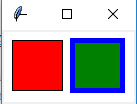创建画布对象：
root = Tk() 创建一个Tk根窗口组件rootc = Canvas(root, bg = 'white', width = 130, height = 70) 创建大小为200 * 100、背景颜色为白色的画布c.pack() 调用组件pack()方法，调整其显示位置和大小
绘制矩形：
c.create_rectangle(x0, y0, x1, y1, option, ...)

（x0，y0）是左上角的坐标（x1，y1）是右下角的坐标c.create_rectangle(70, 10, 120, 60, fill = 'green', outline = 'blue', width = 5) 用蓝色边框、绿色填充矩形，边框宽度为5
3. 参考例13.3利用Canvas组件创建绘制椭圆的程序，尝试修改椭圆边框样式、边框颜色以及填充颜色
from tkinter import *

root = Tk()
c = Canvas(root, bg = 'white', width = 280, height = 70)
c.pack()

c.create_oval(10, 10, 60, 60, fill = 'green')
c.create_oval(70, 10, 120, 60, fill = 'green', outline = 'red', width = 5)
c.create_oval(130, 25, 180, 45, dash = (10,))
c.create_oval(190, 10, 270, 50, dash = (1,), width = 2)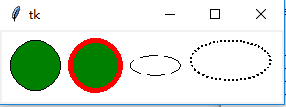绘制椭圆
c.create_oval(x0, y0, x1, y1, option, ...)

（x0，y0）是左上角的坐标（x1，y1）是右下角的坐标c.create_oval(70, 10, 120, 60, fill = 'green', outline = 'red', width = 5) 绿色填充、红色边框，宽度为5c.create_oval(130, 25, 180, 45, dash = (10,)) 虚线椭圆
4. 参考例13.4利用Canvas组件创建绘制圆弧的程序，尝试修改圆弧样式、边框颜色以及填充颜色
from tkinter import *

root = Tk()
c = Canvas(root, bg = 'white', width = 250, height = 70)
c.pack()

c.create_arc(10, 10, 60, 60, style = ARC)
c.create_arc(70, 10, 120, 60, style = CHORD)
c.create_arc(130, 10, 180, 60, style = PIESLICE)
for i in range(0, 360, 60):
c.create_arc(190, 10, 240, 60, fill = 'green', outline = 'red', start = i, extent = 30)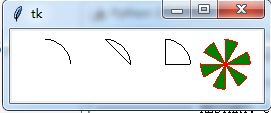绘制圆弧：
c.create_arc(x0, y0, x1, y1, option, ...)

（x0，y0）是左上角的坐标（x1，y1）是右下角的坐标选项start（开始角度，默认为0）和extend（圆弧角度，从start开始逆时针旋转，默认为90度）决定圆弧的角度范围选项start用于设置圆弧的样式
5. 参考例13.5利用Canvas组件创建绘制线条的程序，尝试修改线条样式和颜色
from tkinter import *

root = Tk()
c = Canvas(root, bg = 'white', width = 250, height = 70)
c.pack()

c.create_line(10, 10, 60, 60, arrow = BOTH, arrowshape = (3, 4, 5))
c.create_line(70, 10, 95, 10, 120, 60, fill = 'red')
c.create_line(130, 10, 180, 10, 130, 60, 180, 60, fill = 'green', width = 10, arrow = BOTH, joinstyle = MITER)
c.create_line(190, 10, 240, 10, 190, 60, 240, 60, width = 10)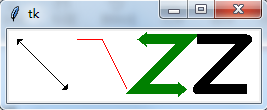绘制线条：
c.create_line(x0, y0, x1, y1, ..., xn, yn, option, ...)

（x0，y0）,（x1，y1），…，（xn，yn）是线条上各个点的坐标
6. 参考例13.6利用Canvas组件创建绘制多边形的程序，尝试修改多边形的形状、线条样式和填充颜色
from tkinter import *

root = Tk()
c = Canvas(root, bg = 'white', width = 250, height = 70)
c.pack()

c.create_polygon(35, 10, 10, 60, 60, 60, fill = 'red', outline = 'green')
c.create_polygon(70, 10, 120, 10, 120, 60, fill = 'white', outline = 'blue')
c.create_polygon(130, 10, 180, 10, 180, 60, 130, 60, outline = 'blue')
c.create_polygon(190, 10, 240, 10, 190, 60, 240, 60, fill = 'white', outline = 'black')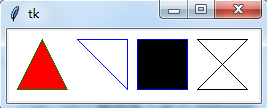绘制多边形：
c.create_polygon(x0, y0, x1, y1, ..., option, ...)

（x0，y0）,（x1，y1），…，（xn，yn）是多边形上各个顶点的坐标
7. 参考例13.7利用Canvas组件创建绘制字符串和图形的程序，绘制y = cos(x) 的图形
绘制字符串：
c.create_text(x, y, option, ...)

（x，y）是字符串放置的中心位置
y = sin(x)
from tkinter import *
import math

WIDTH, HEIGHT = 510, 210
ORIGIN_X, ORIGIN_Y = 2, HEIGHT/2 #原点

SCALE_X, SCALE_Y = 40, 100 #x轴、y轴缩放倍数
ox, oy = 0, 0
x, y = 0, 0
arc = 0 #弧度
END_ARC = 360 * 2 #函数图形画两个周期

root = Tk()
c = Canvas(root, bg = 'white', width = WIDTH, height = HEIGHT)
c.pack()

c.create_text(200, 20, text = 'y = sin(x)')
c.create_line(0, ORIGIN_Y, WIDTH, ORIGIN_Y)
c.create_line(ORIGIN_X, 0, ORIGIN_X, HEIGHT) #绘制x轴，y轴
for i in range(0, END_ARC+1, 10):
arc = math.pi * i / 180
x = ORIGIN_X + arc * SCALE_X
y = ORIGIN_Y - math.sin(arc) * SCALE_Y
c.create_line(ox, oy, x, y)
ox, oy = x, y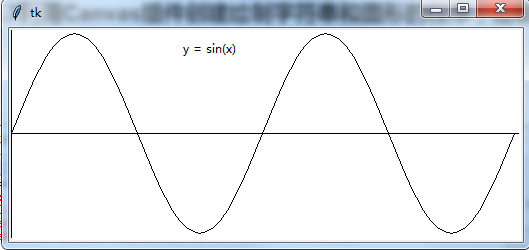y = cos(x)
from tkinter import *
import math

WIDTH, HEIGHT = 510, 210
ORIGIN_X, ORIGIN_Y = 2, HEIGHT/2 #原点

SCALE_X, SCALE_Y = 40, 100 #x轴、y轴缩放倍数
ox, oy = 0, 0
x, y = 0, 0
arc = 0 #弧度
END_ARC = 360 * 2 #函数图形画两个周期

root = Tk()
c = Canvas(root, bg = 'white', width = WIDTH, height = HEIGHT)
c.pack()

c.create_text(200, 20, text = 'y = cos(x)')
c.create_line(0, ORIGIN_Y, WIDTH, ORIGIN_Y)
c.create_line(ORIGIN_X, 0, ORIGIN_X, HEIGHT)
for i in range(0, END_ARC+1, 10):
arc = math.pi * i / 180
x = ORIGIN_X + arc * SCALE_X
y = ORIGIN_Y - math.cos(arc) * SCALE_Y
c.create_line(ox, oy, x, y)
ox, oy = x, y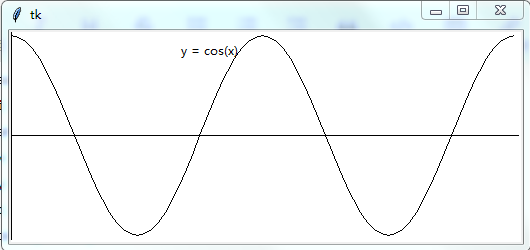图形绘制模块：turtle
…

后面章节内容：未完待续…
第十四章 数值日期和时间处理

第十五章 字符串和文本处理

第十六章 文件和数据交换

第十七章 数据访问

第十八章 网络编程和通信

第十九章 并行计算：进程、线程和协程

第二十章 系统管理
展开全文• 形式语言与自动机，图灵机的C++代码实现，用作转换器，有计算功能，X的y次幂turing
• 该捆绑软件中的所有转换器都采用from2to（）的形式。 示例：将 700 千帕转换为每平方英寸磅： kPa2psi(700) 答案 = 101.5264 支持的单位及其缩写： 加速： 平方厘米每秒 (cmps2) 地球重力 (G) 英尺每秒平方 ...matlab
• 验证用户输入的是否位数字，若是数字则求平方，若不是数字则给出提示。 运算符 a.数学运算符 + - * / % ++ – +：表示数学加法，或者字符串连接运输 -、*：若字符串参与，将数值字符串以数字参与运算 /：java...
1.JS概述 1）概念 js全程：JavaScript(一门客户端脚本语言) a.js可以直接嵌入在html页面中，由浏览器解释执行，不进行预编译 b.用于向页面添加动态交互行为 c.具有与java语言类似的语法，一种网页编程语言
操作方向： —操作DOM元素（html元素） —操作BOM元素（浏览器对象）
2）js特点： 1.解释执行：不需要预编译，逐行执行 2.基于对象：内置了大量的现成对象
3）作用 a.实现客户端数据简单计算 b.客户端表单合法性验证 c.添加浏览器事件的突发 d.网页特殊显示效果的制作 e.实现服务器的异步数据提交
4）遵循es规范（es6） a.严格区分大小写 b.数字、字母、下划线、\$组成，不能用数字开头 c.驼峰命名法
5）写法 a.行内式（定义事件） 在事件中定义操作，直接写js代码 alert(); 弹出警告框 事件： onclick 鼠标点击 ondblclick 鼠标双击 onmouseover 鼠标移入 onmouseout 鼠标移出 onkeydown 键盘按下 onkeyup 键盘抬起 onblur 失去焦点 onfocus 获取焦点 写法： οnclick=“函数()”; 事件=“事件触发的函数”;
b.内部式（嵌入式） 嵌入在页面的
c.外部式(文件调用) js代码位于单独的.js文件中（注意保存的编码） 写法： step1：在js文件中写js代码（定义行数） step2：将.js文件引入到当前页面中  step3：在html行内添加事件，调用函数
js注释 单行：//注释文字 多行：/注释文字/
js控制台： 不需要页面也可以直接在控制台（console）写js代码 F12（右击检查）---->打开控制台 Enter回车键：执行 Shift+Enter：换行
console.log(内容);//将内容打印到控制台
2.js组成 1）变量（var let const 无） js是弱类型语言，使用var关键字声明变量 刑如： var a = 1; var s = “李四”;
拆： var a;//a没有类型 var a=1;//初始化a才会有类型，a为number类型 a=“李四”;//a为String类型
typeof 变量名;//用来验证变量名
注意： 1.js是弱类型语言，不同的变量类型会根据数值自动给定类型 2.变量没有类型，统一用关键字var声明，变量值是有类型的 3.使用“=”进行赋值，没有初始化的变量自动取值为undefined 4.js存在变量声明提升（js会根据不同的值自动转换为对应的数据类型）
js数据类型 a.基本类型number、string，boolean number：不区分整数和小数
 string：一对引号包裹的字符串（单双引都可以）
----单双引号只能交替引用，特殊字符需要转义   \n  \t  \s
常用函数：
str.indexof("",from[int index]);
返回指定字符串在str中第一次出现的位置（从from开始第一次出现的位置）
str.replace("","");
将指定字符串替换为指定字符串，只替换第一个，属于不完全替换

boolean:仅有两个值，true、false
可以参与运算，自动作为数值，true值为1，false为0

b.特殊类型undefined、null undefined 变量声明未初始化，该变量的值和类型均为undefined null 代表"无值"或者"无对象"，可以通过一个变量赋值null来清空变量内容
c.对象类型object 内置对象 外部对象 自定义对象
数据类型转换： a.自动转换（直接转换属于默认规则） number+string=string boolean+string=string boolean+number=number boolean+boolean=number
b.强制转换（利用类型转换函数） toString();任何类型转换成string类型
parseInt();强转为整数，全局函数，具有截取功能
parseFloat();强转为小数，全局函数，具有截取功能
NaN:Not a Number(不是数字，不能转换成数字)
isNaN();全局函数，判断是否为非数字（true表示非数字，false表示数字）
案例1： 验证用户输入的是否位数字，若是数字则求平方，若不是数字则给出提示。
运算符 a.数学运算符 + - * / % ++ – +：表示数学加法，或者字符串连接运输 -、*：若字符串参与，将数值字符串以数字参与运算 /：java中7/2=3，js中7/2=3.5
b.关系运算  > >= < <= == !=
==：只比较值，不比较类型
===：比较类型和值

c.逻辑运算符&&(与) ||(或) !(非)
短路逻辑：结果为boolean类型，true或者false
非短路逻辑：结果为number类型（1或者0）

d.三元运算符（三目）
语法：判断表达式？表达式1:表达式2;

注意：js中出现的判断表达式或者调解表达式可以为任意表达式，可以为任何类型
转换规则：
非0数字、非空字符串、{}、对象----->true
0、null、“”、undefined、NaN------->false

案例2： 用户在页面输入一个值，判断是否为50，要求提升用户输入大，小，对？
js的流程控制 a.分支流程if-else ，switch-case
b.循环流程	for，while，do-while

案例3： 统计输入框重点敏感字（gun）出现的次数，将敏感字替换为"***"
内置对象： 简单内置对象：Number String 组合内置对象：Math Date Array 复杂内置对象：Funtion RegExp
1.Number:对象
var age=10;
var salary=new Number(10);//不常用

常用函数：
num.toFixed(n);
将数值转成为字符串，并通过四舍五入保留小数点后n位，如果位数不够补0

2.String:对象
var str1="abc";
var str2=new String("abc");	//不常用

常用属性与函数：
length;返回字符串长度
toUpperCase();小转大
toLowerCase();大转小
charAt(index);返回指定位置的字符串
indexOf(findStr,[fromIndex]);从指定下标位置查找字符串第一次出现的位置
lastIndexOf(findStr);返回一个指定字符串最后一次出现的位置
substring(start,[end]);截取指定下标位置的字符串（前包括后不包括）
split(byStr,[howmany]);将字符串进行拆分，得到字符串数组（几个）
replace(findStr,toStr);字符串替换（不完全替换）

组合内置对象：Math Date Array 复杂内置对象：Funtion RegExp
3.Math对象 常用函数： Math.sqrt(a);//返回数字a的平方根 pow(a,b);//返回a的b次幂（方） random();//返回0-1之间的随机数 round(x);//返回数字x四舍五入最接近的整数 max(x,y,z…);//找出其中最大值 min(x,y,z…);//找出其中最小值 abs(x);//返回x的绝对值 floor();//向下取整  ceil();//向上取整
4.Date对象 var date=new Date();//当前系统时间 var date1=new Date(“2009/02/05”);//指定日期时间
常用函数： getTime();//获取当前时间的毫秒值 getDate();//获取当前日期 getDay();//获取当前一周的第几天 getFullYear();//获取当前的年份 toLocalString();//转换成字符串数据（当前时间） setDate();//修改日期本身的值
5.Array对象（数组的数据类型可以不一致） var arr1=[10,“张三”,30,true];//常用 var arr2=new Array(10,“张三”,30,true); var arr3=new Array(); var arr4=new Array(5);//指定数组长度
注意：数组长度是可变的，由内部元素撑开大小
常用属性和函数： length;获取数组长度 reverse();翻转数组 sort([函数名]);数组排序，默认规则：数组的数据首个字母排序；自定义规则：根据传的函数名排序。

6.Function对象 js中函数就是Function对象，函数名就是指向Function对象的引用， 可以直接使用函数名访问函数对象，函数名()是调用函数。
函数定义：
方式一，使用function关键字声明一个函数
function 函数名([参数]){
函数体；
return 返回值；
}
函数的返回值默认返回undefined，可以使用return返回具体的值

方式二，匿名函数
var 函数名=function([参数]){
函数体；
return 返回值；
}

方式三，创建一个Function对象
var 函数名=new Function("参数1","参数2","函数体;");
ps：小括号里面前面是函数的参数，后面是函数体

补充：
方式四，箭头函数（c6以后）
var 函数名=([参数])=>{函数体;}

注意:在js中没有类似于java的重载，如果方法名相同，则以最后一个为准

js如果需要实现类似于java的重载效果，可以使用arguments对象实现
arguments代表当前方法被传入的所有参数形成的参数数组
arguments.length：函数的参数个数
arguments[i]:取第i个下标的元素

补充：变量的作用域（var 无 let const）
1.var声明的变量，可以修改，不初始化输出undefined，有作用域
定义在函数内部--->私有变量--->整个函数体
定义在函数外部--->全局变量--->整个js（存在变量提升）
2.let是块级作用域，函数内部使用let定义后，对函数外部无影响
3.const定义的变量初始化以后不可以修改--->类似于java常量
4.没有任何关键字声明的变量--->全局变量

全局函数：所有js对象都可以使用 parseInt(); parseFloat(); isNaN(); eval(“js代码”);-----执行函数 只接受合法的表达式和语句/只接受原始的字符串

7.RegExp正则对象 var reg=/正则表达式/tag; var reg=new RegExp(“正则表达式”,“tag”);
tag标识： g(globel):设定当前匹配为全局匹配 i(ignore):忽略匹配中的大小写检查
正则表达式的常用组成部分： () 普通 [] 枚举，范围 /[1-5] {} 次数，个数
/a/：不完全匹配 /^a/:以a开头 /a

/

:

以

a

结

尾

/

a

/:以a结尾 /^a

/:完全匹配
常用函数： 正则对象.text(字符串); 判断一个字符串是否匹配该正则表达式，符合返回true，不符合返回false
String对象中适用正则的函数： str.replace(reg,toStr);//替换 str.match(reg);//返回匹配字符串匹配正则的内容，形成数组 str.search(reg);//返回字符串第一次匹配正则的下标
外部对象： BOM(Broswer Object Model):浏览器对象模型 用来访问和操作浏览器窗口，是js有能力和浏览器对话， 执行操作不与页面内容发生直接关系，没有相关标准，但被广泛支持和使用

DOM(Document Object Model):文档对象模型（html文档） 用来操作文档，定义了访问和操作html文档的标准方法，应用程序通过DOM操作， 来实现对html文档数据的操作，当页面被加载时，浏览器会创建页面的文档对象模型（DOM树）， 通过可编程的对象模型，js能够创建动态的html（元素、属性、样式、事件）。 DOM常用操作：读取节点的信息、修改节点的信息、创建新节点、删除节点
5个属性： document：窗口中显示的html文档 history：浏览器窗口的历史记录 location：窗口文件地址 screen：浏览器当前屏幕 navigator：浏览器相关信息
5个对象： Document: History: length:返回访问的地址个数 back();返回上一个地址 forward();进入下一个地址 go(index:index<0 回退,index>0 前进) Location: href:当前窗口正在浏览的网页地址 reload();重新载入当前页面 Screen: width;实际的屏幕宽度 height;实际屏幕的高度 availWidth;可用的屏幕宽度 availHeight;可用的屏幕高度 Navigator: userAgent;获取浏览器相关信息的属性
打开新窗口：
window.open(url);//打开新窗口


定时器：
a.一次性定时器
在一个设定的事件间隔后执行代码，不是函数被调用之后立即执行
var t=window.setTimeout(执行语句|函数名,time);
t表示返回已经启动的定时器对象
time表示时间间隔，单位毫秒

停止定时器：clearTimeout(t);

b.周期性定时器
以一定的时间间隔循环执行代码
var t=setInterval(执行语句|函数名,time);

停止计时器：clearInterval(t);

案例：
显示时间按钮，启动时间按钮，停止时间按钮


DOM对象（DOM树）在页面加载时候由浏览器生成的整个html文档， 操作文档中任何内容，都需要通过document
js可以创建动态的html（元素（节点）、属性、样式、事件）
js对DOM操作：查找、读取、修改、新增、删除

查找(节点)： 1.通过id查找 document.getElementById(“id名”); 如果id值错误，则返回null
2.通过标签名查找
document.getElementsByTagName("标签名");
根据指定标签名返回全部元素组成数组，
如果标签名错误，返回长度为0的节点数组，根据下标定位具体的节点

3.通过添加name属性查找
document.getElementsByName("name属性");
根据指定的name返回全部的元素组成数组

4.通过层次查找节点
ele.parentNode
遵循文档的上下层次结构，查找单个父节点

ele.childNodes
遵循文档的上下层次结构，查找多个子节点

5.根据选择器查找节点
document.querySelector("选择器");
选择符合选择器的第一个节点

document.querySelectorAll("选择器");
选择符合选择器的所有节点

6.根据class属性查找
document.getElementsByClassName("选择器");
根据指定的class返回全部的元素组成的数组

读取、修改（文本、属性、样式…） innerHTML属性： 设置或者获取位于对象起始和结束标签内的文本 不解析html文本，将文本内容当成字符串打印
innerText属性： 设置或者获取位于对象起始和结束标签内的文本 只能获取解析之后的文本内容
 读取：ele.innerHTML       ele.innerText
修改：ele.innerHTML=值    ele.innerText=值

读取、修改样式 读取：ele.style 修改：ele.style=“样式:样式值”; ele.style.样式属性=“样式”;
注意：样式属性的写法 font-size改成fontSize（驼峰写法）

读取、修改属性 读取：ele.属性； 修改：ele.属性=值；
  读取：ele.getAttribute(属性名);
修改：ele.setAttribute(属性名,属性值);

获取、修改节点class(推荐) ele.className ele.className=值;
案例： 图片的显示和隐藏
轮播图
新增节点： 1.通过innerHTML属性给页面添加节点 ele.innerHTML=“html代码”;
2.通过函数给页面添加节点 a.创建节点 document.createElement(“节点名称”);
 b.添加新节点到指定位置
追加：新节点作为父节点的最后一个子节点添加
父节点.appendChild(新节点);

旧节点为参考点，新节点位于此节点之前添加
父节点.insertBefore(新节点,旧节点);

删除节点： 父节点.removeChild(删除的指定节点);

js的事件： 事件是指页面元素状态改变，用户在操作鼠标或者键盘时触发的动作
鼠标事件： onclick; 鼠标单机 ondblclick; 鼠标双击 onmouseover; 鼠标移入 onmouseout; 鼠标移出 onmousedown; 鼠标按下 onmouseup; 鼠标抬起
键盘事件： e.keyCode 键盘键码值 onkeydown 键盘按键按下 onkeyup 键盘按键抬起
状态事件： onfocus 获取焦点 onblur 失去焦点 onchange 选择（下拉框、单选框、复选框） onsubmit 表单提交 onload 加载
事件的定义： 方式一，在元素上添加对应的事件 如：
方式二，在js中直接给元素节点添加事件 元素节点.事件名=function(){}
方式三，元素节点.事件名=函数名；
event对象 任何事件触发之后都会产生一个event对象
事件冒泡： 若子节点和父节点有相同的事件，子节点会向上触发父节点的同名事件
取消事件冒泡：e.cancelBubble=true;
** js自定义对象 自定义对象是一种特殊的数据类型，有属性和方法封装而成
属性：指与对象有关的值，访问方式：对象名.属性名 方法：指对象可以执行的行为或者可以完成的功能 访问方式：对象名.方法名
创建自定义对象的三种方式： 方式一：直接创建对象（直接创建Object对象） var user=new Object();
方式二："构造器"创建对象
方式三：JSON创建对象（JSON是一个轻量级数据交换格式） JSON使用属性名：属性值的方式定义，所有的名称都要用双引号引起来，多个属性直接用逗号隔开
展开全文JavaScript JS 脚本语言 js学习
• ## MATLAB教程（1） MATLAB 基础知识

万次阅读 多人点赞 2017-10-26 20:57:32
在编辑中，在calcmean.m的最后添加条件语句，使它根据不同的总体均值返回不同的信息。 if overallMean .49 disp( 'Mean is less than expected') elseif overallMean > .51 disp( 'Mean is ...

初学。去年看过一点点MATLAB，很久不用，遗忘惊人。为了加深自己的印象，扎实基础，现将官网上的基础教程做简单的翻译。

首先，以下从九个部分简单介绍基础入门知识。
第一部分：MATLAB显示桌面的基本布局
https://cn.mathworks.com/help/matlab/learn_matlab/desktop.html
1.启动MATLAB后，桌面会显示默认布局，主要有以下三个部分：
当前文件夹命令行窗口工作空间
2.在MATLAB中一般需要创建变量和调用函数。
直接在命令行中输入变量名以及其值即可（比如：a = 1）,多个变量的创建同理，不再赘述。
需要注意的是，如果没有定义输出变量，MATLAB会使用一个名为ans的变量来储存计算结果。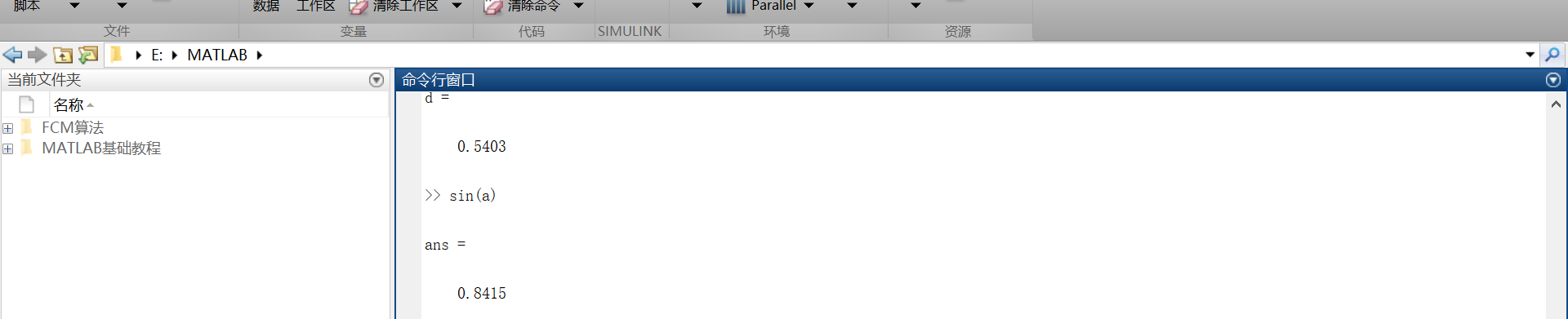3.如果语句以分号结束，表示MATLAB内部会执行这一运算，但是命令行不对计算结果进行输出。
第二部分：矩阵和数组
https://cn.mathworks.com/help/matlab/learn_matlab/matrices-and-arrays.html
大多数的编程语言一次性只能对一个数进行操作，而MATLAB主要被用来对整个矩阵或者数组进行操作。不管是什么类型的数据，所有的额MATLAB变量都是数组。矩阵是常用于线性代数的二维数组。
1.数组的创建
在一个单行中创建一个拥有四个元素的数组，其各个元素之间用逗号或者空格隔开。  例如：  a = [1 2 3 4]  这种数组叫做单行矩阵（行向量）。创建一个多行矩阵，其各个行之间用分号隔开。  例如：  b = [1,2;3,4;5,6]还可以用函数来创建矩阵。  例如：创建一个5行1列每一个元素都是0的列向量。  z = zero(5,1)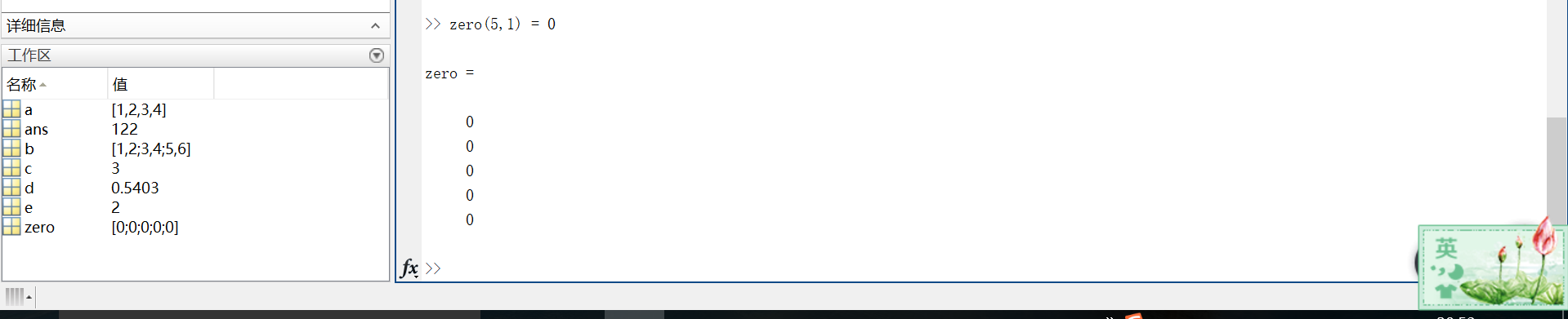2.矩阵和数组的操作
在MATLAB中，允许用一个单一的运算或者函数对矩阵中所有的数据进行处理（操作）。
例如：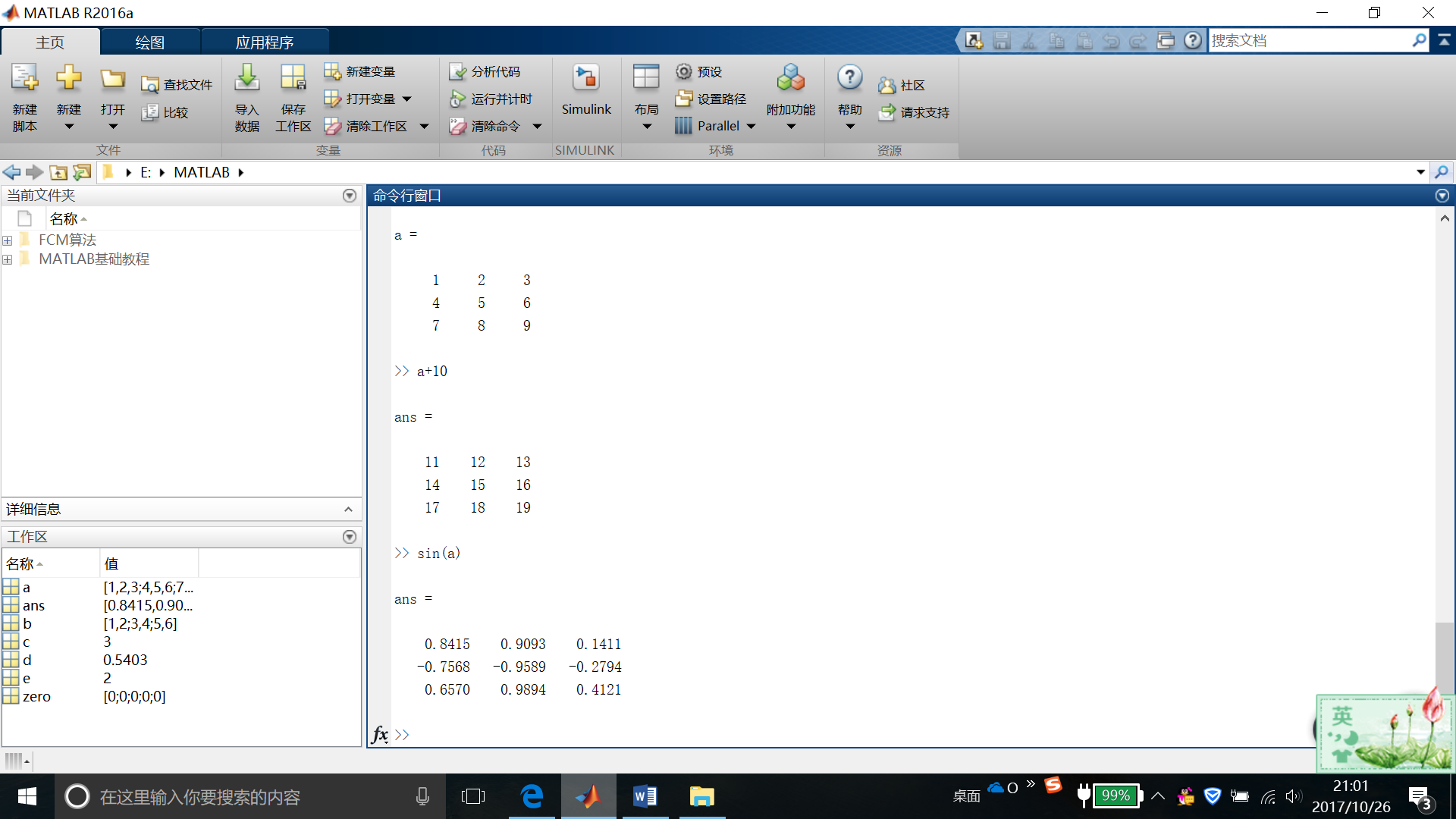如果要对矩阵进行转置，则使用单引号(‘)  例如：a’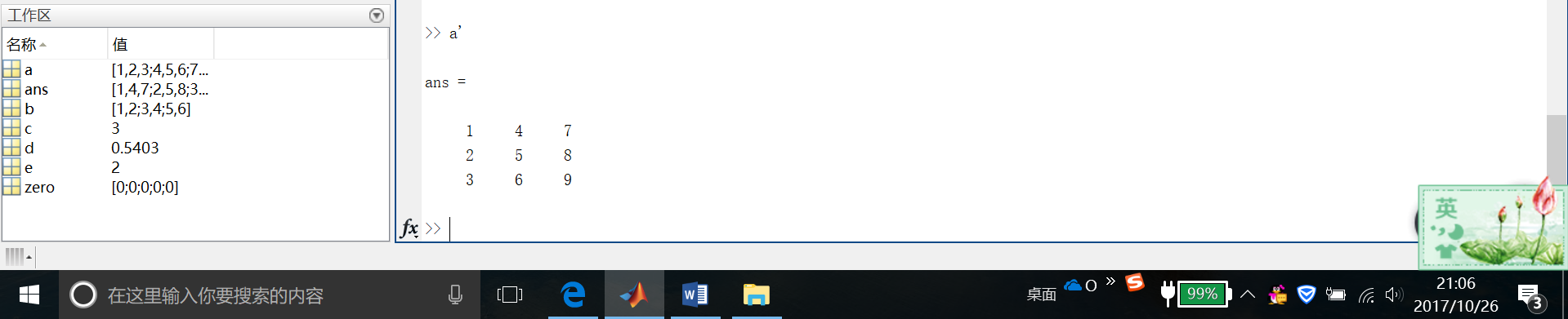可以通过执行标准矩阵乘法，通过*运算符来计算内积。
这是我自己的结果截图……很明显是不对滴~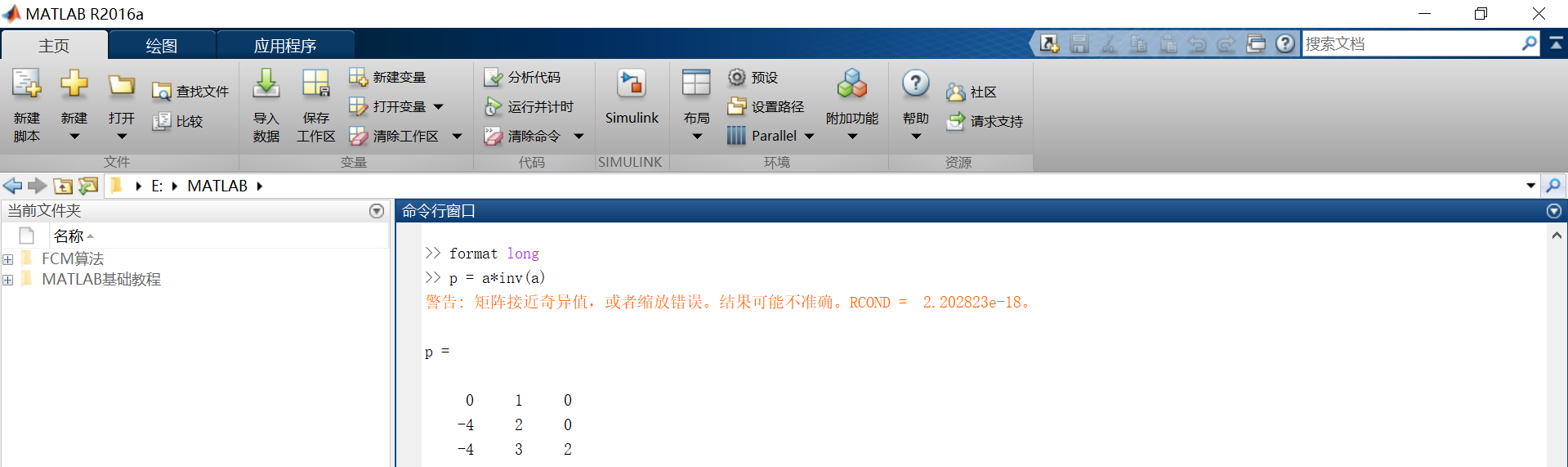然后下面是官网爸爸给的运行结果。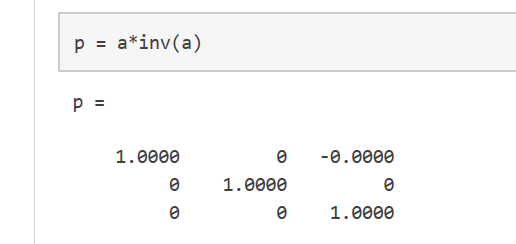我们都知道，一个矩阵与它的逆矩阵相乘的结果的确应该等于单位矩阵。至于这里为什么不对，还希望各位大佬们能不吝赐教，大恩不言谢~
这里要注意的是，p不是一个整数值矩阵，MATLAB存储数字是以浮点型存储的，真实值和它的浮点数在运算时有小小的不同，我们可以使用格式化命令，显示更多的小数位数。

比如：  format long  p = a*inv(a)

可以使用format short来重置到较短格式的使用。
x = a.*a用来表示对矩阵的每一个元素做平方操作。  若需要对每一个元素做立方运算，则用x = a.^3(如图)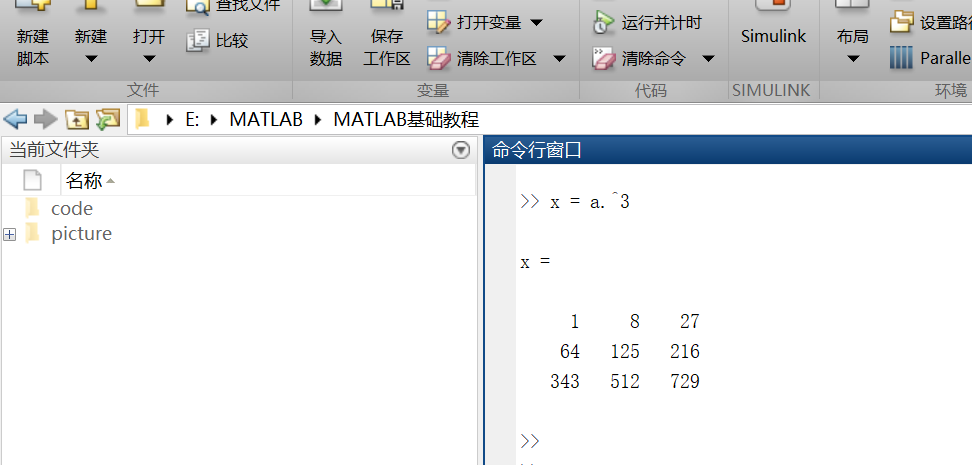矩阵的扩充  行方向上：A = [a,a]  列方向上：A = [a; a]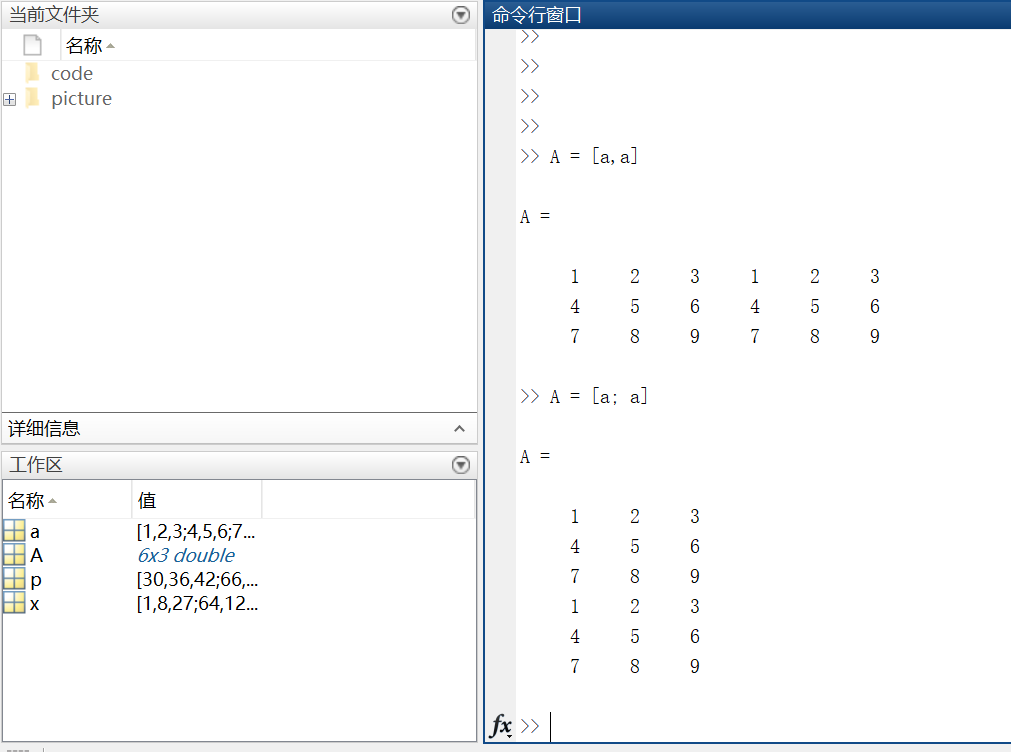复数  复数由实部和虚部组成，它的虚部是-1的平方根。  即sqrt(-1) = 0.0000 + 1.0000i  一般用i或者j来代表复数的虚部部分。
第三部分：数组的索引
https://cn.mathworks.com/help/matlab/learn_matlab/array-indexing.html
MATLAB中的每一个变量都是可以存储多个值的数组。如果想要使用数组中的某一个值，则需要用到索引。
1.单个元素的索引
从一个数组中索引某个元素一般有两种方法：  第一种比较常见：数组名加要索引的元素所在的行和列，例如A(2，4) = 8。  第二种不太常用：数组名加一个下标，该下标按列遍历。A(14) = 8。【这里不太好表达，上图吧~】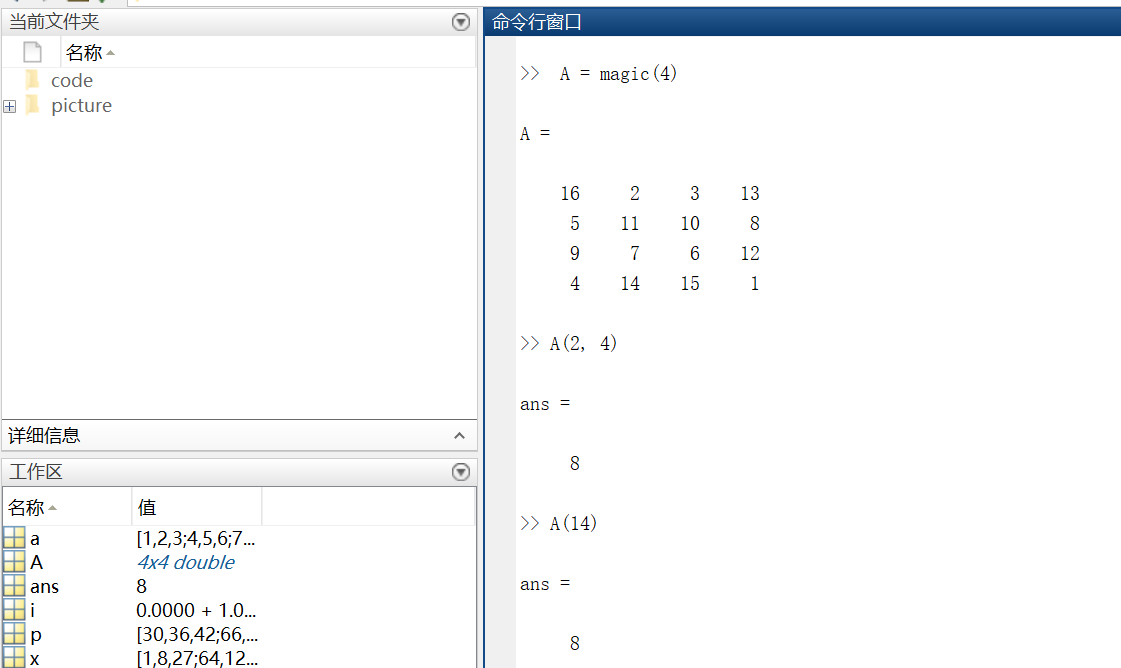再多说一句，其实A(14)中的14表示按列数第14个元素！  利用下标索引元素这种方式叫做线性索引。  注意：如果索引超出矩阵维度，MATLAB会报错。
2.多个元素的索引  引用数组的多个元素，使用冒号操作符m : n(m，n分别表示索引开始和结束的范围)
例1索引第二列第1个元素到第3个元素。  例2索引第三行全部元素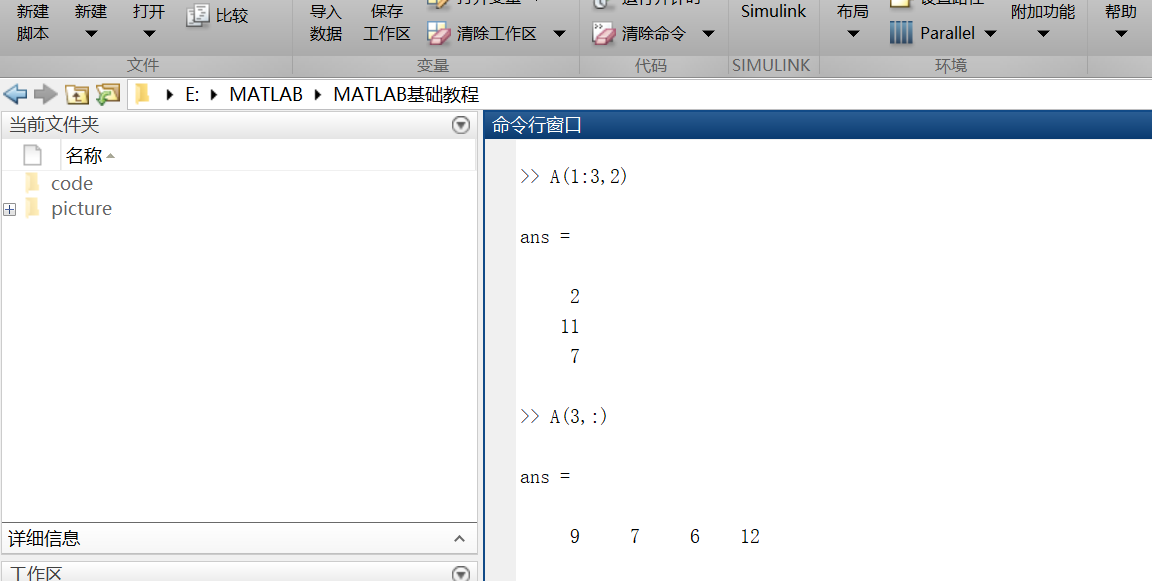不难发现，索引行则将行数放在逗号前；索引列要将列数放在逗号后。
另外，冒号运算符也可以帮助创建一组等距的矢量值，例如：  B = 0:10:100  —->B = 0 10 20 30 40 50 60 70 80 90 100  输出一个从0到100步长为10的数组。
第四部分：工作空间变量
https://cn.mathworks.com/help/matlab/learn_matlab/workspace.html
工作区包含你创建的变量或者从数据文件或其他程序导入到MATLAB中的变量。例如，我现在创建两个变量 ：  A = magic(4)  B = rand(3,5,2)
我们可以通过whos来看工作空间的内容。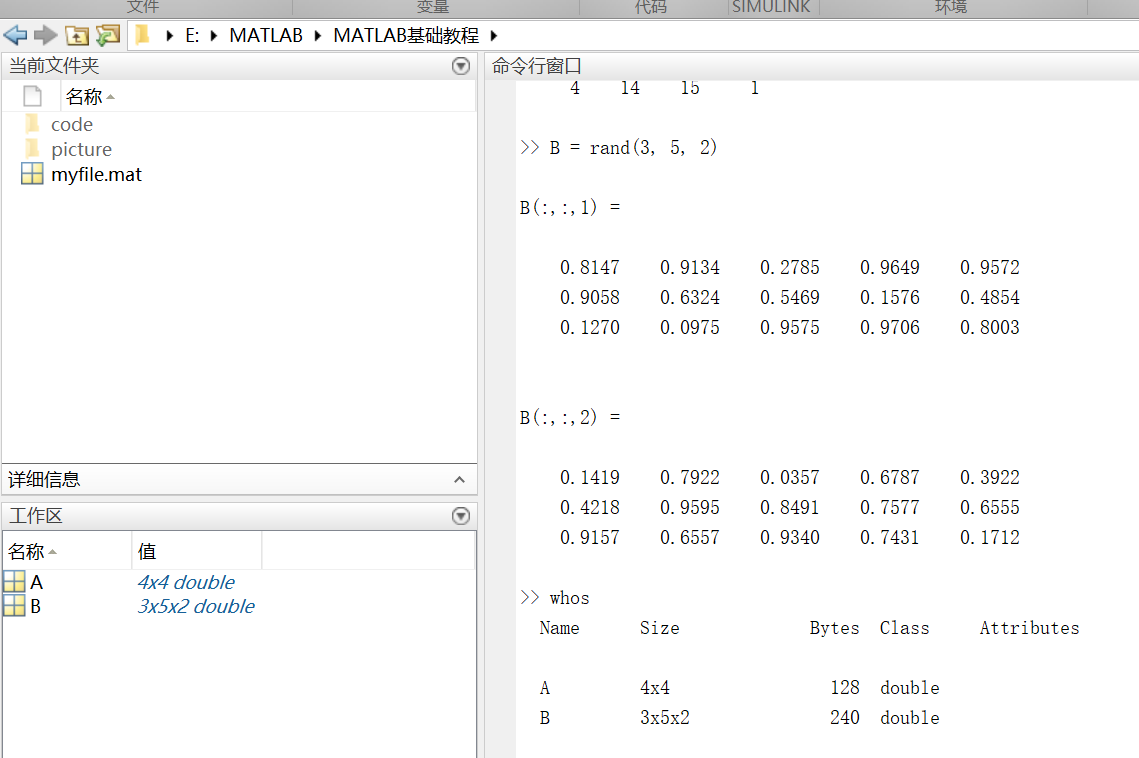第五部分：文本和字符
https://cn.mathworks.com/help/matlab/learn_matlab/character-strings.html
在处理文本时，将其中的字符序列用单引号括起来，可以将文本分配给变量。  例如：myText = ‘Hello, world’;如果文本本身包括一个单引号，则在定义时使用两个单引号。  例如：otherText = ‘You”re right’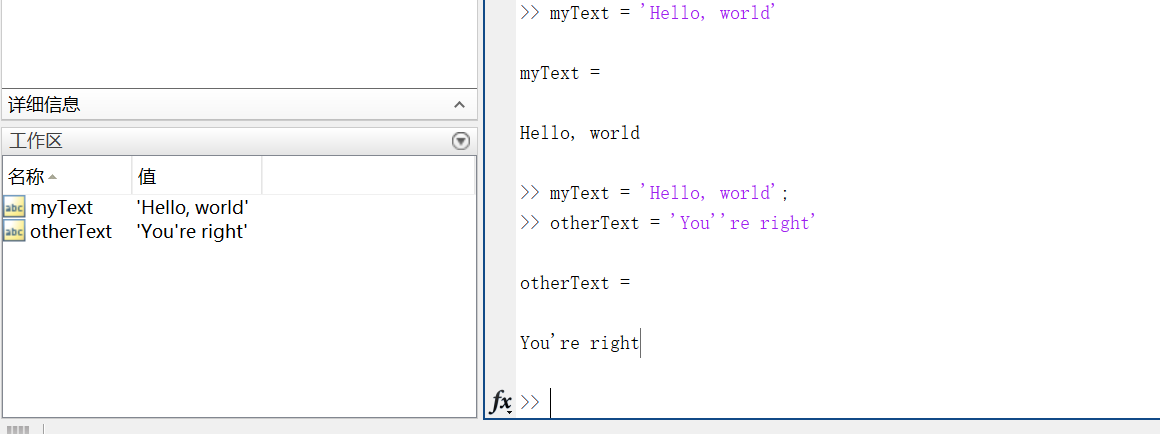同所有的MATLAB变量一样，上述创建的两个变量也都是数组，他们的数据类型是字符型。注意：字符数组也可以像数字数组那样用方括号括起来表示。
要将数字转换成字符，就要用到函数。例如num2str()或者int2str()。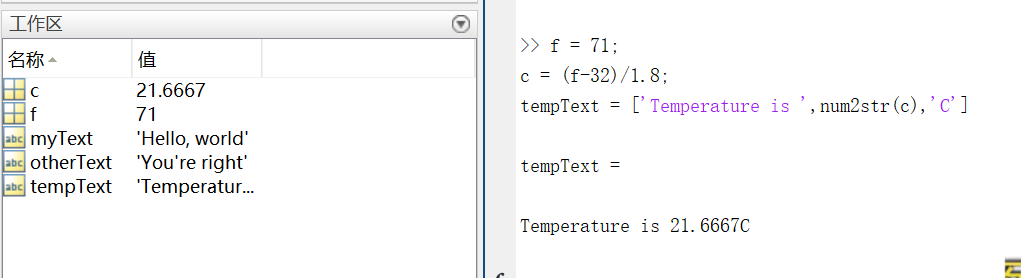第六部分：函数调用
https://cn.mathworks.com/help/matlab/learn_matlab/calling-functions.html
MATLAB提供了大量函数来执行计算任务，函数相当于其他编程语言中的子程序或方法。  例如求最大值的函数，max()。
将输入参数用括号括起来，如果有多个参数，则将参数用逗号隔开。  一般通过将函数值赋给某个变量这种方式来返回（输出）函数值，如果有多个输出参数，则需将它们用方括号括起来。  例如：  A = [1 3 5];  [maxA,location] = max(A)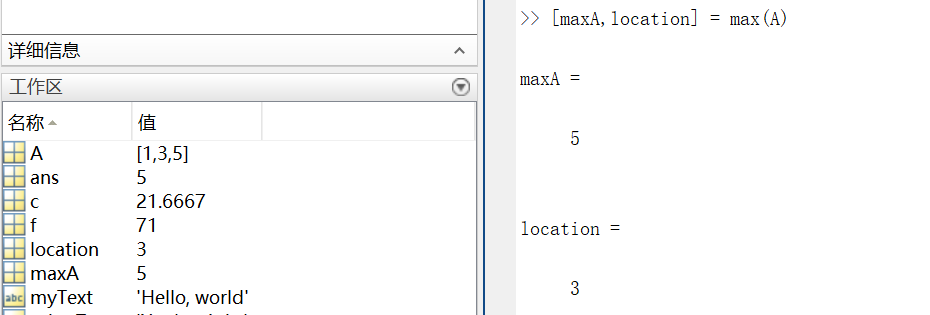可以用单引号括住任何字符进行输入。  例如：  disp(‘hello world’)  ===>输出 hello world

disp()用来显示变量的内容,括号里可以是字符,结构体,矩阵等等，类似于c语言的printf()。

clc函数被用来清空命令行。
第七部分：二、三维图
https://cn.mathworks.com/help/matlab/learn_matlab/plots.html
折线图 （1） 画图 x = 0:pi/1000:2*pi;  y = sin(x);  plot(x,y)
这里x就用到了前面说到的索引。x表示0到2*pi之间步长为pi/100的值。  二维图如下：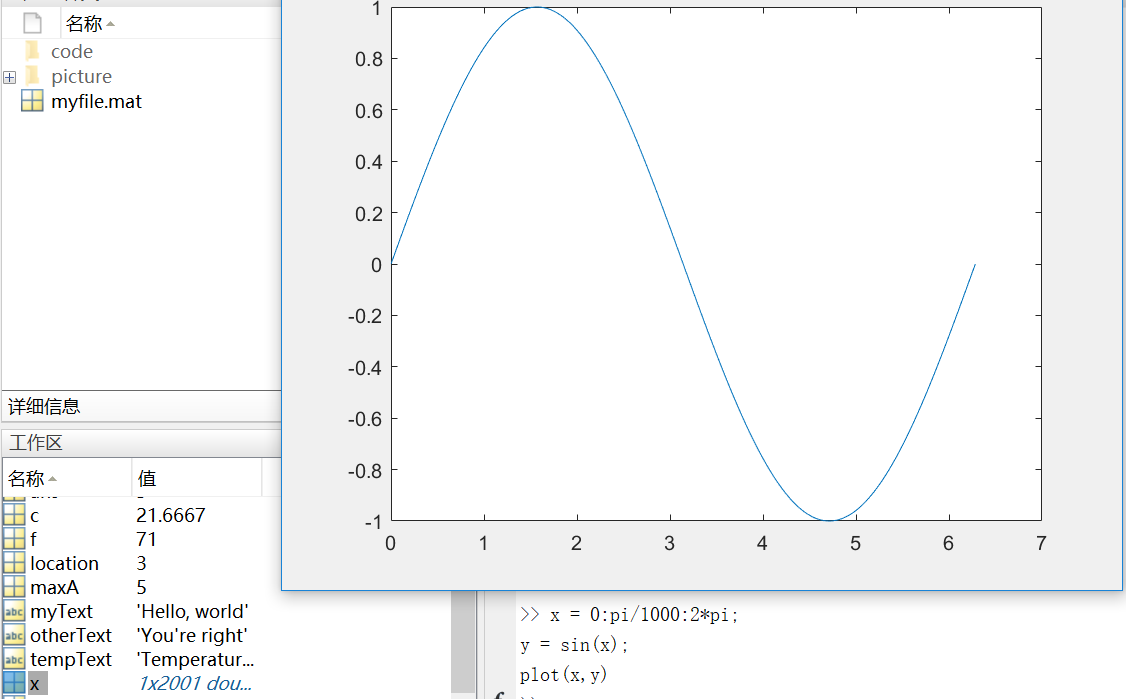这里多说一下步长，步长决定了横轴取值的密集程度。下图是将步长改为pi/10之后的图像。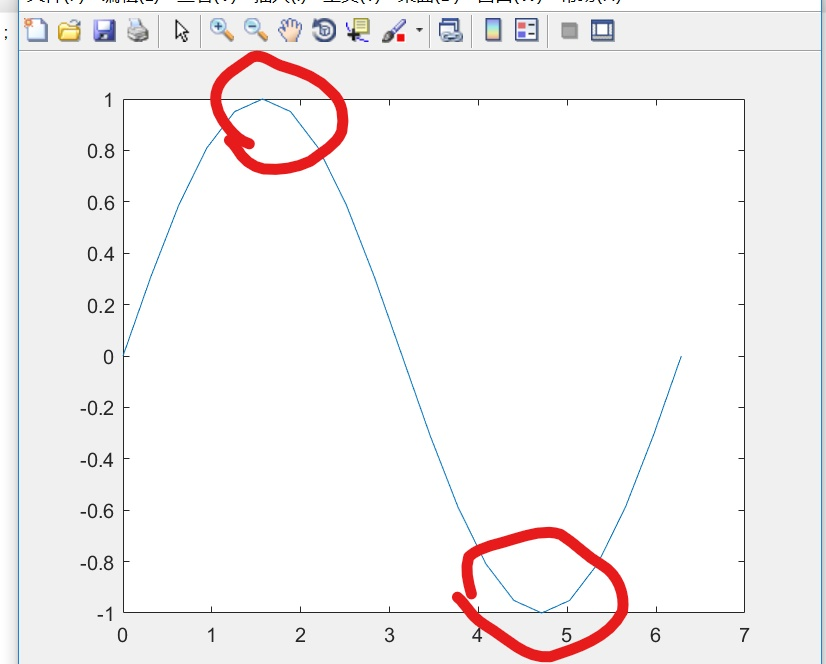对比图中标记处不难发现，步长增大，图像的精确度变低，所以这里尽量保证步长足够小，使得x可以取到足够多的值
（2） 坐标  我们可以对横轴和纵轴分别命名，并对上述正弦图像加上标题。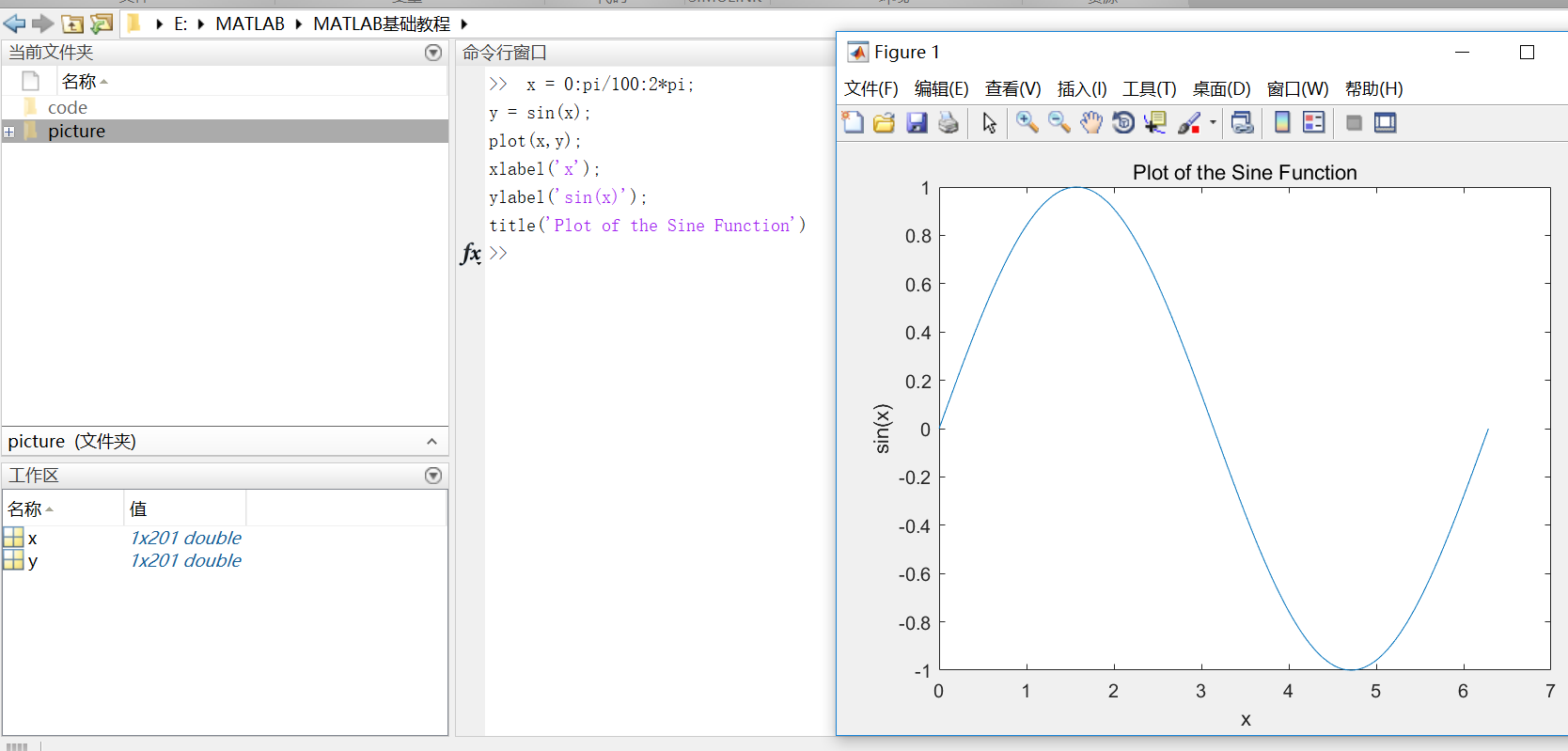（3）plot(x, y, ‘r–’)  通过在图像函数中添加第三个输入参数，可以用红色虚线绘制与原来相同的变量。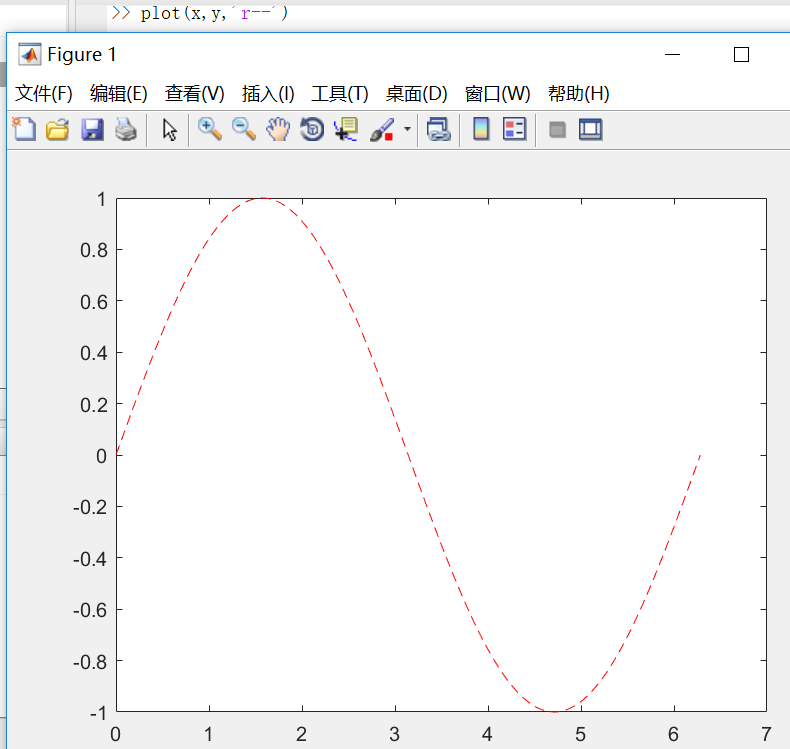其中第三个参数规定了线条的颜色、样式和标记的字符。（例如上例中的‘r–’表示红色虚线。）标记是指对每一个数据点用参数所给的标记形式进行表示。（例如’g:o’表示用‘o’连成一条绿色的线输出=.=我这个解释没谁了，讲不清楚还是上图吧…..）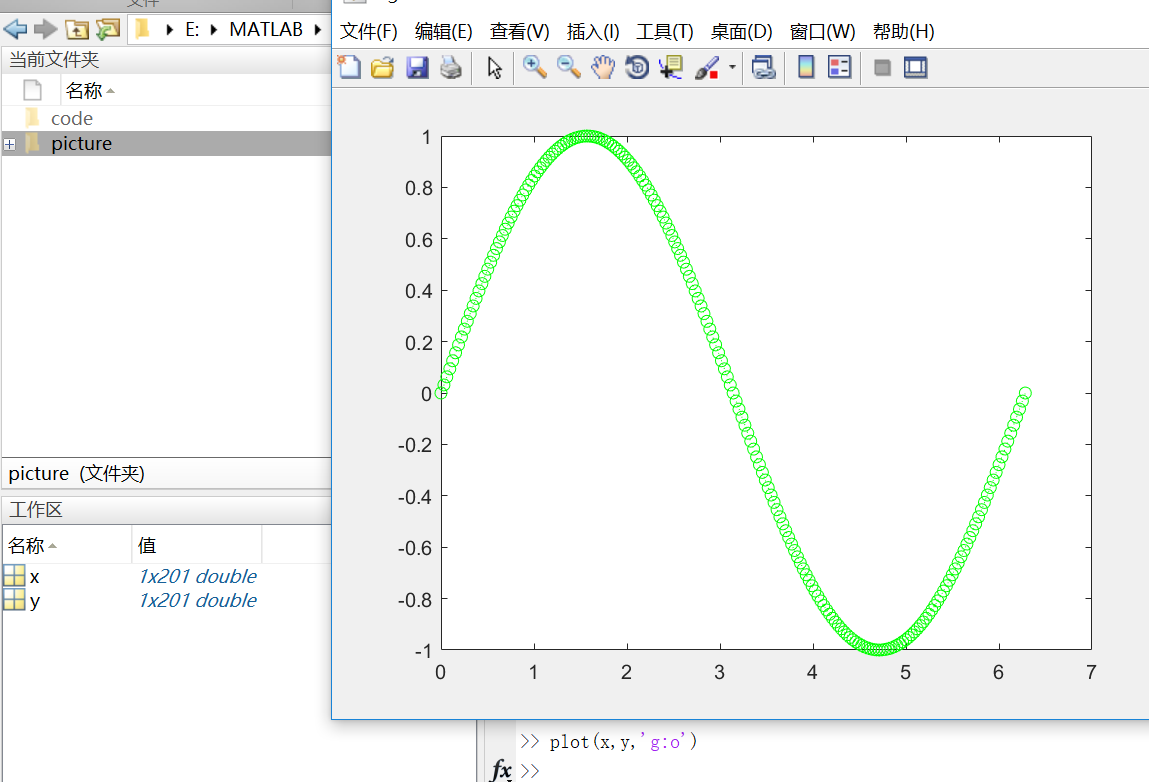这样的标记除了’o’，还有‘+’，‘*’等等。
这时候你会发现，我们开始为第一个图定义的标题和标签不再显示在当前图形窗口中了。这是因为在默认情况下，重新调用一个绘图函数，MATLAB就会清除数据，重置轴和其他元素来准备新的绘图。  若要将图添加到现有图形，使用hold命令即可。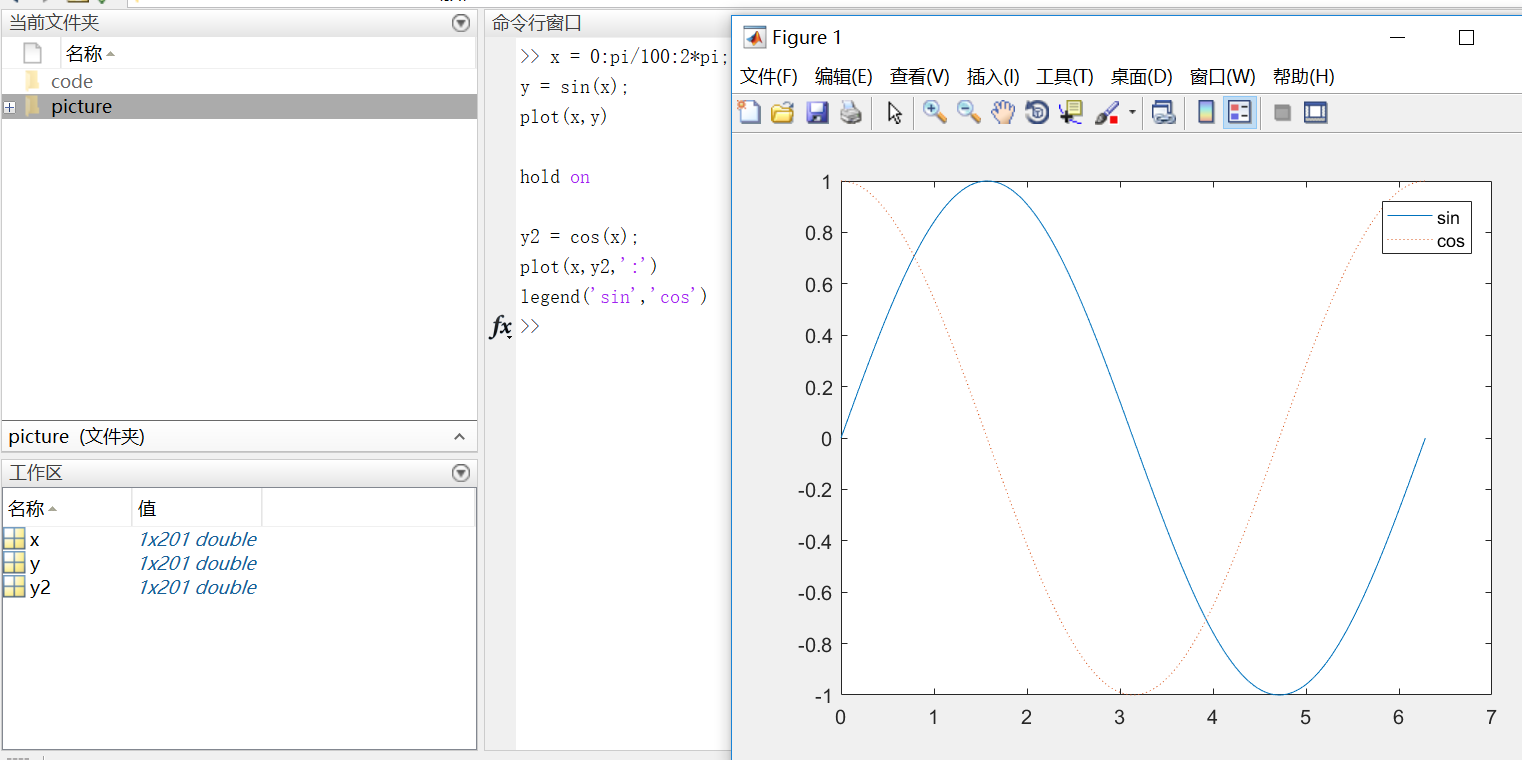在使用hold off或close the window命令之前，所有的图都会出现在当前的图形窗口中。
3D图像 3D图像通常用有两个参数的函数来定义一个曲面，z = f(x,y) 。  对于z，首先使用meshgrid命令在函数定义域内创建若干(x,y)。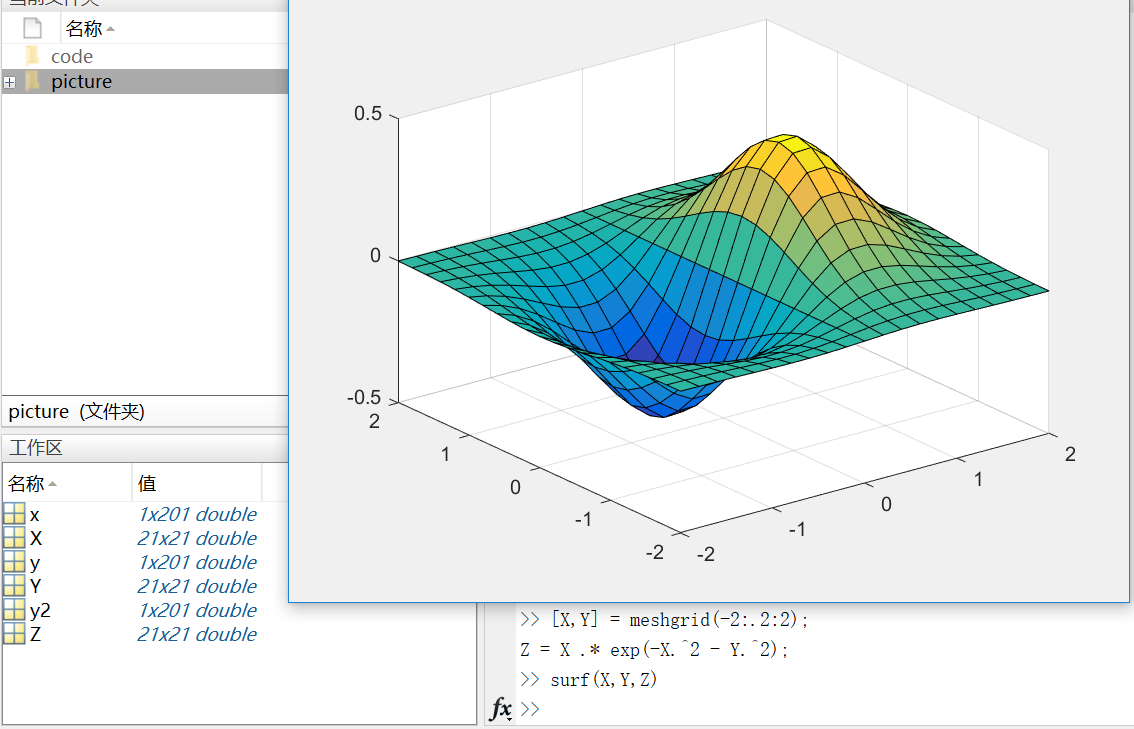这里需要注意的是，在matlab中，.2代表0.2  (-2:.2:2)表示在-2到2的区间上以0.2的长度为间隔的向量；
当变量x是由向量、数组或隔开的点组成（如x=0：0.1：1）时，则后面有x变量的方程要加点 (.)，例如：y=x.*exp(-x.*2)  但是如果x是连续的变量，如syms x，则方程写成y=x*exp(-x*2)。
Subplots  你可以用subplot函数在同一窗口的不同区域显示多个图像。  subplots函数的前两个输入参数显示当前窗口每行每列的图像数量，第三个输入参数指定活动区域。例如，在图形窗口创建四个区域来分别输出图像。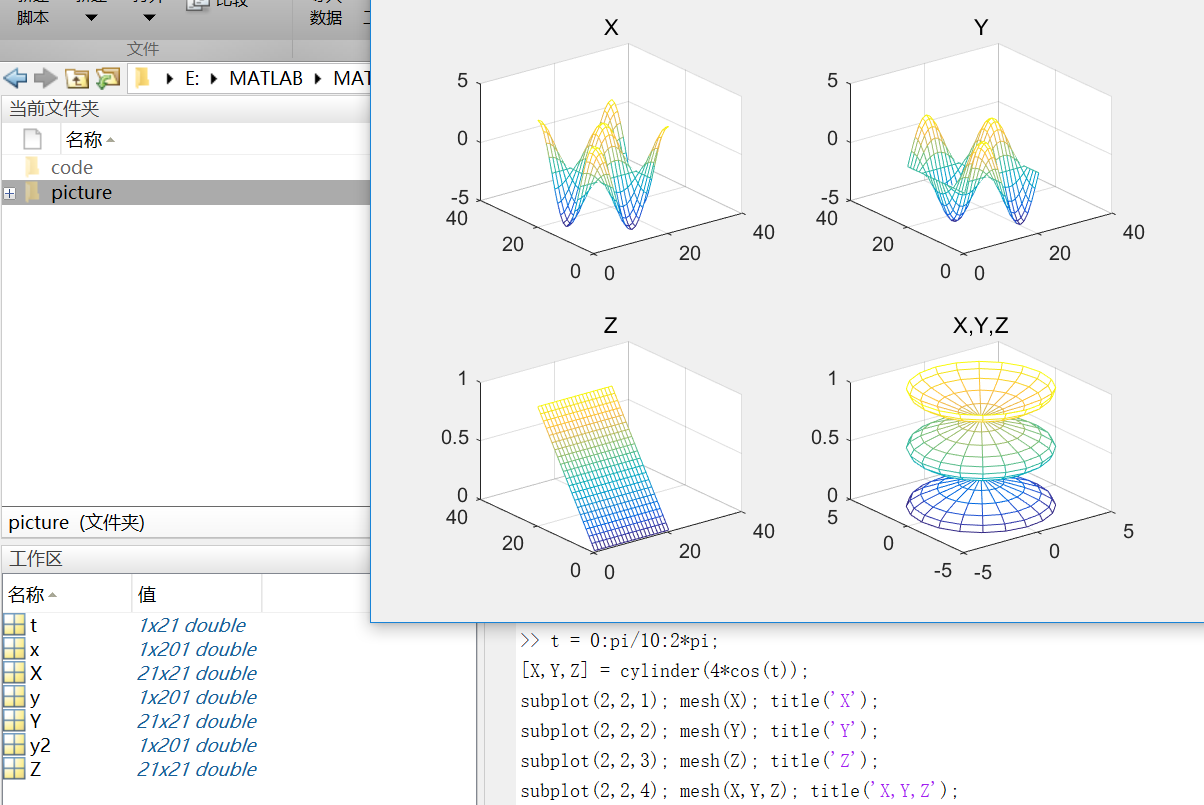mesh()生成由X，Y和Z指定的网线面，由C指定的颜色的三维网格图。
第八部分：程序和脚本
https://cn.mathworks.com/help/matlab/learn_matlab/scripts.html
示例脚本（从均匀分布中生成随机数据并计算平均值。绘制数据和平均值。）  用edit命令来创建脚本：  edit plotrand  这样就打开了一个叫plotrand.m的空文件，输入产生随机数向量的代码：
n = 50;
r = rand(n,1);
plot(r)

然后，添加代码在图中绘制水平线：
m = mean(r);
hold on
plot([0,n],[m,m])
hold off
title('Mean of Random Uniform Data')

在写代码过程中，可用（%）在代码之间添加注释。  最后保存脚本，在命令行窗口输入plotrand运行脚本。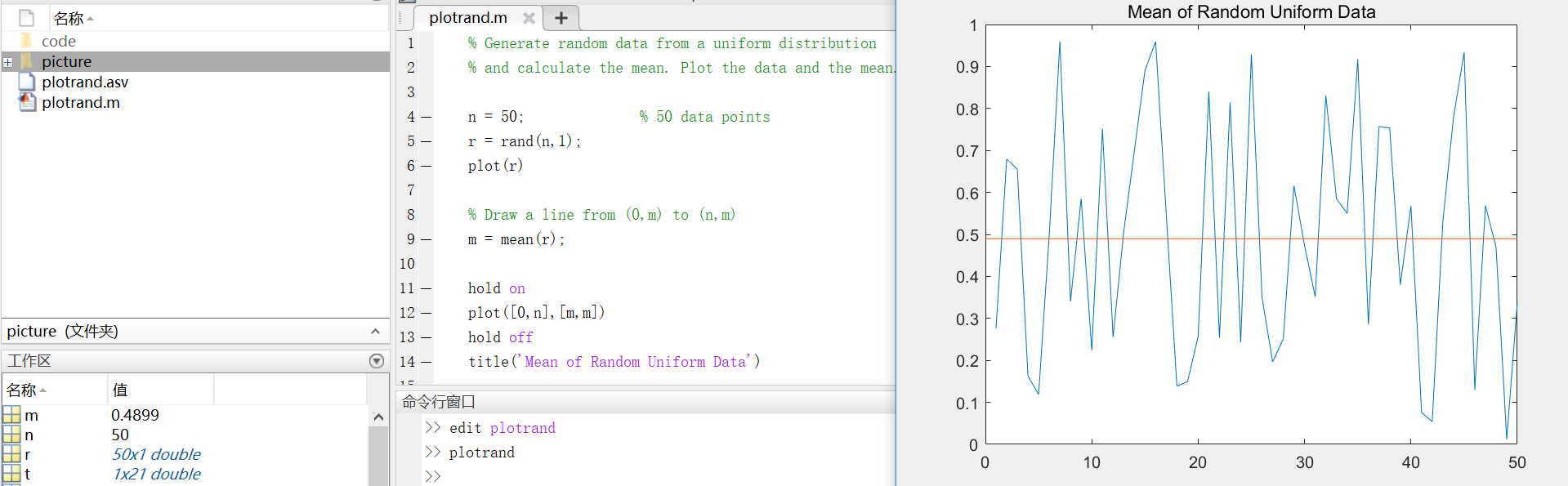循环和条件语句  在脚本中，可以循环使用部分代码，并且通过使用（for,while,if,switch等关键字）来执行这部分代码。  例如，创建一个叫calcmean.m的脚本，利用for循环计算五个随机样本的平均数和总平均数。
nsamples = 5;
npoints = 50;

for k = 1:nsamples
currentData = rand(npoints,1);
sampleMean(k) = mean(currentData);
end
overallMean = mean(sampleMean)
现在，修改for循环，以便每次迭代时都可以查看结果。在运行脚本时，会先显示中间结果，再计算总体均值。
for k = 1:nsamples
iterationString = ['Iteration #',int2str(k)];
disp(iterationString)
currentData = rand(npoints,1);
sampleMean(k) = mean(currentData)
end
overallMean = mean(sampleMean)

calcmean
在编辑器中，在calcmean.m的最后添加条件语句，使它根据不同的总体均值返回不同的信息。
if overallMean < .49
disp('Mean is less than expected')
elseif overallMean > .51
disp('Mean is greater than expected')
else
disp('Mean is within the expected range')
end
脚本位置  MATLAB中，要运行脚本，文件必须位于当前文件夹或搜索路径中的文件夹中。  默认情况下，MATLAB安装程序创建的MATLAB文件夹位于搜索路径上。如果要在另一个文件夹中存储和运行程序，需要将其添加到搜索路径中：在当前文件夹浏览器中选择文件夹，右键单击，然后选择“添加到路径”。
第九部分：帮助和文档
https://cn.mathworks.com/help/matlab/learn_matlab/help.html
使用doc命令在单独的窗口中打开函数文档。 doc mean键入左圆括号后暂停，MATLAB在命令窗口中就会显示函数提示（函数文档的语法部分）。 mean(使用帮助命令后，在命令窗口中查看函数的简单用法概括。 help mean
以上就是MATLAB的基础入门知识，欢迎指正呦~
展开全文matlab
•  提高开关频率的好处很明显，但也有些缺点，设计人员应了解其中的得失利弊，才能选择最合适的开关频率来加以... 开关频率很高的直流电源转换器(DC/DC) 正逐渐流行，因为它们可以藉由较小的输出电容和电感，进而...
• ## Python内置函数

万次阅读 多人点赞 2019-05-10 15:10:36
如果 source 为字符串，则按照指定的 encoding 将字符串转换为字节序列； 如果 source 为可迭代类型，则元素必须为[0 ,255] 中的整数； 如果 source 为与 buffer 接口一致的对象，则此对象也可以被用于初始化 ...Python内置函数
• 优点：具有任意复杂的模式分类能力和优良的多维函数映射能力，解决了简单感知不能解决的异或或者一些其他的问题。从结构上讲，BP神经网络具有输入层、隐含层和输出层；从本质上讲，BP算法就是以网络误差平方目标...BP神经网络 神经网络公式推导 神经网络
• OnePerson虽然存储的是Person类对象地址，但不是C中的指针，不能象指针那样可以进行加减运算，也不能转换为其它类型地址，它是引用型变量，只能引用(代表)Person对象，具体意义参见以后章节。和C、C++不同，C#只能用...c#
• ## XGBoost

千次阅读 多人点赞 2019-06-25 00:53:07
本文借鉴自多篇博客，非原创。 XGBoost是机器学习领域最近几年比较火热的一种十分强大的集成学习方法...Boosting是一类可将弱学习提升为强学习的集成学习算法。集成学习主要分为两大类，第一类是以Boosting...XGBoost
• c. 该程序要有顾客购票，查询班机起飞降落时间，班机订票情况等3个功能，并实现菜单选项 5、 用C++编写一个简单的行编辑，每个结点保存一行文本，程序以E file开始，然后显示行数和提示符，如果输入I,后面...
• ## 基于MATLAB的语音信号处理

万次阅读 多人点赞 2018-07-15 01:21:20
这种防混叠滤波通常与模数转换器做在一个集成块内，因此目前来说，语音信号的数字化的质量还是有保证的。市面上购买到的普通声卡在这方面做的都很好，语音声波通过话筒输入到声卡后直接获得的是经过防混叠滤波、A/D...MatLab
• ## 支持向量机

千次阅读 多人点赞 2016-07-18 23:04:15
最优间隔分类与支持向量 函数间隔和几何间隔 如何确定这个超平面 最大间隔划分超平面 对偶问题 对偶问题转化 对偶问题求解 拉格朗日乘子法和KKT条件 拉格朗日乘子法等式约束问题 等式约束问题的合理性 拉格朗日...支持向量机
• //存放转换后的灰度像素值 for ( int i= 0 ;i;i++) { uchar b=(uchar)colorImage->imageData[ 3 *i+ 0 ]; uchar g = (uchar)colorImage->imageData[ 3 * i + 1 ]; uchar r = (uchar)colorImage->...图像分割
• A/D转换器的品种繁多、性能各异，在设计数据采集系统时，首先碰到的就是如何选择合适的A/D转换器以满足系统设计要求的问题。选择A/D转换器件需要考虑器件本身的品质和应用的场合要求，基本上，可以根据以下几个方面...传感器 单片机 信号处理...